# NAME

Examples - Excel::Writer::XLSX example programs.

# DESCRIPTION

This is a documentation only module showing the examples that are included in the Excel::Writer::XLSX distribution.

This file was auto-generated via the gen_examples_pod.pl program that is also included in the examples directory.

# Example programs

The following is a list of the 91 example programs that are included in the Excel::Writer::XLSX distribution.

## Example: a_simple.pl

A simple example of how to use the Excel::Writer::XLSX module to write text and numbers to an Excel xlsx file.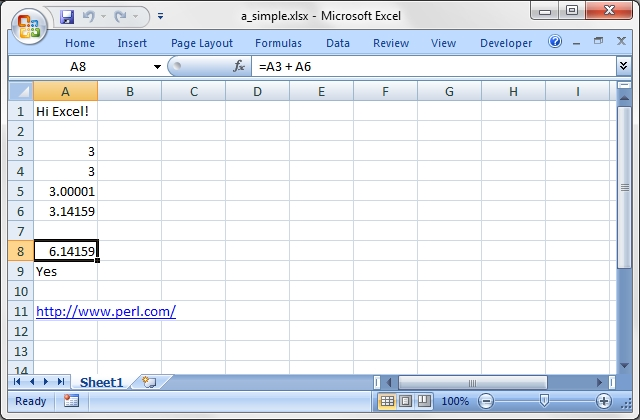Source code for this example:

``````    #!/usr/bin/perl -w

#######################################################################
#
# A simple example of how to use the Excel::Writer::XLSX module to
# write text and numbers to an Excel xlsx file.
#
# Copyright 2000-2021, John McNamara, jmcnamara@cpan.org
#

use strict;
use Excel::Writer::XLSX;

# Create a new workbook called simple.xls and add a worksheet
my \$workbook  = Excel::Writer::XLSX->new( 'a_simple.xlsx' );
my \$worksheet = \$workbook->add_worksheet();

# The general syntax is write(\$row, \$column, \$token). Note that row and
# column are zero indexed
#

# Write some text
\$worksheet->write( 0, 0, "Hi Excel!" );

# Write some numbers
\$worksheet->write( 2, 0, 3 );          # Writes 3
\$worksheet->write( 3, 0, 3.00000 );    # Writes 3
\$worksheet->write( 4, 0, 3.00001 );    # Writes 3.00001
\$worksheet->write( 5, 0, 3.14159 );    # TeX revision no.?

# Write some formulas
\$worksheet->write( 7, 0, '=A3 + A6' );
\$worksheet->write( 8, 0, '=IF(A5>3,"Yes", "No")' );

# Write a hyperlink
\$worksheet->write( 10, 0, 'http://www.perl.com/' );

\$workbook->close();

__END__``````

## Example: bug_report.pl

A template for submitting a bug report.

Run this program and read the output from the command line.

``````    #!/usr/bin/perl -w

###############################################################################
#
# A template for submitting a bug report.
#
# Run this program and read the output from the command line.
#
# Copyright 2000-2021, John McNamara, jmcnamara@cpan.org
#

use strict;

print << 'HINTS_1';

REPORTING A BUG OR ASKING A QUESTION

Feel free to report bugs or ask questions. However, to save time
consider the following steps first:

The Excel::Writer::XLSX documentation has been refined in
response to user questions. Therefore, if you have a question it is
possible that someone else has asked it before you and that it is
already addressed in the documentation. Since there is a lot of
documentation to get through you should at least read the table of
contents and search for keywords that you are interested in.

Look at the example programs:

There are over 80 example programs shipped with the standard
Excel::Writer::XLSX distribution. Many of these were created
in response to user questions. Try to identify an example program
that corresponds to your query and adapt it to your needs.

HINTS_1
print "Press enter ..."; <STDIN>;

print << 'HINTS_2';

If you submit a bug report here are some pointers.

1.  Put "Excel::Writer::XLSX:" at the beginning of the subject line.
This helps to filter genuine messages from spam.

2.  Describe the problems as clearly and as concisely as possible.

3.  Send a sample program. It is often easier to describe a problem in
code than in written prose.

4.  The sample program should be as small as possible to demonstrate the
problem. Don't copy and past large sections of your program. The
program should also be self contained and working.

A sample bug report is generated below. If you use this format then it
will help to analyse your question and respond to it more quickly.

Please don't send patches without contacting the author first.

HINTS_2
print "Press enter ..."; <STDIN>;

print << 'EMAIL';

=======================================================================

To:      John McNamara <jmcnamara@cpan.org>
Subject: Excel::Writer::XLSX: Problem with something.

Hi John,

I am using Excel::Writer::XLSX and I have encountered a problem. I
want it to do SOMETHING but the module appears to do SOMETHING_ELSE.

Here is some code that demonstrates the problem.

#!/usr/bin/perl -w

use strict;
use Excel::Writer::XLSX;

my \$workbook  = Excel::Writer::XLSX->new("reload.xls");
my \$worksheet = \$workbook->add_worksheet();

\$worksheet->write(0, 0, "Hi Excel!");

\$workbook->close();

__END__

My automatically generated system details are as follows:
EMAIL

print "\n    Perl version   : \$]";
print "\n    OS name        : \$^O";
print "\n    Module versions: (not all are required)\n";

my @modules = qw(
Excel::Writer::XLSX
Archive::Zip
XML::Writer
IO::File
File::Temp
);

for my \$module (@modules) {
my \$version;
eval "require \$module";

if (not \$@) {
\$version = \$module->VERSION;
\$version = '(unknown)' if not defined \$version;
}
else {
\$version = '(not installed)';
}

printf "%21s%-24s\t%s\n", "", \$module, \$version;
}

print << "BYE";
Yours etc.,

A. Person
--

BYE

__END__``````

## Example: demo.pl

A simple demo of some of the features of Excel::Writer::XLSX.

This program is used to create the project screenshot for Freshmeat: http://freshmeat.net/projects/writeexcel/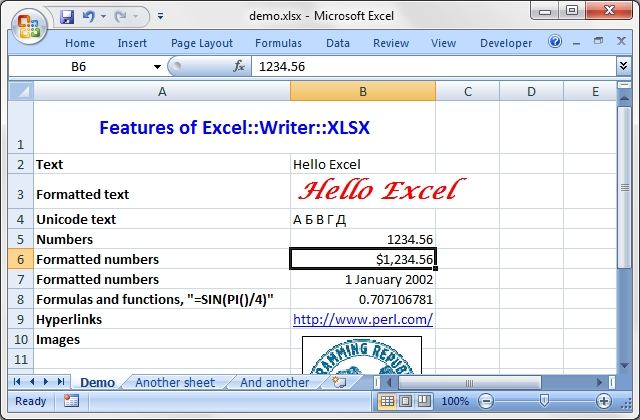Source code for this example:

``````    #!/usr/bin/perl -w

#######################################################################
#
# A simple demo of some of the features of Excel::Writer::XLSX.
#
# This program is used to create the project screenshot for Freshmeat:
# L<http://freshmeat.net/projects/writeexcel/>
#
# Copyright 2000-2021, John McNamara, jmcnamara@cpan.org
#

use strict;
use Excel::Writer::XLSX;

my \$workbook   = Excel::Writer::XLSX->new( 'demo.xlsx' );
my \$worksheet  = \$workbook->add_worksheet( 'Demo' );
my \$worksheet2 = \$workbook->add_worksheet( 'Another sheet' );
my \$worksheet3 = \$workbook->add_worksheet( 'And another' );

my \$bold = \$workbook->add_format( bold => 1 );

#######################################################################
#
# Write a general heading
#
\$worksheet->set_column( 'A:A', 36, \$bold );
\$worksheet->set_column( 'B:B', 20 );
\$worksheet->set_row( 0, 40 );

bold  => 1,
color => 'blue',
size  => 16,
merge => 1,
align => 'vcenter',
);

my @headings = ( 'Features of Excel::Writer::XLSX', '' );

#######################################################################
#
# Some text examples
#
my \$text_format = \$workbook->add_format(
bold   => 1,
italic => 1,
color  => 'red',
size   => 18,
font   => 'Lucida Calligraphy'
);

\$worksheet->write( 'A2', "Text" );
\$worksheet->write( 'B2', "Hello Excel" );
\$worksheet->write( 'A3', "Formatted text" );
\$worksheet->write( 'B3', "Hello Excel", \$text_format );
\$worksheet->write( 'A4', "Unicode text" );
\$worksheet->write( 'B4', "\x{0410} \x{0411} \x{0412} \x{0413} \x{0414}" );

#######################################################################
#
# Some numeric examples
#
my \$num1_format = \$workbook->add_format( num_format => '\$#,##0.00' );
my \$num2_format = \$workbook->add_format( num_format => ' d mmmm yyy' );

\$worksheet->write( 'A5', "Numbers" );
\$worksheet->write( 'B5', 1234.56 );
\$worksheet->write( 'A6', "Formatted numbers" );
\$worksheet->write( 'B6', 1234.56, \$num1_format );
\$worksheet->write( 'A7', "Formatted numbers" );
\$worksheet->write( 'B7', 37257, \$num2_format );

#######################################################################
#
# Formulae
#
\$worksheet->set_selection( 'B8' );
\$worksheet->write( 'A8', 'Formulas and functions, "=SIN(PI()/4)"' );
\$worksheet->write( 'B8', '=SIN(PI()/4)' );

#######################################################################
#
#
\$worksheet->write( 'A9', "Hyperlinks" );
\$worksheet->write( 'B9', 'http://www.perl.com/' );

#######################################################################
#
# Images
#
\$worksheet->write( 'A10', "Images" );
\$worksheet->insert_image( 'B10', 'republic.png',
{ x_offset => 16, y_offset => 8 } );

#######################################################################
#
# Misc
#
\$worksheet->write( 'A18', "Page/printer setup" );
\$worksheet->write( 'A19', "Multiple worksheets" );

\$workbook->close();

__END__``````

## Example: formats.pl

Examples of formatting using the Excel::Writer::XLSX module.

This program demonstrates almost all possible formatting options. It is worth running this program and viewing the output Excel file if you are interested in the various formatting possibilities.Source code for this example:

``````    #!/usr/bin/perl -w

###############################################################################
#
# Examples of formatting using the Excel::Writer::XLSX module.
#
# This program demonstrates almost all possible formatting options. It is worth
# running this program and viewing the output Excel file if you are interested
# in the various formatting possibilities.
#
# Copyright 2000-2021, John McNamara, jmcnamara@cpan.org
#

use strict;
use Excel::Writer::XLSX;

my \$workbook = Excel::Writer::XLSX->new( 'formats.xlsx' );

# Some common formats
my \$center = \$workbook->add_format( align => 'center' );
my \$heading = \$workbook->add_format( align => 'center', bold => 1 );

# The named colors
my %colors = (
0x08, 'black',
0x0C, 'blue',
0x10, 'brown',
0x0F, 'cyan',
0x17, 'gray',
0x11, 'green',
0x0B, 'lime',
0x0E, 'magenta',
0x12, 'navy',
0x35, 'orange',
0x21, 'pink',
0x14, 'purple',
0x0A, 'red',
0x16, 'silver',
0x09, 'white',
0x0D, 'yellow',

);

# Call these subroutines to demonstrate different formatting options
intro();
fonts();
named_colors();
standard_colors();
numeric_formats();
borders();
patterns();
alignment();
misc();

# Note: this is required
\$workbook->close();

######################################################################
#
# Intro.
#
sub intro {

my \$worksheet = \$workbook->add_worksheet( 'Introduction' );

\$worksheet->set_column( 0, 0, 60 );

my \$format = \$workbook->add_format();
\$format->set_bold();
\$format->set_size( 14 );
\$format->set_color( 'blue' );
\$format->set_align( 'center' );

my \$format2 = \$workbook->add_format();
\$format2->set_bold();
\$format2->set_color( 'blue' );

my \$format3 = \$workbook->add_format(
color     => 'blue',
underline => 1,
);

\$worksheet->write( 2, 0, 'This workbook demonstrates some of', \$format );
\$worksheet->write( 3, 0, 'the formatting options provided by', \$format );
\$worksheet->write( 4, 0, 'the Excel::Writer::XLSX module.',    \$format );
\$worksheet->write( 'A7', 'Sections:', \$format2 );

\$worksheet->write( 'A8', "internal:Fonts!A1", 'Fonts', \$format3 );

\$worksheet->write( 'A9', "internal:'Named colors'!A1",
'Named colors', \$format3 );

\$worksheet->write(
'A10',
"internal:'Standard colors'!A1",
'Standard colors', \$format3
);

\$worksheet->write(
'A11',
"internal:'Numeric formats'!A1",
'Numeric formats', \$format3
);

\$worksheet->write( 'A12', "internal:Borders!A1", 'Borders', \$format3 );
\$worksheet->write( 'A13', "internal:Patterns!A1", 'Patterns', \$format3 );
\$worksheet->write( 'A14', "internal:Alignment!A1", 'Alignment', \$format3 );
\$worksheet->write( 'A15', "internal:Miscellaneous!A1", 'Miscellaneous',
\$format3 );

}

######################################################################
#
# Demonstrate the named colors.
#
sub named_colors {

my \$worksheet = \$workbook->add_worksheet( 'Named colors' );

\$worksheet->set_column( 0, 3, 15 );

\$worksheet->write( 0, 0, "Index", \$heading );
\$worksheet->write( 0, 1, "Index", \$heading );
\$worksheet->write( 0, 2, "Name",  \$heading );
\$worksheet->write( 0, 3, "Color", \$heading );

my \$i = 1;

while ( my ( \$index, \$color ) = each %colors ) {
my \$format = \$workbook->add_format(
bg_color => \$color,
pattern  => 1,
border   => 1
);

\$worksheet->write( \$i + 1, 0, \$index, \$center );
\$worksheet->write( \$i + 1, 1, sprintf( "0x%02X", \$index ), \$center );
\$worksheet->write( \$i + 1, 2, \$color, \$center );
\$worksheet->write( \$i + 1, 3, '',     \$format );
\$i++;
}
}

######################################################################
#
# Demonstrate the standard Excel colors in the range 8..63.
#
sub standard_colors {

my \$worksheet = \$workbook->add_worksheet( 'Standard colors' );

\$worksheet->set_column( 0, 3, 15 );

\$worksheet->write( 0, 0, "Index", \$heading );
\$worksheet->write( 0, 1, "Index", \$heading );
\$worksheet->write( 0, 2, "Color", \$heading );
\$worksheet->write( 0, 3, "Name",  \$heading );

for my \$i ( 8 .. 63 ) {
my \$format = \$workbook->add_format(
bg_color => \$i,
pattern  => 1,
border   => 1
);

\$worksheet->write( ( \$i - 7 ), 0, \$i, \$center );
\$worksheet->write( ( \$i - 7 ), 1, sprintf( "0x%02X", \$i ), \$center );
\$worksheet->write( ( \$i - 7 ), 2, '', \$format );

# Add the  color names
if ( exists \$colors{\$i} ) {
\$worksheet->write( ( \$i - 7 ), 3, \$colors{\$i}, \$center );

}
}
}

######################################################################
#
# Demonstrate the standard numeric formats.
#
sub numeric_formats {

my \$worksheet = \$workbook->add_worksheet( 'Numeric formats' );

\$worksheet->set_column( 0, 4, 15 );
\$worksheet->set_column( 5, 5, 45 );

\$worksheet->write( 0, 0, "Index",       \$heading );
\$worksheet->write( 0, 1, "Index",       \$heading );
\$worksheet->write( 0, 2, "Unformatted", \$heading );
\$worksheet->write( 0, 3, "Formatted",   \$heading );
\$worksheet->write( 0, 4, "Negative",    \$heading );
\$worksheet->write( 0, 5, "Format",      \$heading );

#<<<
my @formats;
push @formats, [ 0x00, 1234.567,   0,         'General' ];
push @formats, [ 0x01, 1234.567,   0,         '0' ];
push @formats, [ 0x02, 1234.567,   0,         '0.00' ];
push @formats, [ 0x03, 1234.567,   0,         '#,##0' ];
push @formats, [ 0x04, 1234.567,   0,         '#,##0.00' ];
push @formats, [ 0x05, 1234.567,   -1234.567, '(\$#,##0_);(\$#,##0)' ];
push @formats, [ 0x06, 1234.567,   -1234.567, '(\$#,##0_);[Red](\$#,##0)' ];
push @formats, [ 0x07, 1234.567,   -1234.567, '(\$#,##0.00_);(\$#,##0.00)' ];
push @formats, [ 0x08, 1234.567,   -1234.567, '(\$#,##0.00_);[Red](\$#,##0.00)' ];
push @formats, [ 0x09, 0.567,      0,         '0%' ];
push @formats, [ 0x0a, 0.567,      0,         '0.00%' ];
push @formats, [ 0x0b, 1234.567,   0,         '0.00E+00' ];
push @formats, [ 0x0c, 0.75,       0,         '# ?/?' ];
push @formats, [ 0x0d, 0.3125,     0,         '# ??/??' ];
push @formats, [ 0x0e, 36892.521,  0,         'm/d/yy' ];
push @formats, [ 0x0f, 36892.521,  0,         'd-mmm-yy' ];
push @formats, [ 0x10, 36892.521,  0,         'd-mmm' ];
push @formats, [ 0x11, 36892.521,  0,         'mmm-yy' ];
push @formats, [ 0x12, 36892.521,  0,         'h:mm AM/PM' ];
push @formats, [ 0x13, 36892.521,  0,         'h:mm:ss AM/PM' ];
push @formats, [ 0x14, 36892.521,  0,         'h:mm' ];
push @formats, [ 0x15, 36892.521,  0,         'h:mm:ss' ];
push @formats, [ 0x16, 36892.521,  0,         'm/d/yy h:mm' ];
push @formats, [ 0x25, 1234.567,   -1234.567, '(#,##0_);(#,##0)' ];
push @formats, [ 0x26, 1234.567,   -1234.567, '(#,##0_);[Red](#,##0)' ];
push @formats, [ 0x27, 1234.567,   -1234.567, '(#,##0.00_);(#,##0.00)' ];
push @formats, [ 0x28, 1234.567,   -1234.567, '(#,##0.00_);[Red](#,##0.00)' ];
push @formats, [ 0x29, 1234.567,   -1234.567, '_(* #,##0_);_(* (#,##0);_(* "-"_);_(@_)' ];
push @formats, [ 0x2a, 1234.567,   -1234.567, '_(\$* #,##0_);_(\$* (#,##0);_(\$* "-"_);_(@_)' ];
push @formats, [ 0x2b, 1234.567,   -1234.567, '_(* #,##0.00_);_(* (#,##0.00);_(* "-"??_);_(@_)' ];
push @formats, [ 0x2c, 1234.567,   -1234.567, '_(\$* #,##0.00_);_(\$* (#,##0.00);_(\$* "-"??_);_(@_)' ];
push @formats, [ 0x2d, 36892.521,  0,         'mm:ss' ];
push @formats, [ 0x2e, 3.0153,     0,         '[h]:mm:ss' ];
push @formats, [ 0x2f, 36892.521,  0,         'mm:ss.0' ];
push @formats, [ 0x30, 1234.567,   0,         '##0.0E+0' ];
push @formats, [ 0x31, 1234.567,   0,         '@' ];
#>>>

my \$i;
foreach my \$format ( @formats ) {
my \$style = \$workbook->add_format();
\$style->set_num_format( \$format-> );

\$i++;
\$worksheet->write( \$i, 0, \$format->, \$center );
\$worksheet->write( \$i, 1, sprintf( "0x%02X", \$format-> ), \$center );
\$worksheet->write( \$i, 2, \$format->, \$center );
\$worksheet->write( \$i, 3, \$format->, \$style );

if ( \$format-> ) {
\$worksheet->write( \$i, 4, \$format->, \$style );
}

\$worksheet->write_string( \$i, 5, \$format-> );
}
}

######################################################################
#
# Demonstrate the font options.
#
sub fonts {

my \$worksheet = \$workbook->add_worksheet( 'Fonts' );

\$worksheet->set_column( 0, 0, 30 );
\$worksheet->set_column( 1, 1, 10 );

\$worksheet->write( 0, 0, "Font name", \$heading );
\$worksheet->write( 0, 1, "Font size", \$heading );

my @fonts;
push @fonts, [ 10, 'Arial' ];
push @fonts, [ 12, 'Arial' ];
push @fonts, [ 14, 'Arial' ];
push @fonts, [ 12, 'Arial Black' ];
push @fonts, [ 12, 'Arial Narrow' ];
push @fonts, [ 12, 'Century Schoolbook' ];
push @fonts, [ 12, 'Courier' ];
push @fonts, [ 12, 'Courier New' ];
push @fonts, [ 12, 'Garamond' ];
push @fonts, [ 12, 'Impact' ];
push @fonts, [ 12, 'Lucida Handwriting' ];
push @fonts, [ 12, 'Times New Roman' ];
push @fonts, [ 12, 'Symbol' ];
push @fonts, [ 12, 'Wingdings' ];
push @fonts, [ 12, 'A font that doesn\'t exist' ];

my \$i;
foreach my \$font ( @fonts ) {
my \$format = \$workbook->add_format();

\$format->set_size( \$font-> );
\$format->set_font( \$font-> );

\$i++;
\$worksheet->write( \$i, 0, \$font->, \$format );
\$worksheet->write( \$i, 1, \$font->, \$format );
}

}

######################################################################
#
# Demonstrate the standard Excel border styles.
#
sub borders {

my \$worksheet = \$workbook->add_worksheet( 'Borders' );

\$worksheet->set_column( 0, 4, 10 );
\$worksheet->set_column( 5, 5, 40 );

\$worksheet->write( 0, 0, "Index",                                \$heading );
\$worksheet->write( 0, 1, "Index",                                \$heading );
\$worksheet->write( 0, 3, "Style",                                \$heading );
\$worksheet->write( 0, 5, "The style is highlighted in red for ", \$heading );
\$worksheet->write( 1, 5, "emphasis, the default color is black.",

for my \$i ( 0 .. 13 ) {
my \$format = \$workbook->add_format();
\$format->set_border( \$i );
\$format->set_border_color( 'red' );
\$format->set_align( 'center' );

\$worksheet->write( ( 2 * ( \$i + 1 ) ), 0, \$i, \$center );
\$worksheet->write( ( 2 * ( \$i + 1 ) ),
1, sprintf( "0x%02X", \$i ), \$center );

\$worksheet->write( ( 2 * ( \$i + 1 ) ), 3, "Border", \$format );
}

\$worksheet->write( 30, 0, "Diag type",              \$heading );
\$worksheet->write( 30, 1, "Index",                  \$heading );
\$worksheet->write( 30, 3, "Style",                  \$heading );
\$worksheet->write( 30, 5, "Diagonal Border styles", \$heading );

for my \$i ( 1 .. 3 ) {
my \$format = \$workbook->add_format();
\$format->set_diag_type( \$i );
\$format->set_diag_border( 1 );
\$format->set_diag_color( 'red' );
\$format->set_align( 'center' );

\$worksheet->write( ( 2 * ( \$i + 15 ) ), 0, \$i, \$center );
\$worksheet->write( ( 2 * ( \$i + 15 ) ),
1, sprintf( "0x%02X", \$i ), \$center );

\$worksheet->write( ( 2 * ( \$i + 15 ) ), 3, "Border", \$format );
}
}

######################################################################
#
# Demonstrate the standard Excel cell patterns.
#
sub patterns {

my \$worksheet = \$workbook->add_worksheet( 'Patterns' );

\$worksheet->set_column( 0, 4, 10 );
\$worksheet->set_column( 5, 5, 50 );

\$worksheet->write( 0, 0, "Index",   \$heading );
\$worksheet->write( 0, 1, "Index",   \$heading );
\$worksheet->write( 0, 3, "Pattern", \$heading );

\$worksheet->write( 0, 5, "The background colour has been set to silver.",
\$worksheet->write( 1, 5, "The foreground colour has been set to green.",

for my \$i ( 0 .. 18 ) {
my \$format = \$workbook->add_format();

\$format->set_pattern( \$i );
\$format->set_bg_color( 'silver' );
\$format->set_fg_color( 'green' );
\$format->set_align( 'center' );

\$worksheet->write( ( 2 * ( \$i + 1 ) ), 0, \$i, \$center );
\$worksheet->write( ( 2 * ( \$i + 1 ) ),
1, sprintf( "0x%02X", \$i ), \$center );

\$worksheet->write( ( 2 * ( \$i + 1 ) ), 3, "Pattern", \$format );

if ( \$i == 1 ) {
\$worksheet->write( ( 2 * ( \$i + 1 ) ),
5, "This is solid colour, the most useful pattern.", \$heading );
}
}
}

######################################################################
#
# Demonstrate the standard Excel cell alignments.
#
sub alignment {

my \$worksheet = \$workbook->add_worksheet( 'Alignment' );

\$worksheet->set_column( 0, 7, 12 );
\$worksheet->set_row( 0, 40 );
\$worksheet->set_selection( 7, 0 );

my \$format01 = \$workbook->add_format();
my \$format02 = \$workbook->add_format();
my \$format03 = \$workbook->add_format();
my \$format04 = \$workbook->add_format();
my \$format05 = \$workbook->add_format();
my \$format06 = \$workbook->add_format();
my \$format07 = \$workbook->add_format();
my \$format08 = \$workbook->add_format();
my \$format09 = \$workbook->add_format();
my \$format10 = \$workbook->add_format();
my \$format11 = \$workbook->add_format();
my \$format12 = \$workbook->add_format();
my \$format13 = \$workbook->add_format();
my \$format14 = \$workbook->add_format();
my \$format15 = \$workbook->add_format();
my \$format16 = \$workbook->add_format();
my \$format17 = \$workbook->add_format();

\$format02->set_align( 'top' );
\$format03->set_align( 'bottom' );
\$format04->set_align( 'vcenter' );
\$format05->set_align( 'vjustify' );
\$format06->set_text_wrap();

\$format07->set_align( 'left' );
\$format08->set_align( 'right' );
\$format09->set_align( 'center' );
\$format10->set_align( 'fill' );
\$format11->set_align( 'justify' );
\$format12->set_merge();

\$format13->set_rotation( 45 );
\$format14->set_rotation( -45 );
\$format15->set_rotation( 270 );

\$format16->set_shrink();
\$format17->set_indent( 1 );

\$worksheet->write( 0, 0, 'Vertical',   \$heading );
\$worksheet->write( 0, 1, 'top',        \$format02 );
\$worksheet->write( 0, 2, 'bottom',     \$format03 );
\$worksheet->write( 0, 3, 'vcenter',    \$format04 );
\$worksheet->write( 0, 4, 'vjustify',   \$format05 );
\$worksheet->write( 0, 5, "text\nwrap", \$format06 );

\$worksheet->write( 2, 0, 'Horizontal', \$heading );
\$worksheet->write( 2, 1, 'left',       \$format07 );
\$worksheet->write( 2, 2, 'right',      \$format08 );
\$worksheet->write( 2, 3, 'center',     \$format09 );
\$worksheet->write( 2, 4, 'fill',       \$format10 );
\$worksheet->write( 2, 5, 'justify',    \$format11 );

\$worksheet->write( 3, 1, 'merge', \$format12 );
\$worksheet->write( 3, 2, '',      \$format12 );

\$worksheet->write( 3, 3, 'Shrink ' x 3, \$format16 );
\$worksheet->write( 3, 4, 'Indent',      \$format17 );

\$worksheet->write( 5, 0, 'Rotation',   \$heading );
\$worksheet->write( 5, 1, 'Rotate 45',  \$format13 );
\$worksheet->write( 6, 1, 'Rotate -45', \$format14 );
\$worksheet->write( 7, 1, 'Rotate 270', \$format15 );
}

######################################################################
#
# Demonstrate other miscellaneous features.
#
sub misc {

my \$worksheet = \$workbook->add_worksheet( 'Miscellaneous' );

\$worksheet->set_column( 2, 2, 25 );

my \$format01 = \$workbook->add_format();
my \$format02 = \$workbook->add_format();
my \$format03 = \$workbook->add_format();
my \$format04 = \$workbook->add_format();
my \$format05 = \$workbook->add_format();
my \$format06 = \$workbook->add_format();
my \$format07 = \$workbook->add_format();

\$format01->set_underline( 0x01 );
\$format02->set_underline( 0x02 );
\$format03->set_underline( 0x21 );
\$format04->set_underline( 0x22 );
\$format05->set_font_strikeout();
\$format06->set_font_outline();

\$worksheet->write( 1,  2, 'Underline  0x01',          \$format01 );
\$worksheet->write( 3,  2, 'Underline  0x02',          \$format02 );
\$worksheet->write( 5,  2, 'Underline  0x21',          \$format03 );
\$worksheet->write( 7,  2, 'Underline  0x22',          \$format04 );
\$worksheet->write( 9,  2, 'Strikeout',                \$format05 );
\$worksheet->write( 11, 2, 'Outline (Macintosh only)', \$format06 );
\$worksheet->write( 13, 2, 'Shadow (Macintosh only)',  \$format07 );
}

\$workbook->close();

__END__``````

## Example: regions.pl

An example of how to use the Excel::Writer::XLSX module to write a basic Excel workbook with multiple worksheets.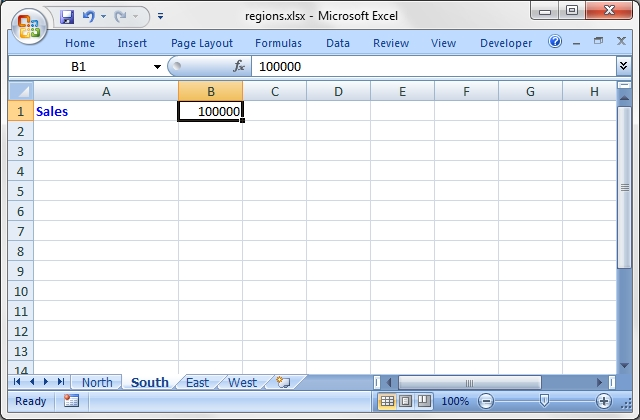Source code for this example:

``````    #!/usr/bin/perl -w

###############################################################################
#
# An example of how to use the Excel::Writer::XLSX module to write a basic
# Excel workbook with multiple worksheets.
#
# Copyright 2000-2021, John McNamara, jmcnamara@cpan.org
#

use strict;
use Excel::Writer::XLSX;

# Create a new Excel workbook
my \$workbook = Excel::Writer::XLSX->new( 'regions.xlsx' );

# Add some worksheets
my \$north = \$workbook->add_worksheet( "North" );
my \$south = \$workbook->add_worksheet( "South" );
my \$east  = \$workbook->add_worksheet( "East" );
my \$west  = \$workbook->add_worksheet( "West" );

# Add a Format
my \$format = \$workbook->add_format();
\$format->set_bold();
\$format->set_color( 'blue' );

# Add a caption to each worksheet
foreach my \$worksheet ( \$workbook->sheets() ) {
\$worksheet->write( 0, 0, "Sales", \$format );
}

# Write some data
\$north->write( 0, 1, 200000 );
\$south->write( 0, 1, 100000 );
\$east->write( 0, 1, 150000 );
\$west->write( 0, 1, 100000 );

# Set the active worksheet
\$south->activate();

# Set the width of the first column
\$south->set_column( 0, 0, 20 );

# Set the active cell
\$south->set_selection( 0, 1 );

\$workbook->close();

__END__``````

## Example: stats.pl

A simple example of how to use functions with the Excel::Writer::XLSX module.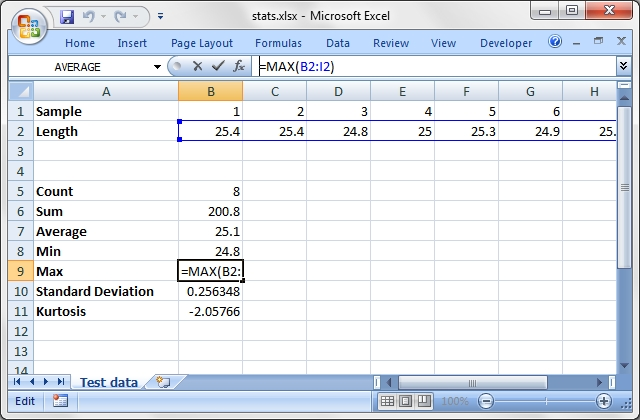Source code for this example:

``````    #!/usr/bin/perl -w

###############################################################################
#
# A simple example of how to use functions with the Excel::Writer::XLSX
# module.
#
# Copyright 2000-2021, John McNamara, jmcnamara@cpan.org
#

use strict;
use Excel::Writer::XLSX;

# Create a new workbook and add a worksheet
my \$workbook  = Excel::Writer::XLSX->new( 'stats.xlsx' );
my \$worksheet = \$workbook->add_worksheet( 'Test data' );

# Set the column width for columns 1
\$worksheet->set_column( 0, 0, 20 );

# Create a format for the headings
my \$format = \$workbook->add_format();
\$format->set_bold();

# Write the sample data
\$worksheet->write( 0, 0, 'Sample', \$format );
\$worksheet->write( 0, 1, 1 );
\$worksheet->write( 0, 2, 2 );
\$worksheet->write( 0, 3, 3 );
\$worksheet->write( 0, 4, 4 );
\$worksheet->write( 0, 5, 5 );
\$worksheet->write( 0, 6, 6 );
\$worksheet->write( 0, 7, 7 );
\$worksheet->write( 0, 8, 8 );

\$worksheet->write( 1, 0, 'Length', \$format );
\$worksheet->write( 1, 1, 25.4 );
\$worksheet->write( 1, 2, 25.4 );
\$worksheet->write( 1, 3, 24.8 );
\$worksheet->write( 1, 4, 25.0 );
\$worksheet->write( 1, 5, 25.3 );
\$worksheet->write( 1, 6, 24.9 );
\$worksheet->write( 1, 7, 25.2 );
\$worksheet->write( 1, 8, 24.8 );

# Write some statistical functions
\$worksheet->write( 4, 0, 'Count', \$format );
\$worksheet->write( 4, 1, '=COUNT(B1:I1)' );

\$worksheet->write( 5, 0, 'Sum', \$format );
\$worksheet->write( 5, 1, '=SUM(B2:I2)' );

\$worksheet->write( 6, 0, 'Average', \$format );
\$worksheet->write( 6, 1, '=AVERAGE(B2:I2)' );

\$worksheet->write( 7, 0, 'Min', \$format );
\$worksheet->write( 7, 1, '=MIN(B2:I2)' );

\$worksheet->write( 8, 0, 'Max', \$format );
\$worksheet->write( 8, 1, '=MAX(B2:I2)' );

\$worksheet->write( 9, 0, 'Standard Deviation', \$format );
\$worksheet->write( 9, 1, '=STDEV(B2:I2)' );

\$worksheet->write( 10, 0, 'Kurtosis', \$format );
\$worksheet->write( 10, 1, '=KURT(B2:I2)' );

\$workbook->close();

__END__``````

## Example: autofilter.pl

An example of how to create autofilters with Excel::Writer::XLSX.

An autofilter is a way of adding drop down lists to the headers of a 2D range of worksheet data. This allows users to filter the data based on simple criteria so that some data is shown and some is hidden.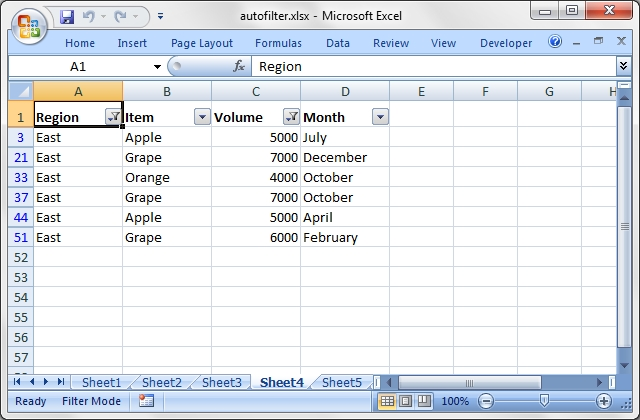Source code for this example:

``````    #!/usr/bin/perl

###############################################################################
#
# An example of how to create autofilters with Excel::Writer::XLSX.
#
# An autofilter is a way of adding drop down lists to the headers of a 2D range
# of worksheet data. This allows users to filter the data based on
# simple criteria so that some data is shown and some is hidden.
#
# Copyright 2000-2021, John McNamara, jmcnamara@cpan.org
#

use strict;
use warnings;
use Excel::Writer::XLSX;

my \$workbook = Excel::Writer::XLSX->new( 'autofilter.xlsx' );

my \$worksheet1 = \$workbook->add_worksheet();
my \$worksheet2 = \$workbook->add_worksheet();
my \$worksheet3 = \$workbook->add_worksheet();
my \$worksheet4 = \$workbook->add_worksheet();
my \$worksheet5 = \$workbook->add_worksheet();
my \$worksheet6 = \$workbook->add_worksheet();

my \$bold = \$workbook->add_format( bold => 1 );

# Extract the data embedded at the end of this file.
my @headings = split ' ', <DATA>;
my @data;
push @data, [split] while <DATA>;

# Set up several sheets with the same data.
for my \$worksheet ( \$workbook->sheets() ) {
\$worksheet->set_column( 'A:D', 12 );
\$worksheet->set_row( 0, 20, \$bold );
\$worksheet->write( 'A1', \@headings );
}

###############################################################################
#
# Example 1. Autofilter without conditions.
#

\$worksheet1->autofilter( 'A1:D51' );
\$worksheet1->write( 'A2', [ [@data] ] );

###############################################################################
#
#
# Example 2. Autofilter with a filter condition in the first column.
#

# The range in this example is the same as above but in row-column notation.
\$worksheet2->autofilter( 0, 0, 50, 3 );

# The placeholder "Region" in the filter is ignored and can be any string
# that adds clarity to the expression.
#
\$worksheet2->filter_column( 0, 'Region eq East' );

#
# Hide the rows that don't match the filter criteria.
#
my \$row = 1;

for my \$row_data ( @data ) {
my \$region = \$row_data->;

if ( \$region eq 'East' ) {

# Row is visible.
}
else {

# Hide row.
\$worksheet2->set_row( \$row, undef, undef, 1 );
}

\$worksheet2->write( \$row++, 0, \$row_data );
}

###############################################################################
#
#
# Example 3. Autofilter with a dual filter condition in one of the columns.
#

\$worksheet3->autofilter( 'A1:D51' );

\$worksheet3->filter_column( 'A', 'x eq East or x eq South' );

#
# Hide the rows that don't match the filter criteria.
#
\$row = 1;

for my \$row_data ( @data ) {
my \$region = \$row_data->;

if ( \$region eq 'East' or \$region eq 'South' ) {

# Row is visible.
}
else {

# Hide row.
\$worksheet3->set_row( \$row, undef, undef, 1 );
}

\$worksheet3->write( \$row++, 0, \$row_data );
}

###############################################################################
#
#
# Example 4. Autofilter with filter conditions in two columns.
#

\$worksheet4->autofilter( 'A1:D51' );

\$worksheet4->filter_column( 'A', 'x eq East' );
\$worksheet4->filter_column( 'C', 'x > 3000 and x < 8000' );

#
# Hide the rows that don't match the filter criteria.
#
\$row = 1;

for my \$row_data ( @data ) {
my \$region = \$row_data->;
my \$volume = \$row_data->;

if (    \$region eq 'East'
and \$volume > 3000
and \$volume < 8000 )
{

# Row is visible.
}
else {

# Hide row.
\$worksheet4->set_row( \$row, undef, undef, 1 );
}

\$worksheet4->write( \$row++, 0, \$row_data );
}

###############################################################################
#
#
# Example 5. Autofilter with filter for blanks.
#

# Create a blank cell in our test data.
\$data-> = '';

\$worksheet5->autofilter( 'A1:D51' );
\$worksheet5->filter_column( 'A', 'x == Blanks' );

#
# Hide the rows that don't match the filter criteria.
#
\$row = 1;

for my \$row_data ( @data ) {
my \$region = \$row_data->;

if ( \$region eq '' ) {

# Row is visible.
}
else {

# Hide row.
\$worksheet5->set_row( \$row, undef, undef, 1 );
}

\$worksheet5->write( \$row++, 0, \$row_data );
}

###############################################################################
#
#
# Example 6. Autofilter with filter for non-blanks.
#

\$worksheet6->autofilter( 'A1:D51' );
\$worksheet6->filter_column( 'A', 'x == NonBlanks' );

#
# Hide the rows that don't match the filter criteria.
#
\$row = 1;

for my \$row_data ( @data ) {
my \$region = \$row_data->;

if ( \$region ne '' ) {

# Row is visible.
}
else {

# Hide row.
\$worksheet6->set_row( \$row, undef, undef, 1 );
}

\$worksheet6->write( \$row++, 0, \$row_data );
}

\$workbook->close();

__DATA__
Region    Item      Volume    Month
East      Apple     9000      July
East      Apple     5000      July
South     Orange    9000      September
North     Apple     2000      November
West      Apple     9000      November
South     Pear      7000      October
North     Pear      9000      August
West      Orange    1000      December
West      Grape     1000      November
South     Pear      10000     April
West      Grape     6000      January
South     Orange    3000      May
North     Apple     3000      December
South     Apple     7000      February
West      Grape     1000      December
East      Grape     8000      February
South     Grape     10000     June
West      Pear      7000      December
South     Apple     2000      October
East      Grape     7000      December
North     Grape     6000      April
East      Pear      8000      February
North     Apple     7000      August
North     Orange    7000      July
North     Apple     6000      June
South     Grape     8000      September
West      Apple     3000      October
South     Orange    10000     November
West      Grape     4000      July
North     Orange    5000      August
East      Orange    1000      November
East      Orange    4000      October
North     Grape     5000      August
East      Apple     1000      December
South     Apple     10000     March
East      Grape     7000      October
West      Grape     1000      September
East      Grape     10000     October
South     Orange    8000      March
North     Apple     4000      July
South     Orange    5000      July
West      Apple     4000      June
East      Apple     5000      April
North     Pear      3000      August
East      Grape     9000      November
North     Orange    8000      October
East      Apple     10000     June
South     Pear      1000      December
North     Grape     10000     July
East      Grape     6000      February``````

## Example: array_formula.pl

Example of how to use the Excel::Writer::XLSX module to write simple array formulas.Source code for this example:

``````    #!/usr/bin/perl

#######################################################################
#
# Example of how to use the Excel::Writer::XLSX module to write simple
# array formulas.
#
# Copyright 2000-2021, John McNamara, jmcnamara@cpan.org
#

use strict;
use warnings;
use Excel::Writer::XLSX;

# Create a new workbook and add a worksheet
my \$workbook  = Excel::Writer::XLSX->new( 'array_formula.xlsx' );
my \$worksheet = \$workbook->add_worksheet();

# Write some test data.
\$worksheet->write( 'B1', [ [ 500, 10 ], [ 300, 15 ] ] );
\$worksheet->write( 'B5', [ [ 1, 2, 3 ], [ 20234, 21003, 10000 ] ] );

# Write an array formula that returns a single value
\$worksheet->write( 'A1', '{=SUM(B1:C1*B2:C2)}' );

# Same as above but more verbose.
\$worksheet->write_array_formula( 'A2:A2', '{=SUM(B1:C1*B2:C2)}' );

# Write an array formula that returns a range of values
\$worksheet->write_array_formula( 'A5:A7', '{=TREND(C5:C7,B5:B7)}' );

\$workbook->close();

__END__

``````

## Example: cgi.pl

Example of how to use the Excel::Writer::XLSX module to send an Excel file to a browser in a CGI program.

On Windows the hash-bang line should be something like:

``    #!C:\Perl\bin\perl.exe``

The "Content-Disposition" line will cause a prompt to be generated to save the file. If you want to stream the file to the browser instead, comment out that line as shown below.

``````    #!/usr/bin/perl

###############################################################################
#
# Example of how to use the Excel::Writer::XLSX module to send an Excel
# file to a browser in a CGI program.
#
# On Windows the hash-bang line should be something like:
#
#     #!C:\Perl\bin\perl.exe
#
# The "Content-Disposition" line will cause a prompt to be generated to save
# the file. If you want to stream the file to the browser instead, comment out
# that line as shown below.
#
# Copyright 2000-2021, John McNamara, jmcnamara@cpan.org
#

use strict;
use warnings;
use Excel::Writer::XLSX;

# Set the filename and send the content type
my \$filename = "cgitest.xlsx";

# The Content-Disposition will generate a prompt to save the file. If you want
# to stream the file to the browser, comment out the following line.
print "Content-Disposition: attachment; filename=\$filename\n";
print "\n";

# Redirect the output to STDOUT. Binmode the filehandle in case it is needed.
binmode STDOUT;

my \$workbook  = Excel::Writer::XLSX->new( \*STDOUT );
my \$worksheet = \$workbook->add_worksheet();

# Set the column width for column 1
\$worksheet->set_column( 0, 0, 20 );

# Create a format
my \$format = \$workbook->add_format();
\$format->set_bold();
\$format->set_size( 15 );
\$format->set_color( 'blue' );

# Write to the workbook
\$worksheet->write( 0, 0, "Hi Excel!", \$format );

\$workbook->close();

__END__``````

## Example: chart_area.pl

A demo of an Area chart in Excel::Writer::XLSX.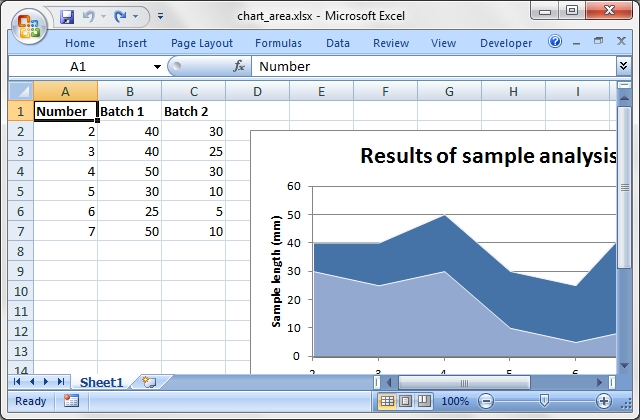Source code for this example:

``````    #!/usr/bin/perl

#######################################################################
#
# A demo of an Area chart in Excel::Writer::XLSX.
#
# Copyright 2000-2021, John McNamara, jmcnamara@cpan.org
#

use strict;
use warnings;
use Excel::Writer::XLSX;

my \$workbook  = Excel::Writer::XLSX->new( 'chart_area.xlsx' );
my \$worksheet = \$workbook->add_worksheet();
my \$bold      = \$workbook->add_format( bold => 1 );

# Add the worksheet data that the charts will refer to.
my \$headings = [ 'Number', 'Batch 1', 'Batch 2' ];
my \$data = [
[ 2,  3,  4,  5,  6,  7 ],
[ 40, 40, 50, 30, 25, 50 ],
[ 30, 25, 30, 10, 5,  10 ],

];

\$worksheet->write( 'A1', \$headings, \$bold );
\$worksheet->write( 'A2', \$data );

# Create a new chart object. In this case an embedded chart.
my \$chart1 = \$workbook->add_chart( type => 'area', embedded => 1 );

# Configure the first series.
name       => '=Sheet1!\$B\$1',
categories => '=Sheet1!\$A\$2:\$A\$7',
values     => '=Sheet1!\$B\$2:\$B\$7',
);

# Configure second series. Note alternative use of array ref to define
# ranges: [ \$sheetname, \$row_start, \$row_end, \$col_start, \$col_end ].
name       => '=Sheet1!\$C\$1',
categories => [ 'Sheet1', 1, 6, 0, 0 ],
values     => [ 'Sheet1', 1, 6, 2, 2 ],
);

# Add a chart title and some axis labels.
\$chart1->set_title ( name => 'Results of sample analysis' );
\$chart1->set_x_axis( name => 'Test number' );
\$chart1->set_y_axis( name => 'Sample length (mm)' );

# Set an Excel chart style. Blue colors with white outline and shadow.
\$chart1->set_style( 11 );

# Insert the chart into the worksheet (with an offset).
\$worksheet->insert_chart( 'D2', \$chart1, { x_offset => 25, y_offset => 10 } );

#
# Create a stacked chart sub-type
#
my \$chart2 = \$workbook->add_chart(
type     => 'area',
embedded => 1,
subtype  => 'stacked'
);

# Configure the first series.
name       => '=Sheet1!\$B\$1',
categories => '=Sheet1!\$A\$2:\$A\$7',
values     => '=Sheet1!\$B\$2:\$B\$7',
);

# Configure second series.
name       => '=Sheet1!\$C\$1',
categories => [ 'Sheet1', 1, 6, 0, 0 ],
values     => [ 'Sheet1', 1, 6, 2, 2 ],
);

# Add a chart title and some axis labels.
\$chart2->set_title ( name => 'Stacked Chart' );
\$chart2->set_x_axis( name => 'Test number' );
\$chart2->set_y_axis( name => 'Sample length (mm)' );

# Set an Excel chart style. Blue colors with white outline and shadow.
\$chart2->set_style( 12 );

# Insert the chart into the worksheet (with an offset).
\$worksheet->insert_chart( 'D18', \$chart2, { x_offset => 25, y_offset => 10 } );

#
# Create a percent stacked chart sub-type
#
my \$chart3 = \$workbook->add_chart(
type     => 'area',
embedded => 1,
subtype  => 'percent_stacked'
);

# Configure the first series.
name       => '=Sheet1!\$B\$1',
categories => '=Sheet1!\$A\$2:\$A\$7',
values     => '=Sheet1!\$B\$2:\$B\$7',
);

# Configure second series.
name       => '=Sheet1!\$C\$1',
categories => [ 'Sheet1', 1, 6, 0, 0 ],
values     => [ 'Sheet1', 1, 6, 2, 2 ],
);

# Add a chart title and some axis labels.
\$chart3->set_title ( name => 'Percent Stacked Chart' );
\$chart3->set_x_axis( name => 'Test number' );
\$chart3->set_y_axis( name => 'Sample length (mm)' );

# Set an Excel chart style. Blue colors with white outline and shadow.
\$chart3->set_style( 13 );

# Insert the chart into the worksheet (with an offset).
\$worksheet->insert_chart( 'D34', \$chart3, { x_offset => 25, y_offset => 10 } );

\$workbook->close();

__END__``````

## Example: chart_bar.pl

A demo of an Bar chart in Excel::Writer::XLSX.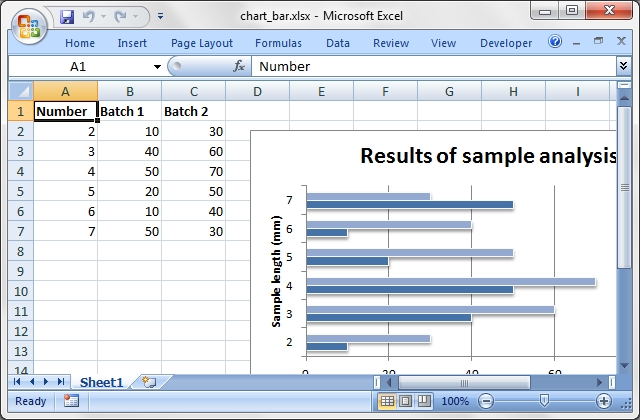Source code for this example:

``````    #!/usr/bin/perl

#######################################################################
#
# A demo of an Bar chart in Excel::Writer::XLSX.
#
# Copyright 2000-2021, John McNamara, jmcnamara@cpan.org
#

use strict;
use warnings;
use Excel::Writer::XLSX;

my \$workbook  = Excel::Writer::XLSX->new( 'chart_bar.xlsx' );
my \$worksheet = \$workbook->add_worksheet();
my \$bold      = \$workbook->add_format( bold => 1 );

# Add the worksheet data that the charts will refer to.
my \$headings = [ 'Number', 'Batch 1', 'Batch 2' ];
my \$data = [
[ 2,  3,  4,  5,  6,  7 ],
[ 10, 40, 50, 20, 10, 50 ],
[ 30, 60, 70, 50, 40, 30 ],

];

\$worksheet->write( 'A1', \$headings, \$bold );
\$worksheet->write( 'A2', \$data );

# Create a new chart object. In this case an embedded chart.
my \$chart1 = \$workbook->add_chart( type => 'bar', embedded => 1 );

# Configure the first series.
name       => '=Sheet1!\$B\$1',
categories => '=Sheet1!\$A\$2:\$A\$7',
values     => '=Sheet1!\$B\$2:\$B\$7',
);

# Configure second series. Note alternative use of array ref to define
# ranges: [ \$sheetname, \$row_start, \$row_end, \$col_start, \$col_end ].
name       => '=Sheet1!\$C\$1',
categories => [ 'Sheet1', 1, 6, 0, 0 ],
values     => [ 'Sheet1', 1, 6, 2, 2 ],
);

# Add a chart title and some axis labels.
\$chart1->set_title ( name => 'Results of sample analysis' );
\$chart1->set_x_axis( name => 'Test number' );
\$chart1->set_y_axis( name => 'Sample length (mm)' );

# Set an Excel chart style. Blue colors with white outline and shadow.
\$chart1->set_style( 11 );

# Insert the chart into the worksheet (with an offset).
\$worksheet->insert_chart( 'D2', \$chart1, { x_offset => 25, y_offset => 10 } );

#
# Create a stacked chart sub-type
#
my \$chart2 = \$workbook->add_chart(
type     => 'bar',
embedded => 1,
subtype  => 'stacked'
);

# Configure the first series.
name       => '=Sheet1!\$B\$1',
categories => '=Sheet1!\$A\$2:\$A\$7',
values     => '=Sheet1!\$B\$2:\$B\$7',
);

# Configure second series.
name       => '=Sheet1!\$C\$1',
categories => [ 'Sheet1', 1, 6, 0, 0 ],
values     => [ 'Sheet1', 1, 6, 2, 2 ],
);

# Add a chart title and some axis labels.
\$chart2->set_title ( name => 'Stacked Chart' );
\$chart2->set_x_axis( name => 'Test number' );
\$chart2->set_y_axis( name => 'Sample length (mm)' );

# Set an Excel chart style. Blue colors with white outline and shadow.
\$chart2->set_style( 12 );

# Insert the chart into the worksheet (with an offset).
\$worksheet->insert_chart( 'D18', \$chart2, { x_offset => 25, y_offset => 10 } );

#
# Create a percent stacked chart sub-type
#
my \$chart3 = \$workbook->add_chart(
type     => 'bar',
embedded => 1,
subtype  => 'percent_stacked'
);

# Configure the first series.
name       => '=Sheet1!\$B\$1',
categories => '=Sheet1!\$A\$2:\$A\$7',
values     => '=Sheet1!\$B\$2:\$B\$7',
);

# Configure second series.
name       => '=Sheet1!\$C\$1',
categories => [ 'Sheet1', 1, 6, 0, 0 ],
values     => [ 'Sheet1', 1, 6, 2, 2 ],
);

# Add a chart title and some axis labels.
\$chart3->set_title ( name => 'Percent Stacked Chart' );
\$chart3->set_x_axis( name => 'Test number' );
\$chart3->set_y_axis( name => 'Sample length (mm)' );

# Set an Excel chart style. Blue colors with white outline and shadow.
\$chart3->set_style( 13 );

# Insert the chart into the worksheet (with an offset).
\$worksheet->insert_chart( 'D34', \$chart3, { x_offset => 25, y_offset => 10 } );

\$workbook->close();

__END__``````

## Example: chart_column.pl

A demo of a Column chart in Excel::Writer::XLSX.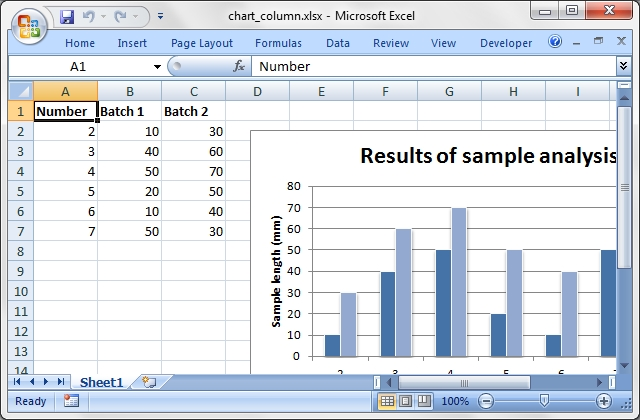Source code for this example:

``````    #!/usr/bin/perl

#######################################################################
#
# A demo of a Column chart in Excel::Writer::XLSX.
#
# Copyright 2000-2021, John McNamara, jmcnamara@cpan.org
#

use strict;
use warnings;
use Excel::Writer::XLSX;

my \$workbook  = Excel::Writer::XLSX->new( 'chart_column.xlsx' );
my \$worksheet = \$workbook->add_worksheet();
my \$bold      = \$workbook->add_format( bold => 1 );

# Add the worksheet data that the charts will refer to.
my \$headings = [ 'Number', 'Batch 1', 'Batch 2' ];
my \$data = [
[ 2,  3,  4,  5,  6,  7 ],
[ 10, 40, 50, 20, 10, 50 ],
[ 30, 60, 70, 50, 40, 30 ],

];

\$worksheet->write( 'A1', \$headings, \$bold );
\$worksheet->write( 'A2', \$data );

# Create a new chart object. In this case an embedded chart.
my \$chart1 = \$workbook->add_chart( type => 'column', embedded => 1 );

# Configure the first series.
name       => '=Sheet1!\$B\$1',
categories => '=Sheet1!\$A\$2:\$A\$7',
values     => '=Sheet1!\$B\$2:\$B\$7',
);

# Configure second series. Note alternative use of array ref to define
# ranges: [ \$sheetname, \$row_start, \$row_end, \$col_start, \$col_end ].
name       => '=Sheet1!\$C\$1',
categories => [ 'Sheet1', 1, 6, 0, 0 ],
values     => [ 'Sheet1', 1, 6, 2, 2 ],
);

# Add a chart title and some axis labels.
\$chart1->set_title ( name => 'Results of sample analysis' );
\$chart1->set_x_axis( name => 'Test number' );
\$chart1->set_y_axis( name => 'Sample length (mm)' );

# Set an Excel chart style. Blue colors with white outline and shadow.
\$chart1->set_style( 11 );

# Insert the chart into the worksheet (with an offset).
\$worksheet->insert_chart( 'D2', \$chart1, { x_offset => 25, y_offset => 10 } );

#
# Create a stacked chart sub-type
#
my \$chart2 = \$workbook->add_chart(
type     => 'column',
embedded => 1,
subtype  => 'stacked'
);

# Configure the first series.
name       => '=Sheet1!\$B\$1',
categories => '=Sheet1!\$A\$2:\$A\$7',
values     => '=Sheet1!\$B\$2:\$B\$7',
);

# Configure second series.
name       => '=Sheet1!\$C\$1',
categories => [ 'Sheet1', 1, 6, 0, 0 ],
values     => [ 'Sheet1', 1, 6, 2, 2 ],
);

# Add a chart title and some axis labels.
\$chart2->set_title ( name => 'Stacked Chart' );
\$chart2->set_x_axis( name => 'Test number' );
\$chart2->set_y_axis( name => 'Sample length (mm)' );

# Set an Excel chart style. Blue colors with white outline and shadow.
\$chart2->set_style( 12 );

# Insert the chart into the worksheet (with an offset).
\$worksheet->insert_chart( 'D18', \$chart2, { x_offset => 25, y_offset => 10 } );

#
# Create a percent stacked chart sub-type
#
my \$chart3 = \$workbook->add_chart(
type     => 'column',
embedded => 1,
subtype  => 'percent_stacked'
);

# Configure the first series.
name       => '=Sheet1!\$B\$1',
categories => '=Sheet1!\$A\$2:\$A\$7',
values     => '=Sheet1!\$B\$2:\$B\$7',
);

# Configure second series.
name       => '=Sheet1!\$C\$1',
categories => [ 'Sheet1', 1, 6, 0, 0 ],
values     => [ 'Sheet1', 1, 6, 2, 2 ],
);

# Add a chart title and some axis labels.
\$chart3->set_title ( name => 'Percent Stacked Chart' );
\$chart3->set_x_axis( name => 'Test number' );
\$chart3->set_y_axis( name => 'Sample length (mm)' );

# Set an Excel chart style. Blue colors with white outline and shadow.
\$chart3->set_style( 13 );

# Insert the chart into the worksheet (with an offset).
\$worksheet->insert_chart( 'D34', \$chart3, { x_offset => 25, y_offset => 10 } );

\$workbook->close();

__END__``````

## Example: chart_line.pl

A demo of a Line chart in Excel::Writer::XLSX.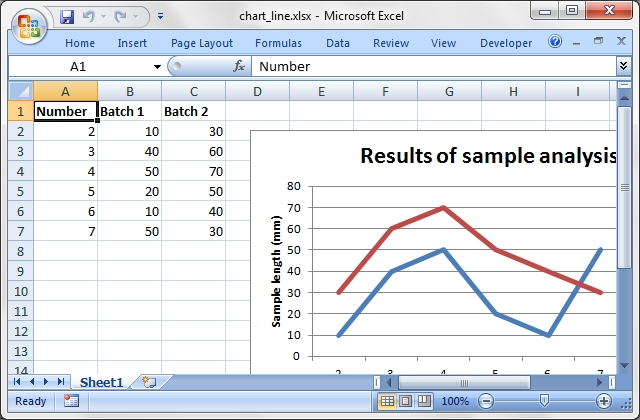Source code for this example:

``````    #!/usr/bin/perl

#######################################################################
#
# A demo of a Line chart in Excel::Writer::XLSX.
#
# Copyright 2000-2021, John McNamara, jmcnamara@cpan.org
#

use strict;
use warnings;
use Excel::Writer::XLSX;

my \$workbook  = Excel::Writer::XLSX->new( 'chart_line.xlsx' );
my \$worksheet = \$workbook->add_worksheet();
my \$bold      = \$workbook->add_format( bold => 1 );

# Add the worksheet data that the charts will refer to.
my \$headings = [ 'Number', 'Batch 1', 'Batch 2' ];
my \$data = [
[ 2, 3, 4, 5, 6, 7 ],
[ 10, 40, 50, 20, 10, 50 ],
[ 30, 60, 70, 50, 40, 30 ],

];

\$worksheet->write( 'A1', \$headings, \$bold );
\$worksheet->write( 'A2', \$data );

# Create a new chart object. In this case an embedded chart.
my \$chart = \$workbook->add_chart( type => 'line', embedded => 1 );

# Configure the first series.
name       => '=Sheet1!\$B\$1',
categories => '=Sheet1!\$A\$2:\$A\$7',
values     => '=Sheet1!\$B\$2:\$B\$7',
);

# Configure second series. Note alternative use of array ref to define
# ranges: [ \$sheetname, \$row_start, \$row_end, \$col_start, \$col_end ].
name       => '=Sheet1!\$C\$1',
categories => [ 'Sheet1', 1, 6, 0, 0 ],
values     => [ 'Sheet1', 1, 6, 2, 2 ],
);

# Add a chart title and some axis labels.
\$chart->set_title ( name => 'Results of sample analysis' );
\$chart->set_x_axis( name => 'Test number' );
\$chart->set_y_axis( name => 'Sample length (mm)' );

# Set an Excel chart style. Colors with white outline and shadow.
\$chart->set_style( 10 );

# Insert the chart into the worksheet (with an offset).
\$worksheet->insert_chart( 'D2', \$chart, { x_offset => 25, y_offset => 10 } );

#
# Create a stacked chart sub-type
#
my \$chart2 = \$workbook->add_chart(
type     => 'line',
embedded => 1,
subtype  => 'stacked'
);

# Configure the first series.
name       => '=Sheet1!\$B\$1',
categories => '=Sheet1!\$A\$2:\$A\$7',
values     => '=Sheet1!\$B\$2:\$B\$7',
);

# Configure second series.
name       => '=Sheet1!\$C\$1',
categories => [ 'Sheet1', 1, 6, 0, 0 ],
values     => [ 'Sheet1', 1, 6, 2, 2 ],
);

# Add a chart title and some axis labels.
\$chart2->set_title ( name => 'Stacked Chart' );
\$chart2->set_x_axis( name => 'Test number' );
\$chart2->set_y_axis( name => 'Sample length (mm)' );

# Set an Excel chart style. Blue colors with white outline and shadow.
\$chart2->set_style( 12 );

# Insert the chart into the worksheet (with an offset).
\$worksheet->insert_chart( 'D18', \$chart2, { x_offset => 25, y_offset => 10 } );

#
# Create a percent stacked chart sub-type
#
my \$chart3 = \$workbook->add_chart(
type     => 'line',
embedded => 1,
subtype  => 'percent_stacked'
);

# Configure the first series.
name       => '=Sheet1!\$B\$1',
categories => '=Sheet1!\$A\$2:\$A\$7',
values     => '=Sheet1!\$B\$2:\$B\$7',
);

# Configure second series.
name       => '=Sheet1!\$C\$1',
categories => [ 'Sheet1', 1, 6, 0, 0 ],
values     => [ 'Sheet1', 1, 6, 2, 2 ],
);

# Add a chart title and some axis labels.
\$chart3->set_title ( name => 'Percent Stacked Chart' );
\$chart3->set_x_axis( name => 'Test number' );
\$chart3->set_y_axis( name => 'Sample length (mm)' );

# Set an Excel chart style. Blue colors with white outline and shadow.
\$chart3->set_style( 13 );

# Insert the chart into the worksheet (with an offset).
\$worksheet->insert_chart( 'D34', \$chart3, { x_offset => 25, y_offset => 10 } );

\$workbook->close();

__END__``````

## Example: chart_pie.pl

A demo of a Pie chart in Excel::Writer::XLSX.

The demo also shows how to set segment colours. It is possible to define chart colors for most types of Excel::Writer::XLSX charts via the add_series() method. However, Pie and Doughtnut charts are a special case since each segment is represented as a point so it is necessary to assign formatting to each point in the series.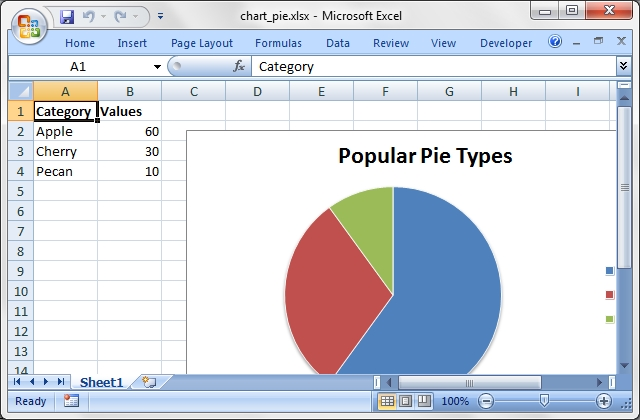Source code for this example:

``````    #!/usr/bin/perl

#######################################################################
#
# A demo of a Pie chart in Excel::Writer::XLSX.
#
# The demo also shows how to set segment colours. It is possible to define
# chart colors for most types of Excel::Writer::XLSX charts via the
# add_series() method. However, Pie and Doughtnut charts are a special case
# since each segment is represented as a point so it is necessary to assign
# formatting to each point in the series.
#
# Copyright 2000-2021, John McNamara, jmcnamara@cpan.org
#

use strict;
use warnings;
use Excel::Writer::XLSX;

my \$workbook  = Excel::Writer::XLSX->new( 'chart_pie.xlsx' );
my \$worksheet = \$workbook->add_worksheet();
my \$bold      = \$workbook->add_format( bold => 1 );

# Add the worksheet data that the charts will refer to.
my \$headings = [ 'Category', 'Values' ];
my \$data = [
[ 'Apple', 'Cherry', 'Pecan' ],
[ 60,       30,       10     ],
];

\$worksheet->write( 'A1', \$headings, \$bold );
\$worksheet->write( 'A2', \$data );

# Create a new chart object. In this case an embedded chart.
my \$chart1 = \$workbook->add_chart( type => 'pie', embedded => 1 );

# Configure the series. Note the use of the array ref to define ranges:
# [ \$sheetname, \$row_start, \$row_end, \$col_start, \$col_end ].
# See below for an alternative syntax.
name       => 'Pie sales data',
categories => [ 'Sheet1', 1, 3, 0, 0 ],
values     => [ 'Sheet1', 1, 3, 1, 1 ],
);

# Add a title.
\$chart1->set_title( name => 'Popular Pie Types' );

# Set an Excel chart style. Colors with white outline and shadow.
\$chart1->set_style( 10 );

# Insert the chart into the worksheet (with an offset).
\$worksheet->insert_chart( 'C2', \$chart1, { x_offset => 25, y_offset => 10 } );

#
# Create a Pie chart with user defined segment colors.
#

# Create an example Pie chart like above.
my \$chart2 = \$workbook->add_chart( type => 'pie', embedded => 1 );

# Configure the series and add user defined segment colours.
name       => 'Pie sales data',
categories => '=Sheet1!\$A\$2:\$A\$4',
values     => '=Sheet1!\$B\$2:\$B\$4',
points     => [
{ fill => { color => '#5ABA10' } },
{ fill => { color => '#FE110E' } },
{ fill => { color => '#CA5C05' } },
],
);

# Add a title.
\$chart2->set_title( name => 'Pie Chart with user defined colors' );

# Insert the chart into the worksheet (with an offset).
\$worksheet->insert_chart( 'C18', \$chart2, { x_offset => 25, y_offset => 10 } );

#
# Create a Pie chart with rotation of the segments.
#

# Create an example Pie chart like above.
my \$chart3 = \$workbook->add_chart( type => 'pie', embedded => 1 );

# Configure the series.
name       => 'Pie sales data',
categories => '=Sheet1!\$A\$2:\$A\$4',
values     => '=Sheet1!\$B\$2:\$B\$4',
);

# Add a title.
\$chart3->set_title( name => 'Pie Chart with segment rotation' );

# Change the angle/rotation of the first segment.
\$chart3->set_rotation(90);

# Insert the chart into the worksheet (with an offset).
\$worksheet->insert_chart( 'C34', \$chart3, { x_offset => 25, y_offset => 10 } );

\$workbook->close();

__END__``````

## Example: chart_doughnut.pl

A demo of a Doughnut chart in Excel::Writer::XLSX.

The demo also shows how to set segment colours. It is possible to define chart colors for most types of Excel::Writer::XLSX charts via the add_series() method. However, Pie and Doughtnut charts are a special case since each segment is represented as a point so it is necessary to assign formatting to each point in the series.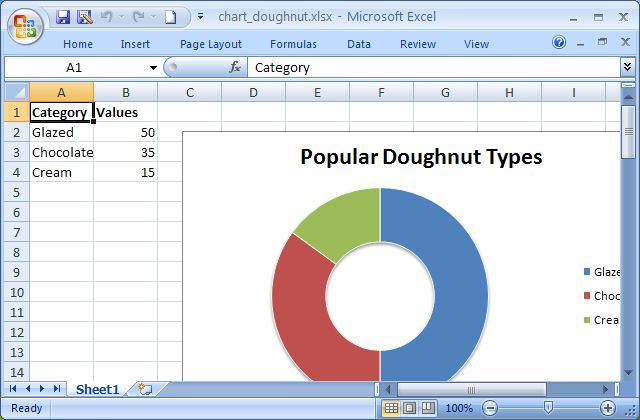Source code for this example:

``````    #!/usr/bin/perl

#######################################################################
#
# A demo of a Doughnut chart in Excel::Writer::XLSX.
#
# The demo also shows how to set segment colours. It is possible to define
# chart colors for most types of Excel::Writer::XLSX charts via the
# add_series() method. However, Pie and Doughtnut charts are a special case
# since each segment is represented as a point so it is necessary to assign
# formatting to each point in the series.
#
# Copyright 2000-2021, John McNamara, jmcnamara@cpan.org
#

use strict;
use warnings;
use Excel::Writer::XLSX;

my \$workbook  = Excel::Writer::XLSX->new( 'chart_doughnut.xlsx' );
my \$worksheet = \$workbook->add_worksheet();
my \$bold      = \$workbook->add_format( bold => 1 );

# Add the worksheet data that the charts will refer to.
my \$headings = [ 'Category', 'Values' ];
my \$data = [
[ 'Glazed', 'Chocolate', 'Cream' ],
[ 50,       35,          15      ],
];

\$worksheet->write( 'A1', \$headings, \$bold );
\$worksheet->write( 'A2', \$data );

# Create a new chart object. In this case an embedded chart.
my \$chart1 = \$workbook->add_chart( type => 'doughnut', embedded => 1 );

# Configure the series. Note the use of the array ref to define ranges:
# [ \$sheetname, \$row_start, \$row_end, \$col_start, \$col_end ].
# See below for an alternative syntax.
name       => 'Doughnut sales data',
categories => [ 'Sheet1', 1, 3, 0, 0 ],
values     => [ 'Sheet1', 1, 3, 1, 1 ],
);

# Add a title.
\$chart1->set_title( name => 'Popular Doughnut Types' );

# Set an Excel chart style. Colors with white outline and shadow.
\$chart1->set_style( 10 );

# Insert the chart into the worksheet (with an offset).
\$worksheet->insert_chart( 'C2', \$chart1, { x_offset => 25, y_offset => 10 } );

#
# Create a Doughnut chart with user defined segment colors.
#

# Create an example Doughnut chart like above.
my \$chart2 = \$workbook->add_chart( type => 'doughnut', embedded => 1 );

# Configure the series and add user defined segment colours.
name       => 'Doughnut sales data',
categories => '=Sheet1!\$A\$2:\$A\$4',
values     => '=Sheet1!\$B\$2:\$B\$4',
points     => [
{ fill => { color => '#FA58D0' } },
{ fill => { color => '#61210B' } },
{ fill => { color => '#F5F6CE' } },
],
);

# Add a title.
\$chart2->set_title( name => 'Doughnut Chart with user defined colors' );

# Insert the chart into the worksheet (with an offset).
\$worksheet->insert_chart( 'C18', \$chart2, { x_offset => 25, y_offset => 10 } );

#
# Create a Doughnut chart with rotation of the segments.
#

# Create an example Doughnut chart like above.
my \$chart3 = \$workbook->add_chart( type => 'doughnut', embedded => 1 );

# Configure the series.
name       => 'Doughnut sales data',
categories => '=Sheet1!\$A\$2:\$A\$4',
values     => '=Sheet1!\$B\$2:\$B\$4',
);

# Add a title.
\$chart3->set_title( name => 'Doughnut Chart with segment rotation' );

# Change the angle/rotation of the first segment.
\$chart3->set_rotation(90);

# Insert the chart into the worksheet (with an offset).
\$worksheet->insert_chart( 'C34', \$chart3, { x_offset => 25, y_offset => 10 } );

#
# Create a Doughnut chart with user defined hole size.
#

# Create an example Doughnut chart like above.
my \$chart4 = \$workbook->add_chart( type => 'doughnut', embedded => 1 );

# Configure the series.
name       => 'Doughnut sales data',
categories => '=Sheet1!\$A\$2:\$A\$4',
values     => '=Sheet1!\$B\$2:\$B\$4',
);

# Add a title.
\$chart4->set_title( name => 'Doughnut Chart with user defined hole size' );

# Change the hole size.
\$chart4->set_hole_size(33);

# Insert the chart into the worksheet (with an offset).
\$worksheet->insert_chart( 'C50', \$chart4, { x_offset => 25, y_offset => 10 } );

\$workbook->close();

__END__``````

A demo of an Radar chart in Excel::Writer::XLSX.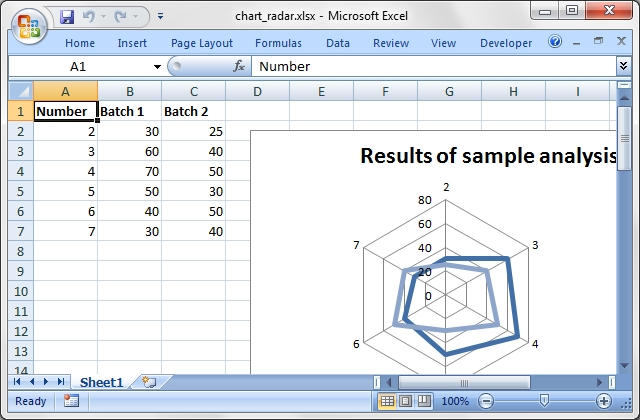Source code for this example:

``````    #!/usr/bin/perl

#######################################################################
#
# A demo of an Radar chart in Excel::Writer::XLSX.
#
# Copyright 2000-2021, John McNamara, jmcnamara@cpan.org
#

use strict;
use warnings;
use Excel::Writer::XLSX;

my \$workbook  = Excel::Writer::XLSX->new( 'chart_radar.xlsx' );
my \$worksheet = \$workbook->add_worksheet();
my \$bold      = \$workbook->add_format( bold => 1 );

# Add the worksheet data that the charts will refer to.
my \$headings = [ 'Number', 'Batch 1', 'Batch 2' ];
my \$data = [
[ 2,  3,  4,  5,  6,  7 ],
[ 30, 60, 70, 50, 40, 30 ],
[ 25, 40, 50, 30, 50, 40 ],

];

\$worksheet->write( 'A1', \$headings, \$bold );
\$worksheet->write( 'A2', \$data );

# Create a new chart object. In this case an embedded chart.
my \$chart1 = \$workbook->add_chart( type => 'radar', embedded => 1 );

# Configure the first series.
name       => '=Sheet1!\$B\$1',
categories => '=Sheet1!\$A\$2:\$A\$7',
values     => '=Sheet1!\$B\$2:\$B\$7',
);

# Configure second series. Note alternative use of array ref to define
# ranges: [ \$sheetname, \$row_start, \$row_end, \$col_start, \$col_end ].
name       => '=Sheet1!\$C\$1',
categories => [ 'Sheet1', 1, 6, 0, 0 ],
values     => [ 'Sheet1', 1, 6, 2, 2 ],
);

# Add a chart title and some axis labels.
\$chart1->set_title ( name => 'Results of sample analysis' );
\$chart1->set_x_axis( name => 'Test number' );
\$chart1->set_y_axis( name => 'Sample length (mm)' );

# Set an Excel chart style. Blue colors with white outline and shadow.
\$chart1->set_style( 11 );

# Insert the chart into the worksheet (with an offset).
\$worksheet->insert_chart( 'D2', \$chart1, { x_offset => 25, y_offset => 10 } );

#
# Create a with_markers chart sub-type
#
my \$chart2 = \$workbook->add_chart(
embedded => 1,
subtype  => 'with_markers'
);

# Configure the first series.
name       => '=Sheet1!\$B\$1',
categories => '=Sheet1!\$A\$2:\$A\$7',
values     => '=Sheet1!\$B\$2:\$B\$7',
);

# Configure second series.
name       => '=Sheet1!\$C\$1',
categories => [ 'Sheet1', 1, 6, 0, 0 ],
values     => [ 'Sheet1', 1, 6, 2, 2 ],
);

# Add a chart title and some axis labels.
\$chart2->set_title ( name => 'Stacked Chart' );
\$chart2->set_x_axis( name => 'Test number' );
\$chart2->set_y_axis( name => 'Sample length (mm)' );

# Set an Excel chart style. Blue colors with white outline and shadow.
\$chart2->set_style( 12 );

# Insert the chart into the worksheet (with an offset).
\$worksheet->insert_chart( 'D18', \$chart2, { x_offset => 25, y_offset => 10 } );

#
# Create a filled chart sub-type
#
my \$chart3 = \$workbook->add_chart(
embedded => 1,
subtype  => 'filled'
);

# Configure the first series.
name       => '=Sheet1!\$B\$1',
categories => '=Sheet1!\$A\$2:\$A\$7',
values     => '=Sheet1!\$B\$2:\$B\$7',
);

# Configure second series.
name       => '=Sheet1!\$C\$1',
categories => [ 'Sheet1', 1, 6, 0, 0 ],
values     => [ 'Sheet1', 1, 6, 2, 2 ],
);

# Add a chart title and some axis labels.
\$chart3->set_title ( name => 'Percent Stacked Chart' );
\$chart3->set_x_axis( name => 'Test number' );
\$chart3->set_y_axis( name => 'Sample length (mm)' );

# Set an Excel chart style. Blue colors with white outline and shadow.
\$chart3->set_style( 13 );

# Insert the chart into the worksheet (with an offset).
\$worksheet->insert_chart( 'D34', \$chart3, { x_offset => 25, y_offset => 10 } );

\$workbook->close();

__END__``````

## Example: chart_scatter.pl

A demo of a Scatter chart in Excel::Writer::XLSX. Other subtypes are also supported such as markers_only (the default), straight_with_markers, straight, smooth_with_markers and smooth. See the main documentation for more details.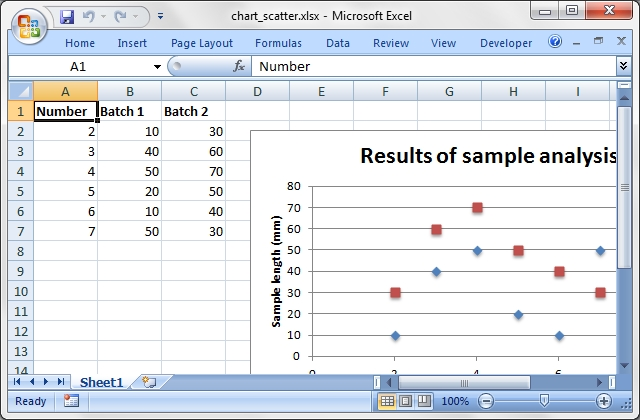Source code for this example:

``````    #!/usr/bin/perl

#######################################################################
#
# A demo of a Scatter chart in Excel::Writer::XLSX. Other subtypes are
# also supported such as markers_only (the default), straight_with_markers,
# straight, smooth_with_markers and smooth. See the main documentation for
# more details.
#
# Copyright 2000-2021, John McNamara, jmcnamara@cpan.org
#

use strict;
use warnings;
use Excel::Writer::XLSX;

my \$workbook  = Excel::Writer::XLSX->new( 'chart_scatter.xlsx' );
my \$worksheet = \$workbook->add_worksheet();
my \$bold      = \$workbook->add_format( bold => 1 );

# Add the worksheet data that the charts will refer to.
my \$headings = [ 'Number', 'Batch 1', 'Batch 2' ];
my \$data = [
[ 2,  3,  4,  5,  6,  7 ],
[ 10, 40, 50, 20, 10, 50 ],
[ 30, 60, 70, 50, 40, 30 ],

];

\$worksheet->write( 'A1', \$headings, \$bold );
\$worksheet->write( 'A2', \$data );

# Create a new chart object. In this case an embedded chart.
my \$chart1 = \$workbook->add_chart( type => 'scatter', embedded => 1 );

# Configure the first series.
name       => '=Sheet1!\$B\$1',
categories => '=Sheet1!\$A\$2:\$A\$7',
values     => '=Sheet1!\$B\$2:\$B\$7',
);

# Configure second series. Note alternative use of array ref to define
# ranges: [ \$sheetname, \$row_start, \$row_end, \$col_start, \$col_end ].
name       => '=Sheet1!\$C\$1',
categories => [ 'Sheet1', 1, 6, 0, 0 ],
values     => [ 'Sheet1', 1, 6, 2, 2 ],
);

# Add a chart title and some axis labels.
\$chart1->set_title ( name => 'Results of sample analysis' );
\$chart1->set_x_axis( name => 'Test number' );
\$chart1->set_y_axis( name => 'Sample length (mm)' );

# Set an Excel chart style. Blue colors with white outline and shadow.
\$chart1->set_style( 11 );

# Insert the chart into the worksheet (with an offset).
\$worksheet->insert_chart( 'D2', \$chart1, { x_offset => 25, y_offset => 10 } );

#
# Create a scatter chart sub-type with straight lines and markers.
#
my \$chart2 = \$workbook->add_chart(
type     => 'scatter',
embedded => 1,
subtype  => 'straight_with_markers'
);

# Configure the first series.
name       => '=Sheet1!\$B\$1',
categories => '=Sheet1!\$A\$2:\$A\$7',
values     => '=Sheet1!\$B\$2:\$B\$7',
);

# Configure second series.
name       => '=Sheet1!\$C\$1',
categories => [ 'Sheet1', 1, 6, 0, 0 ],
values     => [ 'Sheet1', 1, 6, 2, 2 ],
);

# Add a chart title and some axis labels.
\$chart2->set_title ( name => 'Straight line with markers' );
\$chart2->set_x_axis( name => 'Test number' );
\$chart2->set_y_axis( name => 'Sample length (mm)' );

# Set an Excel chart style. Blue colors with white outline and shadow.
\$chart2->set_style( 12 );

# Insert the chart into the worksheet (with an offset).
\$worksheet->insert_chart( 'D18', \$chart2, { x_offset => 25, y_offset => 10 } );

#
# Create a scatter chart sub-type with straight lines and no markers.
#
my \$chart3 = \$workbook->add_chart(
type     => 'scatter',
embedded => 1,
subtype  => 'straight'
);

# Configure the first series.
name       => '=Sheet1!\$B\$1',
categories => '=Sheet1!\$A\$2:\$A\$7',
values     => '=Sheet1!\$B\$2:\$B\$7',
);

# Configure second series.
name       => '=Sheet1!\$C\$1',
categories => [ 'Sheet1', 1, 6, 0, 0 ],
values     => [ 'Sheet1', 1, 6, 2, 2 ],
);

# Add a chart title and some axis labels.
\$chart3->set_title ( name => 'Straight line' );
\$chart3->set_x_axis( name => 'Test number' );
\$chart3->set_y_axis( name => 'Sample length (mm)' );

# Set an Excel chart style. Blue colors with white outline and shadow.
\$chart3->set_style( 13 );

# Insert the chart into the worksheet (with an offset).
\$worksheet->insert_chart( 'D34', \$chart3, { x_offset => 25, y_offset => 10 } );

#
# Create a scatter chart sub-type with smooth lines and markers.
#
my \$chart4 = \$workbook->add_chart(
type     => 'scatter',
embedded => 1,
subtype  => 'smooth_with_markers'
);

# Configure the first series.
name       => '=Sheet1!\$B\$1',
categories => '=Sheet1!\$A\$2:\$A\$7',
values     => '=Sheet1!\$B\$2:\$B\$7',
);

# Configure second series.
name       => '=Sheet1!\$C\$1',
categories => [ 'Sheet1', 1, 6, 0, 0 ],
values     => [ 'Sheet1', 1, 6, 2, 2 ],
);

# Add a chart title and some axis labels.
\$chart4->set_title ( name => 'Smooth line with markers' );
\$chart4->set_x_axis( name => 'Test number' );
\$chart4->set_y_axis( name => 'Sample length (mm)' );

# Set an Excel chart style. Blue colors with white outline and shadow.
\$chart4->set_style( 14 );

# Insert the chart into the worksheet (with an offset).
\$worksheet->insert_chart( 'D51', \$chart4, { x_offset => 25, y_offset => 10 } );

#
# Create a scatter chart sub-type with smooth lines and no markers.
#
my \$chart5 = \$workbook->add_chart(
type     => 'scatter',
embedded => 1,
subtype  => 'smooth'
);

# Configure the first series.
name       => '=Sheet1!\$B\$1',
categories => '=Sheet1!\$A\$2:\$A\$7',
values     => '=Sheet1!\$B\$2:\$B\$7',
);

# Configure second series.
name       => '=Sheet1!\$C\$1',
categories => [ 'Sheet1', 1, 6, 0, 0 ],
values     => [ 'Sheet1', 1, 6, 2, 2 ],
);

# Add a chart title and some axis labels.
\$chart5->set_title ( name => 'Smooth line' );
\$chart5->set_x_axis( name => 'Test number' );
\$chart5->set_y_axis( name => 'Sample length (mm)' );

# Set an Excel chart style. Blue colors with white outline and shadow.
\$chart5->set_style( 15 );

# Insert the chart into the worksheet (with an offset).
\$worksheet->insert_chart( 'D66', \$chart5, { x_offset => 25, y_offset => 10 } );

\$workbook->close();

__END__``````

## Example: chart_secondary_axis.pl

A demo of a Line chart with a secondary axis in Excel::Writer::XLSX.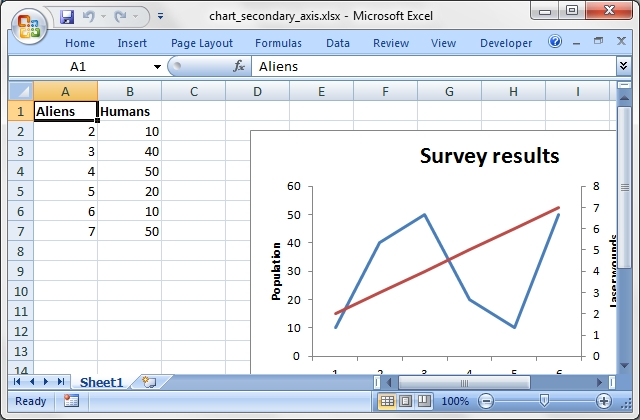Source code for this example:

``````    #!/usr/bin/perl

#######################################################################
#
# A demo of a Line chart with a secondary axis in Excel::Writer::XLSX.
#
# Copyright 2000-2021, John McNamara, jmcnamara@cpan.org
#

use strict;
use warnings;
use Excel::Writer::XLSX;

my \$workbook  = Excel::Writer::XLSX->new( 'chart_secondary_axis.xlsx' );
my \$worksheet = \$workbook->add_worksheet();
my \$bold      = \$workbook->add_format( bold => 1 );

# Add the worksheet data that the charts will refer to.
my \$headings = [ 'Aliens', 'Humans', ];
my \$data = [
[ 2,  3,  4,  5,  6,  7 ],
[ 10, 40, 50, 20, 10, 50 ],

];

\$worksheet->write( 'A1', \$headings, \$bold );
\$worksheet->write( 'A2', \$data );

# Create a new chart object. In this case an embedded chart.
my \$chart = \$workbook->add_chart( type => 'line', embedded => 1 );

# Configure a series with a secondary axis
name    => '=Sheet1!\$A\$1',
values  => '=Sheet1!\$A\$2:\$A\$7',
y2_axis => 1,
);

name   => '=Sheet1!\$B\$1',
values => '=Sheet1!\$B\$2:\$B\$7',
);

\$chart->set_legend( position => 'right' );

# Add a chart title and some axis labels.
\$chart->set_title( name => 'Survey results' );
\$chart->set_x_axis( name => 'Days', );
\$chart->set_y_axis( name => 'Population', major_gridlines => { visible => 0 } );
\$chart->set_y2_axis( name => 'Laser wounds' );

# Insert the chart into the worksheet (with an offset).
\$worksheet->insert_chart( 'D2', \$chart, { x_offset => 25, y_offset => 10 } );

\$workbook->close();

__END__``````

## Example: chart_combined.pl

An example of a Combined chart in Excel::Writer::XLSX.

``````    #!/usr/bin/perl

#######################################################################
#
# An example of a Combined chart in Excel::Writer::XLSX.
#
# Copyright 2000-2021, John McNamara, jmcnamara@cpan.org
#

use strict;
use warnings;
use Excel::Writer::XLSX;

my \$workbook  = Excel::Writer::XLSX->new( 'chart_combined.xlsx' );
my \$worksheet = \$workbook->add_worksheet();
my \$bold      = \$workbook->add_format( bold => 1 );

# Add the worksheet data that the charts will refer to.
my \$headings = [ 'Number', 'Batch 1', 'Batch 2' ];
my \$data = [
[ 2,  3,  4,  5,  6,  7 ],
[ 10, 40, 50, 20, 10, 50 ],
[ 30, 60, 70, 50, 40, 30 ],

];

\$worksheet->write( 'A1', \$headings, \$bold );
\$worksheet->write( 'A2', \$data );

#
# In the first example we will create a combined column and line chart.
# They will share the same X and Y axes.
#

# Create a new column chart. This will use this as the primary chart.
my \$column_chart1 = \$workbook->add_chart( type => 'column', embedded => 1 );

# Configure the data series for the primary chart.
name       => '=Sheet1!\$B\$1',
categories => '=Sheet1!\$A\$2:\$A\$7',
values     => '=Sheet1!\$B\$2:\$B\$7',
);

# Create a new column chart. This will use this as the secondary chart.
my \$line_chart1 = \$workbook->add_chart( type => 'line', embedded => 1 );

# Configure the data series for the secondary chart.
name       => '=Sheet1!\$C\$1',
categories => '=Sheet1!\$A\$2:\$A\$7',
values     => '=Sheet1!\$C\$2:\$C\$7',
);

# Combine the charts.
\$column_chart1->combine( \$line_chart1 );

# Add a chart title and some axis labels. Note, this is done via the
# primary chart.
\$column_chart1->set_title( name => 'Combined chart - same Y axis' );
\$column_chart1->set_x_axis( name => 'Test number' );
\$column_chart1->set_y_axis( name => 'Sample length (mm)' );

# Insert the chart into the worksheet
\$worksheet->insert_chart( 'E2', \$column_chart1 );

#
# In the second example we will create a similar combined column and line
# chart except that the secondary chart will have a secondary Y axis.
#

# Create a new column chart. This will use this as the primary chart.
my \$column_chart2 = \$workbook->add_chart( type => 'column', embedded => 1 );

# Configure the data series for the primary chart.
name       => '=Sheet1!\$B\$1',
categories => '=Sheet1!\$A\$2:\$A\$7',
values     => '=Sheet1!\$B\$2:\$B\$7',
);

# Create a new column chart. This will use this as the secondary chart.
my \$line_chart2 = \$workbook->add_chart( type => 'line', embedded => 1 );

# Configure the data series for the secondary chart. We also set a
# secondary Y axis via (y2_axis). This is the only difference between
# this and the first example, apart from the axis label below.
name       => '=Sheet1!\$C\$1',
categories => '=Sheet1!\$A\$2:\$A\$7',
values     => '=Sheet1!\$C\$2:\$C\$7',
y2_axis    => 1,
);

# Combine the charts.
\$column_chart2->combine( \$line_chart2 );

# Add a chart title and some axis labels.
\$column_chart2->set_title(  name => 'Combine chart - secondary Y axis' );
\$column_chart2->set_x_axis( name => 'Test number' );
\$column_chart2->set_y_axis( name => 'Sample length (mm)' );

# Note: the y2 properites are on the secondary chart.
\$line_chart2->set_y2_axis( name => 'Target length (mm)' );

# Insert the chart into the worksheet
\$worksheet->insert_chart( 'E18', \$column_chart2 );

\$workbook->close();

__END__``````

## Example: chart_pareto.pl

A demo of a Pareto chart in Excel::Writer::XLSX.

``````    #!/usr/bin/perl

#######################################################################
#
# A demo of a Pareto chart in Excel::Writer::XLSX.
#
# Copyright 2000-2021, John McNamara, jmcnamara@cpan.org
#

use strict;
use warnings;
use Excel::Writer::XLSX;

my \$workbook  = Excel::Writer::XLSX->new( 'chart_pareto.xlsx' );
my \$worksheet = \$workbook->add_worksheet();

# Formats used in the workbook.
my \$bold           = \$workbook->add_format( bold       => 1 );
my \$percent_format = \$workbook->add_format( num_format => '0.0%' );

# Widen the columns for visibility.
\$worksheet->set_column( 'A:A', 15 );
\$worksheet->set_column( 'B:C', 10 );

# Add the worksheet data that the charts will refer to.
my \$headings = [ 'Reason', 'Number', 'Percentage' ];

my \$reasons = [
'Traffic',   'Child care', 'Public Transport', 'Weather',
'Overslept', 'Emergency',
];

my \$numbers  = [ 60,   40,    20,  15,  10,    5 ];
my \$percents = [ 0.44, 0.667, 0.8, 0.9, 0.967, 1 ];

\$worksheet->write_row( 'A1', \$headings, \$bold );
\$worksheet->write_col( 'A2', \$reasons );
\$worksheet->write_col( 'B2', \$numbers );
\$worksheet->write_col( 'C2', \$percents, \$percent_format );

# Create a new column chart. This will be the primary chart.
my \$column_chart = \$workbook->add_chart( type => 'column', embedded => 1 );

# Add a series.
categories => '=Sheet1!\$A\$2:\$A\$7',
values     => '=Sheet1!\$B\$2:\$B\$7',
);

# Add a chart title.
\$column_chart->set_title( name => 'Reasons for lateness' );

# Turn off the chart legend.
\$column_chart->set_legend( position => 'none' );

# Set the title and scale of the Y axes. Note, the secondary axis is set from
# the primary chart.
\$column_chart->set_y_axis(
name => 'Respondents (number)',
min  => 0,
max  => 120
);
\$column_chart->set_y2_axis( max => 1 );

# Create a new line chart. This will be the secondary chart.
my \$line_chart = \$workbook->add_chart( type => 'line', embedded => 1 );

# Add a series, on the secondary axis.
categories => '=Sheet1!\$A\$2:\$A\$7',
values     => '=Sheet1!\$C\$2:\$C\$7',
marker     => { type => 'automatic' },
y2_axis    => 1,
);

# Combine the charts.
\$column_chart->combine( \$line_chart );

# Insert the chart into the worksheet.
\$worksheet->insert_chart( 'F2', \$column_chart );

\$workbook->close();

__END__``````

## Example: chart_stock.pl

A demo of a Stock chart in Excel::Writer::XLSX.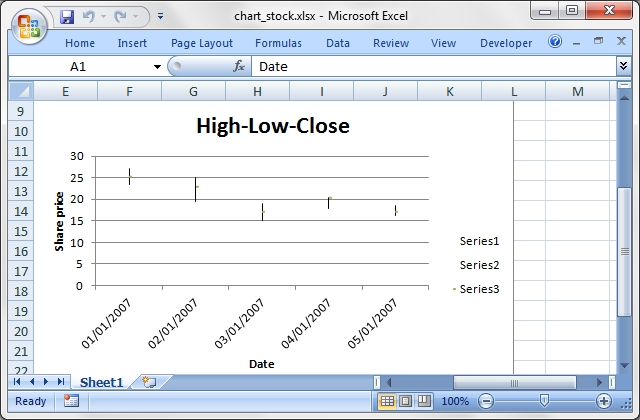Source code for this example:

``````    #!/usr/bin/perl

#######################################################################
#
# A demo of a Stock chart in Excel::Writer::XLSX.
#
# Copyright 2000-2021, John McNamara, jmcnamara@cpan.org
#

use strict;
use warnings;
use Excel::Writer::XLSX;
use Excel::Writer::XLSX;

my \$workbook    = Excel::Writer::XLSX->new( 'chart_stock.xlsx' );
my \$worksheet   = \$workbook->add_worksheet();
my \$bold        = \$workbook->add_format( bold => 1 );
my \$date_format = \$workbook->add_format( num_format => 'dd/mm/yyyy' );
my \$chart       = \$workbook->add_chart( type => 'stock', embedded => 1 );

# Add the worksheet data that the charts will refer to.
my \$headings = [ 'Date', 'High', 'Low', 'Close' ];
my \$data = [

[ '2007-01-01T', '2007-01-02T', '2007-01-03T', '2007-01-04T', '2007-01-05T' ],
[ 27.2,  25.03, 19.05, 20.34, 18.5 ],
[ 23.49, 19.55, 15.12, 17.84, 16.34 ],
[ 25.45, 23.05, 17.32, 20.45, 17.34 ],

];

\$worksheet->write( 'A1', \$headings, \$bold );

for my \$row ( 0 .. 4 ) {
\$worksheet->write_date_time( \$row+1, 0, \$data->->[\$row], \$date_format );
\$worksheet->write( \$row+1, 1, \$data->->[\$row] );
\$worksheet->write( \$row+1, 2, \$data->->[\$row] );
\$worksheet->write( \$row+1, 3, \$data->->[\$row] );

}

\$worksheet->set_column( 'A:D', 11 );

# Add a series for each of the High-Low-Close columns.
categories => '=Sheet1!\$A\$2:\$A\$6',
values     => '=Sheet1!\$B\$2:\$B\$6',
);

categories => '=Sheet1!\$A\$2:\$A\$6',
values     => '=Sheet1!\$C\$2:\$C\$6',
);

categories => '=Sheet1!\$A\$2:\$A\$6',
values     => '=Sheet1!\$D\$2:\$D\$6',
);

# Add a chart title and some axis labels.
\$chart->set_title ( name => 'High-Low-Close', );
\$chart->set_x_axis( name => 'Date', );
\$chart->set_y_axis( name => 'Share price', );

\$worksheet->insert_chart( 'E9', \$chart );

\$workbook->close();

__END__``````

## Example: chart_data_table.pl

A demo of an Column chart with a data table on the X-axis using Excel::Writer::XLSX.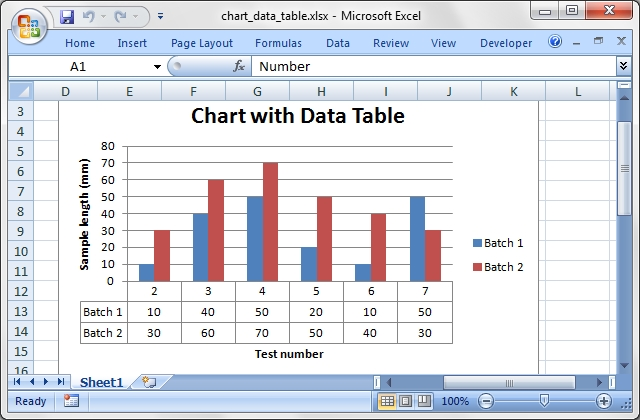Source code for this example:

``````    #!/usr/bin/perl

#######################################################################
#
# A demo of an Column chart with a data table on the X-axis using
# Excel::Writer::XLSX.
#
# Copyright 2000-2021, John McNamara, jmcnamara@cpan.org
#

use strict;
use warnings;
use Excel::Writer::XLSX;

my \$workbook  = Excel::Writer::XLSX->new( 'chart_data_table.xlsx' );
my \$worksheet = \$workbook->add_worksheet();
my \$bold      = \$workbook->add_format( bold => 1 );

# Add the worksheet data that the charts will refer to.
my \$headings = [ 'Number', 'Batch 1', 'Batch 2' ];
my \$data = [
[ 2,  3,  4,  5,  6,  7 ],
[ 10, 40, 50, 20, 10, 50 ],
[ 30, 60, 70, 50, 40, 30 ],

];

\$worksheet->write( 'A1', \$headings, \$bold );
\$worksheet->write( 'A2', \$data );

# Create a column chart with a data table.
my \$chart1 = \$workbook->add_chart( type => 'column', embedded => 1 );

# Configure the first series.
name       => '=Sheet1!\$B\$1',
categories => '=Sheet1!\$A\$2:\$A\$7',
values     => '=Sheet1!\$B\$2:\$B\$7',
);

# Configure second series. Note alternative use of array ref to define
# ranges: [ \$sheetname, \$row_start, \$row_end, \$col_start, \$col_end ].
name       => '=Sheet1!\$C\$1',
categories => [ 'Sheet1', 1, 6, 0, 0 ],
values     => [ 'Sheet1', 1, 6, 2, 2 ],
);

# Add a chart title and some axis labels.
\$chart1->set_title( name => 'Chart with Data Table' );
\$chart1->set_x_axis( name => 'Test number' );
\$chart1->set_y_axis( name => 'Sample length (mm)' );

# Set a default data table on the X-Axis.
\$chart1->set_table();

# Insert the chart into the worksheet (with an offset).
\$worksheet->insert_chart( 'D2', \$chart1, { x_offset => 25, y_offset => 10 } );

#
# Create a second chart.
#
my \$chart2 = \$workbook->add_chart( type => 'column', embedded => 1 );

# Configure the first series.
name       => '=Sheet1!\$B\$1',
categories => '=Sheet1!\$A\$2:\$A\$7',
values     => '=Sheet1!\$B\$2:\$B\$7',
);

# Configure second series.
name       => '=Sheet1!\$C\$1',
categories => [ 'Sheet1', 1, 6, 0, 0 ],
values     => [ 'Sheet1', 1, 6, 2, 2 ],
);

# Add a chart title and some axis labels.
\$chart2->set_title( name => 'Data Table with legend keys' );
\$chart2->set_x_axis( name => 'Test number' );
\$chart2->set_y_axis( name => 'Sample length (mm)' );

# Set a data table on the X-Axis with the legend keys showm.
\$chart2->set_table( show_keys => 1 );

# Hide the chart legend since the keys are show on the data table.
\$chart2->set_legend( position => 'none' );

# Insert the chart into the worksheet (with an offset).
\$worksheet->insert_chart( 'D18', \$chart2, { x_offset => 25, y_offset => 10 } );

\$workbook->close();

__END__``````

## Example: chart_data_tools.pl

A demo of an various Excel chart data tools that are available via an Excel::Writer::XLSX chart.

These include, Trendlines, Data Labels, Error Bars, Drop Lines, High-Low Lines and Up-Down Bars.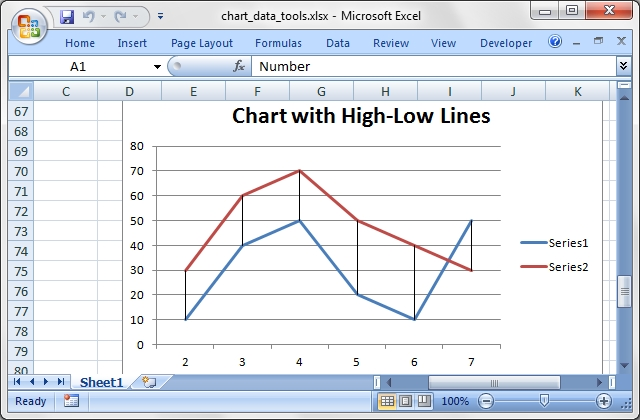Source code for this example:

``````    #!/usr/bin/perl

#######################################################################
#
# A demo of an various Excel chart data tools that are available via
# an Excel::Writer::XLSX chart.
#
# These include, Trendlines, Data Labels, Error Bars, Drop Lines,
# High-Low Lines and Up-Down Bars.
#
# Copyright 2000-2021, John McNamara, jmcnamara@cpan.org
#

use strict;
use warnings;
use Excel::Writer::XLSX;

my \$workbook  = Excel::Writer::XLSX->new( 'chart_data_tools.xlsx' );
my \$worksheet = \$workbook->add_worksheet();
my \$bold      = \$workbook->add_format( bold => 1 );

# Add the worksheet data that the charts will refer to.
my \$headings = [ 'Number', 'Data 1', 'Data 2' ];
my \$data = [
[ 2,  3,  4,  5,  6,  7 ],
[ 10, 40, 50, 20, 10, 50 ],
[ 30, 60, 70, 50, 40, 30 ],

];

\$worksheet->write( 'A1', \$headings, \$bold );
\$worksheet->write( 'A2', \$data );

#######################################################################
#
# Trendline example.
#

# Create a Line chart.
my \$chart1 = \$workbook->add_chart( type => 'line', embedded => 1 );

# Configure the first series with a polynomial trendline.
categories => '=Sheet1!\$A\$2:\$A\$7',
values     => '=Sheet1!\$B\$2:\$B\$7',
trendline  => {
type  => 'polynomial',
order => 3,
},
);

# Configure the second series with a moving average trendline.
categories => '=Sheet1!\$A\$2:\$A\$7',
values     => '=Sheet1!\$C\$2:\$C\$7',
trendline  => { type => 'linear' },
);

# Add a chart title. and some axis labels.
\$chart1->set_title( name => 'Chart with Trendlines' );

# Insert the chart into the worksheet (with an offset).
\$worksheet->insert_chart( 'D2', \$chart1, { x_offset => 25, y_offset => 10 } );

#######################################################################
#
# Data Labels and Markers example.
#

# Create a Line chart.
my \$chart2 = \$workbook->add_chart( type => 'line', embedded => 1 );

# Configure the first series.
categories  => '=Sheet1!\$A\$2:\$A\$7',
values      => '=Sheet1!\$B\$2:\$B\$7',
data_labels => { value => 1 },
marker      => { type => 'automatic' },
);

# Configure the second series.
categories => '=Sheet1!\$A\$2:\$A\$7',
values     => '=Sheet1!\$C\$2:\$C\$7',
);

# Add a chart title. and some axis labels.
\$chart2->set_title( name => 'Chart with Data Labels and Markers' );

# Insert the chart into the worksheet (with an offset).
\$worksheet->insert_chart( 'D18', \$chart2, { x_offset => 25, y_offset => 10 } );

#######################################################################
#
# Error Bars example.
#

# Create a Line chart.
my \$chart3 = \$workbook->add_chart( type => 'line', embedded => 1 );

# Configure the first series.
categories   => '=Sheet1!\$A\$2:\$A\$7',
values       => '=Sheet1!\$B\$2:\$B\$7',
y_error_bars => { type => 'standard_error' },
);

# Configure the second series.
categories => '=Sheet1!\$A\$2:\$A\$7',
values     => '=Sheet1!\$C\$2:\$C\$7',
);

# Add a chart title. and some axis labels.
\$chart3->set_title( name => 'Chart with Error Bars' );

# Insert the chart into the worksheet (with an offset).
\$worksheet->insert_chart( 'D34', \$chart3, { x_offset => 25, y_offset => 10 } );

#######################################################################
#
# Up-Down Bars example.
#

# Create a Line chart.
my \$chart4 = \$workbook->add_chart( type => 'line', embedded => 1 );

# Add the Up-Down Bars.
\$chart4->set_up_down_bars();

# Configure the first series.
categories => '=Sheet1!\$A\$2:\$A\$7',
values     => '=Sheet1!\$B\$2:\$B\$7',
);

# Configure the second series.
categories => '=Sheet1!\$A\$2:\$A\$7',
values     => '=Sheet1!\$C\$2:\$C\$7',
);

# Add a chart title. and some axis labels.
\$chart4->set_title( name => 'Chart with Up-Down Bars' );

# Insert the chart into the worksheet (with an offset).
\$worksheet->insert_chart( 'D50', \$chart4, { x_offset => 25, y_offset => 10 } );

#######################################################################
#
# High-Low Lines example.
#

# Create a Line chart.
my \$chart5 = \$workbook->add_chart( type => 'line', embedded => 1 );

# Add the High-Low lines.
\$chart5->set_high_low_lines();

# Configure the first series.
categories => '=Sheet1!\$A\$2:\$A\$7',
values     => '=Sheet1!\$B\$2:\$B\$7',
);

# Configure the second series.
categories => '=Sheet1!\$A\$2:\$A\$7',
values     => '=Sheet1!\$C\$2:\$C\$7',
);

# Add a chart title. and some axis labels.
\$chart5->set_title( name => 'Chart with High-Low Lines' );

# Insert the chart into the worksheet (with an offset).
\$worksheet->insert_chart( 'D66', \$chart5, { x_offset => 25, y_offset => 10 } );

#######################################################################
#
# Drop Lines example.
#

# Create a Line chart.
my \$chart6 = \$workbook->add_chart( type => 'line', embedded => 1 );

# Add Drop Lines.
\$chart6->set_drop_lines();

# Configure the first series.
categories => '=Sheet1!\$A\$2:\$A\$7',
values     => '=Sheet1!\$B\$2:\$B\$7',
);

# Configure the second series.
categories => '=Sheet1!\$A\$2:\$A\$7',
values     => '=Sheet1!\$C\$2:\$C\$7',
);

# Add a chart title. and some axis labels.
\$chart6->set_title( name => 'Chart with Drop Lines' );

# Insert the chart into the worksheet (with an offset).
\$worksheet->insert_chart( 'D82', \$chart6, { x_offset => 25, y_offset => 10 } );

\$workbook->close();

__END__``````

## Example: chart_data_labels.pl

A demo of an various Excel chart data label features that are available via an Excel::Writer::XLSX chart.

``````    #!/usr/bin/perl

#######################################################################
#
# A demo of an various Excel chart data label features that are available
# via an Excel::Writer::XLSX chart.
#
# Copyright 2000-2021, John McNamara, jmcnamara@cpan.org
#

use strict;
use warnings;
use Excel::Writer::XLSX;

my \$workbook  = Excel::Writer::XLSX->new( 'chart_data_labels.xlsx' );
my \$worksheet = \$workbook->add_worksheet();
my \$bold      = \$workbook->add_format( bold => 1 );

# Add the worksheet data that the charts will refer to.
my \$headings = [ 'Number', 'Data', 'Text' ];
my \$data = [
[ 2,  3,  4,  5,  6,  7 ],
[ 20, 10, 20, 30, 40, 30 ],
[ 'Jan', 'Feb', 'Mar', 'Apr', 'May', 'Jun' ],
];

\$worksheet->write( 'A1', \$headings, \$bold );
\$worksheet->write( 'A2', \$data );

#######################################################################
#
# Example with standard data labels.
#

# Create a Column chart.
my \$chart1 = \$workbook->add_chart( type => 'column', embedded => 1 );

# Configure the data series and add the data labels.
categories => '=Sheet1!\$A\$2:\$A\$7',
values     => '=Sheet1!\$B\$2:\$B\$7',
data_labels => { value => 1 },
);

# Add a chart title.
\$chart1->set_title( name => 'Chart with standard data labels' );

# Turn off the chart legend.
\$chart1->set_legend( none => 1 );

# Insert the chart into the worksheet (with an offset).
\$worksheet->insert_chart( 'D2', \$chart1, { x_offset => 25, y_offset => 10 } );

#######################################################################
#
# Example with value and category data labels.
#

# Create a Column chart.
my \$chart2 = \$workbook->add_chart( type => 'column', embedded => 1 );

# Configure the data series and add the data labels.
categories => '=Sheet1!\$A\$2:\$A\$7',
values     => '=Sheet1!\$B\$2:\$B\$7',
data_labels => { value => 1, category => 1 },
);

# Add a chart title.
\$chart2->set_title( name => 'Category and Value data labels' );

# Turn off the chart legend.
\$chart2->set_legend( none => 1 );

# Insert the chart into the worksheet (with an offset).
\$worksheet->insert_chart( 'D18', \$chart2, { x_offset => 25, y_offset => 10 } );

#######################################################################
#
# Example with standard data labels with different font.
#

# Create a Column chart.
my \$chart3 = \$workbook->add_chart( type => 'column', embedded => 1 );

# Configure the data series and add the data labels.
categories => '=Sheet1!\$A\$2:\$A\$7',
values     => '=Sheet1!\$B\$2:\$B\$7',
data_labels => { value => 1,
font => { bold => 1,
color => 'red',
rotation => -30} },
);

# Add a chart title.
\$chart3->set_title( name => 'Data labels with user defined font' );

# Turn off the chart legend.
\$chart3->set_legend( none => 1 );

# Insert the chart into the worksheet (with an offset).
\$worksheet->insert_chart( 'D34', \$chart3, { x_offset => 25, y_offset => 10 } );

#######################################################################
#
# Example with standard data labels and formatting.
#

# Create a Column chart.
my \$chart4 = \$workbook->add_chart( type => 'column', embedded => 1 );

# Configure the data series and add the data labels.
categories => '=Sheet1!\$A\$2:\$A\$7',
values     => '=Sheet1!\$B\$2:\$B\$7',
data_labels => { value  => 1,
border => {color => 'red'},
fill   => {color => 'yellow'} },
);

# Add a chart title.
\$chart4->set_title( name => 'Data labels with formatting' );

# Turn off the chart legend.
\$chart4->set_legend( none => 1 );

# Insert the chart into the worksheet (with an offset).
\$worksheet->insert_chart( 'D50', \$chart4, { x_offset => 25, y_offset => 10 } );

#######################################################################
#
# Example with custom string data labels.
#

# Create a Column chart.
my \$chart5 = \$workbook->add_chart( type => 'column', embedded => 1 );

# Some custom labels.
my \$custom_labels = [
{ value => 'Amy' },
{ value => 'Bea' },
{ value => 'Eva' },
{ value => 'Fay' },
{ value => 'Liv' },
{ value => 'Una' },
];

# Configure the data series and add the data labels.
categories => '=Sheet1!\$A\$2:\$A\$7',
values     => '=Sheet1!\$B\$2:\$B\$7',
data_labels => { value => 1, custom => \$custom_labels },
);

# Add a chart title.
\$chart5->set_title( name => 'Chart with custom string data labels' );

# Turn off the chart legend.
\$chart5->set_legend( none => 1 );

# Insert the chart into the worksheet (with an offset).
\$worksheet->insert_chart( 'D66', \$chart5, { x_offset => 25, y_offset => 10 } );

#######################################################################
#
# Example with custom data labels from cells.
#

# Create a Column chart.
my \$chart6 = \$workbook->add_chart( type => 'column', embedded => 1 );

# Some custom labels.
\$custom_labels = [
{ value => '=Sheet1!\$C\$2' },
{ value => '=Sheet1!\$C\$3' },
{ value => '=Sheet1!\$C\$4' },
{ value => '=Sheet1!\$C\$5' },
{ value => '=Sheet1!\$C\$6' },
{ value => '=Sheet1!\$C\$7' },
];

# Configure the data series and add the data labels.
categories => '=Sheet1!\$A\$2:\$A\$7',
values     => '=Sheet1!\$B\$2:\$B\$7',
data_labels => { value => 1, custom => \$custom_labels },
);

# Add a chart title.
\$chart6->set_title( name => 'Chart with custom data labels from cells' );

# Turn off the chart legend.
\$chart6->set_legend( none => 1 );

# Insert the chart into the worksheet (with an offset).
\$worksheet->insert_chart( 'D82', \$chart6, { x_offset => 25, y_offset => 10 } );

#######################################################################
#
# Example with custom and default data labels.
#

# Create a Column chart.
my \$chart7 = \$workbook->add_chart( type => 'column', embedded => 1 );

# Some custom labels. The undef items will get the default value.
# We also set a font for the custom items as an extra example.
\$custom_labels = [
{ value => '=Sheet1!\$C\$2', font => { color => 'red' } },
undef,
{ value => '=Sheet1!\$C\$4', font => { color => 'red' } },
{ value => '=Sheet1!\$C\$5', font => { color => 'red' } },
];

# Configure the data series and add the data labels.
categories => '=Sheet1!\$A\$2:\$A\$7',
values     => '=Sheet1!\$B\$2:\$B\$7',
data_labels => { value => 1, custom => \$custom_labels },
);

# Add a chart title.
\$chart7->set_title( name => 'Mixed custom and default data labels' );

# Turn off the chart legend.
\$chart7->set_legend( none => 1 );

# Insert the chart into the worksheet (with an offset).
\$worksheet->insert_chart( 'D98', \$chart7, { x_offset => 25, y_offset => 10 } );

#######################################################################
#
# Example with deleted custom data labels.
#

# Create a Column chart.
my \$chart8 = \$workbook->add_chart( type => 'column', embedded => 1 );

# Some deleted custom labels and defaults (undef). This allows us to
# highlight certain values such as the minimum and maximum.
\$custom_labels = [
{ delete => 1 },
undef,
{ delete => 1 },
{ delete => 1 },
undef,
{ delete => 1 },
];

# Configure the data series and add the data labels.
categories => '=Sheet1!\$A\$2:\$A\$7',
values     => '=Sheet1!\$B\$2:\$B\$7',
data_labels => { value => 1, custom => \$custom_labels },
);

# Add a chart title.
\$chart8->set_title( name => 'Chart with deleted data labels' );

# Turn off the chart legend.
\$chart8->set_legend( none => 1 );

# Insert the chart into the worksheet (with an offset).
\$worksheet->insert_chart( 'D114', \$chart8, { x_offset => 25, y_offset => 10 } );

#######################################################################
#
# Example with custom string data labels and formatting.
#

# Create a Column chart.
my \$chart9 = \$workbook->add_chart( type => 'column', embedded => 1 );

# Some custom labels.
\$custom_labels = [
{ value => 'Amy', border => {color => 'blue'} },
{ value => 'Bea' },
{ value => 'Eva' },
{ value => 'Fay' },
{ value => 'Liv' },
{ value => 'Una', fill   => {color => 'green'} },
];

# Configure the data series and add the data labels.
categories => '=Sheet1!\$A\$2:\$A\$7',
values     => '=Sheet1!\$B\$2:\$B\$7',
data_labels => { value => 1,
custom => \$custom_labels,
border => {color => 'red'},
fill   => {color => 'yellow'} },
);

# Add a chart title.
\$chart9->set_title( name => 'Chart with custom labels and formatting' );

# Turn off the chart legend.
\$chart9->set_legend( none => 1 );

# Insert the chart into the worksheet (with an offset).
\$worksheet->insert_chart( 'D130', \$chart9, { x_offset => 25, y_offset => 10 } );

\$workbook->close();

__END__``````

## Example: chart_clustered.pl

A demo of a clustered category chart in Excel::Writer::XLSX.

``````    #!/usr/bin/perl

#######################################################################
#
# A demo of a clustered category chart in Excel::Writer::XLSX.
#
# Copyright 2000-2021, John McNamara, jmcnamara@cpan.org
#

use strict;
use warnings;
use Excel::Writer::XLSX;

my \$workbook  = Excel::Writer::XLSX->new( 'chart_clustered.xlsx' );
my \$worksheet = \$workbook->add_worksheet();
my \$bold      = \$workbook->add_format( bold => 1 );

# Add the worksheet data that the charts will refer to.
my \$headings = [ 'Types',  'Sub Type',   'Value 1', 'Value 2', 'Value 3' ];
my \$data = [
[ 'Type 1', 'Sub Type A', 5000,      8000,      6000 ],
[ '',       'Sub Type B', 2000,      3000,      4000 ],
[ '',       'Sub Type C', 250,       1000,      2000 ],
[ 'Type 2', 'Sub Type D', 6000,      6000,      6500 ],
[ '',       'Sub Type E', 500,       300,       200 ],
];

\$worksheet->write( 'A1', \$headings, \$bold );
\$worksheet->write_col( 'A2', \$data );

# Create a new chart object. In this case an embedded chart.
my \$chart = \$workbook->add_chart( type => 'column', embedded => 1 );

# Configure the series. Note, that the categories are 2D ranges (from column A
# to column B). This creates the clusters. The series are shown as formula
# strings for clarity but you can also use the array syntax. See the docs.
name       => '=Sheet1!\$C\$1',
categories => '=Sheet1!\$A\$2:\$B\$6',
values     => '=Sheet1!\$C\$2:\$C\$6',
);

name       => '=Sheet1!\$D\$1',
categories => '=Sheet1!\$A\$2:\$B\$6',
values     => '=Sheet1!\$D\$2:\$D\$6',
);

name       => '=Sheet1!\$E\$1',
categories => '=Sheet1!\$A\$2:\$B\$6',
values     => '=Sheet1!\$E\$2:\$E\$6',
);

# Set the Excel chart style.
\$chart->set_style( 37 );

# Turn off the legend.
\$chart->set_legend( position => 'none' );

# Insert the chart into the worksheet.
\$worksheet->insert_chart( 'G3', \$chart );

\$workbook->close();

__END__``````

## Example: chart_styles.pl

An example showing all 48 default chart styles available in Excel 2007 using Excel::Writer::XLSX.. Note, these styles are not the same as the styles available in Excel 2013.

``````    #!/usr/bin/perl

#######################################################################
#
# An example showing all 48 default chart styles available in Excel 2007
# using Excel::Writer::XLSX.. Note, these styles are not the same as the
# styles available in Excel 2013.
#
# Copyright 2000-2021, John McNamara, jmcnamara@cpan.org
#

use strict;
use warnings;
use Excel::Writer::XLSX;

my \$workbook = Excel::Writer::XLSX->new( 'chart_styles.xlsx' );

# Show the styles for all of these chart types.
my @chart_types = ( 'column', 'area', 'line', 'pie' );

for my \$chart_type ( @chart_types ) {

# Add a worksheet for each chart type.
my \$worksheet = \$workbook->add_worksheet( ucfirst( \$chart_type ) );
\$worksheet->set_zoom( 30 );
my \$style_number = 1;

# Create 48 charts, each with a different style.
for ( my \$row_num = 0 ; \$row_num < 90 ; \$row_num += 15 ) {
for ( my \$col_num = 0 ; \$col_num < 64 ; \$col_num += 8 ) {

my \$chart = \$workbook->add_chart(
type     => \$chart_type,
embedded => 1
);

\$chart->add_series( values => '=Data!\$A\$1:\$A\$6' );
\$chart->set_title( name => 'Style ' . \$style_number );
\$chart->set_legend( none => 1 );
\$chart->set_style( \$style_number );

\$worksheet->insert_chart( \$row_num, \$col_num, \$chart );
\$style_number++;
}
}
}

# Create a worksheet with data for the charts.
my \$data = [ 10, 40, 50, 20, 10, 50 ];
my \$data_worksheet = \$workbook->add_worksheet( 'Data' );
\$data_worksheet->write_col( 'A1', \$data );
\$data_worksheet->hide();

\$workbook->close();

__END__``````

## Example: chart_gauge.pl

A demo of an Gauge Chart in Excel::Writer::XLSX.

A Gauge Chart isn't a native chart type in Excel. It is constructed by combining a doughnut chart and a pie chart and by using some non-filled elements. This example follows the following online example of how to create a Gauge Chart in Excel: https://www.excel-easy.com/examples/gauge-chart.html

``````    #!/usr/bin/perl

#######################################################################
#
# A demo of an Gauge Chart in Excel::Writer::XLSX.
#
# A Gauge Chart isn't a native chart type in Excel. It is constructed by
# combining a doughnut chart and a pie chart and by using some non-filled
# elements. This example follows the following online example of how to create
# a Gauge Chart in Excel: https://www.excel-easy.com/examples/gauge-chart.html
#
# Copyright 2000-2021, John McNamara, jmcnamara@cpan.org
#

use strict;
use warnings;
use Excel::Writer::XLSX;

my \$workbook  = Excel::Writer::XLSX->new( 'chart_gauge.xlsx' );
my \$worksheet = \$workbook->add_worksheet();

my \$chart_doughnut = \$workbook->add_chart( type => 'doughnut', embedded => 1 );
my \$chart_pie      = \$workbook->add_chart( type => 'pie', embedded => 1 );

# Add some data for the Doughnut and Pie charts. This is set up so the
# gauge goes from 0-100. It is initially set at 75%.
\$worksheet->write_col( 'H2', ['Donut', 25, 50, 25, 100] );
\$worksheet->write_col( 'I2', ['Pie', 75, 1, '=200-I4-I3'] );

# Configure the doughnut chart as the background for the gauge.
name   => '=Sheet1!\$H\$2',
values => '=Sheet1!\$H\$3:\$H\$6',
points => [
{ fill => { color => 'green' } },
{ fill => { color => 'yellow' } },
{ fill => { color => 'red' } },
{ fill => { none  => 1 } },
],
);

# Rotate chart so the gauge parts are above the horizontal.
\$chart_doughnut->set_rotation( 270 );

# Turn off the chart legend.
\$chart_doughnut->set_legend( none => 1 );

# Turn off the chart fill and border.
\$chart_doughnut->set_chartarea(
border => { none  => 1 },
fill   => { none  => 1 },
);

# Configure the pie chart as the needle for the gauge.
name   => '=Sheet1!\$I\$2',
values => '=Sheet1!\$I\$3:\$I\$6',
points => [
{ fill => { none  => 1 } },
{ fill => { color => 'black' } },
{ fill => { none  => 1 } },
],
);

# Rotate the pie chart/needle to align with the doughnut/gauge.
\$chart_pie->set_rotation( 270 );

# Combine the pie and doughnut charts.
\$chart_doughnut->combine(\$chart_pie);

# Insert the chart into the worksheet.
\$worksheet->insert_chart( 'A1', \$chart_doughnut );

\$workbook->close();``````

## Example: colors.pl

Demonstrates Excel::Writer::XLSX's named colours and the Excel colour palette.

The set_custom_color() Worksheet method can be used to override one of the built-in palette values with a more suitable colour. See the main docs.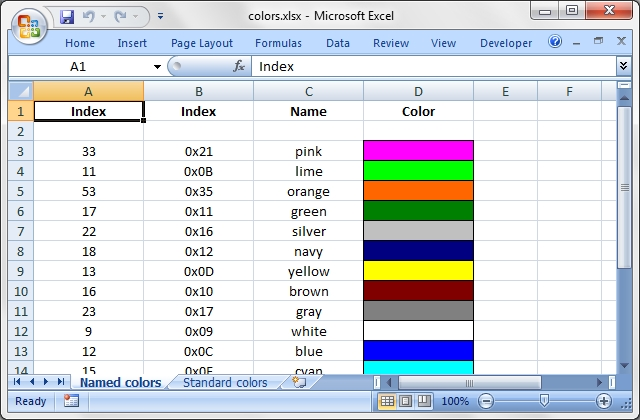Source code for this example:

``````    #!/usr/bin/perl -w

################################################################################
#
# Demonstrates Excel::Writer::XLSX's named colours and the Excel colour
# palette.
#
# The set_custom_color() Worksheet method can be used to override one of the
# built-in palette values with a more suitable colour. See the main docs.
#
# Copyright 2000-2021, John McNamara, jmcnamara@cpan.org
#

use strict;
use Excel::Writer::XLSX;

my \$workbook = Excel::Writer::XLSX->new( 'colors.xlsx' );

# Some common formats
my \$center = \$workbook->add_format( align => 'center' );
my \$heading = \$workbook->add_format( align => 'center', bold => 1 );

######################################################################
#
# Demonstrate the named colors.
#

my %colors = (
0x08, 'black',
0x0C, 'blue',
0x10, 'brown',
0x0F, 'cyan',
0x17, 'gray',
0x11, 'green',
0x0B, 'lime',
0x0E, 'magenta',
0x12, 'navy',
0x35, 'orange',
0x21, 'pink',
0x14, 'purple',
0x0A, 'red',
0x16, 'silver',
0x09, 'white',
0x0D, 'yellow',

);

my \$worksheet1 = \$workbook->add_worksheet( 'Named colors' );

\$worksheet1->set_column( 0, 3, 15 );

\$worksheet1->write( 0, 0, "Index", \$heading );
\$worksheet1->write( 0, 1, "Index", \$heading );
\$worksheet1->write( 0, 2, "Name",  \$heading );
\$worksheet1->write( 0, 3, "Color", \$heading );

my \$i = 1;

while ( my ( \$index, \$color ) = each %colors ) {
my \$format = \$workbook->add_format(
fg_color => \$color,
pattern  => 1,
border   => 1
);

\$worksheet1->write( \$i + 1, 0, \$index, \$center );
\$worksheet1->write( \$i + 1, 1, sprintf( "0x%02X", \$index ), \$center );
\$worksheet1->write( \$i + 1, 2, \$color, \$center );
\$worksheet1->write( \$i + 1, 3, '',     \$format );
\$i++;
}

######################################################################
#
# Demonstrate the standard Excel colors in the range 8..63.
#

my \$worksheet2 = \$workbook->add_worksheet( 'Standard colors' );

\$worksheet2->set_column( 0, 3, 15 );

\$worksheet2->write( 0, 0, "Index", \$heading );
\$worksheet2->write( 0, 1, "Index", \$heading );
\$worksheet2->write( 0, 2, "Color", \$heading );
\$worksheet2->write( 0, 3, "Name",  \$heading );

for my \$i ( 8 .. 63 ) {
my \$format = \$workbook->add_format(
fg_color => \$i,
pattern  => 1,
border   => 1
);

\$worksheet2->write( ( \$i - 7 ), 0, \$i, \$center );
\$worksheet2->write( ( \$i - 7 ), 1, sprintf( "0x%02X", \$i ), \$center );
\$worksheet2->write( ( \$i - 7 ), 2, '', \$format );

# Add the  color names
if ( exists \$colors{\$i} ) {
\$worksheet2->write( ( \$i - 7 ), 3, \$colors{\$i}, \$center );

}
}

######################################################################
#
# Demonstrate the Html colors.
#

%colors = (
'#000000',  'black',
'#0000FF',  'blue',
'#800000',  'brown',
'#00FFFF',  'cyan',
'#808080',  'gray',
'#008000',  'green',
'#00FF00',  'lime',
'#FF00FF',  'magenta',
'#000080',  'navy',
'#FF6600',  'orange',
'#FF00FF',  'pink',
'#800080',  'purple',
'#FF0000',  'red',
'#C0C0C0',  'silver',
'#FFFFFF',  'white',
'#FFFF00',  'yellow',
);

my \$worksheet3 = \$workbook->add_worksheet( 'Html colors' );

\$worksheet3->set_column( 0, 3, 15 );

\$worksheet3->write( 0, 0, "Html", \$heading );
\$worksheet3->write( 0, 1, "Name",  \$heading );
\$worksheet3->write( 0, 2, "Color", \$heading );

\$i = 1;

while ( my ( \$html_color, \$color ) = each %colors ) {
my \$format = \$workbook->add_format(
fg_color => \$html_color,
pattern  => 1,
border   => 1
);

\$worksheet3->write( \$i + 1, 1, \$html_color, \$center );
\$worksheet3->write( \$i + 1, 2, \$color,      \$center );
\$worksheet3->write( \$i + 1, 3, '',          \$format );
\$i++;
}

\$workbook->close();

__END__``````

This example demonstrates writing cell comments.

A cell comment is indicated in Excel by a small red triangle in the upper right-hand corner of the cell.

For more advanced comment options see comments2.pl.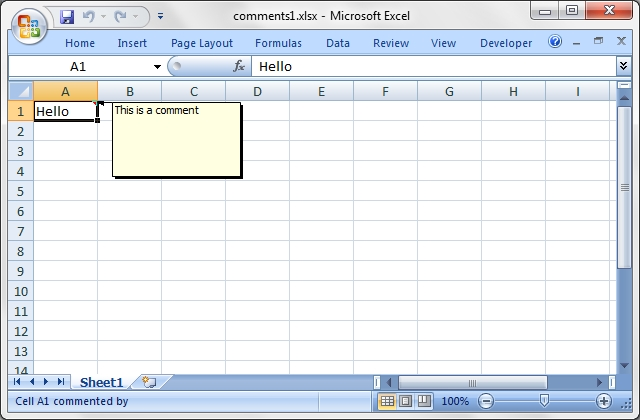Source code for this example:

``````    #!/usr/bin/perl

###############################################################################
#
# This example demonstrates writing cell comments.
#
# A cell comment is indicated in Excel by a small red triangle in the upper
# right-hand corner of the cell.
#
# For more advanced comment options see comments2.pl.
#
# Copyright 2000-2021, John McNamara, jmcnamara@cpan.org
#

use strict;
use warnings;
use Excel::Writer::XLSX;

my \$workbook  = Excel::Writer::XLSX->new( 'comments1.xlsx' );
my \$worksheet = \$workbook->add_worksheet();

\$worksheet->write( 'A1', 'Hello' );
\$worksheet->write_comment( 'A1', 'This is a comment' );

\$workbook->close();

__END__``````

This example demonstrates writing cell comments.

A cell comment is indicated in Excel by a small red triangle in the upper right-hand corner of the cell.

Each of the worksheets demonstrates different features of cell comments.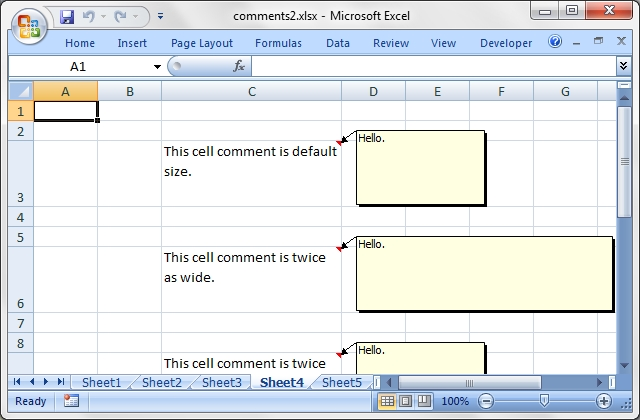Source code for this example:

``````    #!/usr/bin/perl

###############################################################################
#
# This example demonstrates writing cell comments.
#
# A cell comment is indicated in Excel by a small red triangle in the upper
# right-hand corner of the cell.
#
# Each of the worksheets demonstrates different features of cell comments.
#
# Copyright 2000-2021, John McNamara, jmcnamara@cpan.org
#

use strict;
use warnings;
use Excel::Writer::XLSX;

my \$workbook   = Excel::Writer::XLSX->new( 'comments2.xlsx' );
my \$text_wrap  = \$workbook->add_format( text_wrap => 1, valign => 'top' );
my \$worksheet1 = \$workbook->add_worksheet();
my \$worksheet2 = \$workbook->add_worksheet();
my \$worksheet3 = \$workbook->add_worksheet();
my \$worksheet4 = \$workbook->add_worksheet();
my \$worksheet5 = \$workbook->add_worksheet();
my \$worksheet6 = \$workbook->add_worksheet();
my \$worksheet7 = \$workbook->add_worksheet();
my \$worksheet8 = \$workbook->add_worksheet();

# Variables that we will use in each example.
my \$cell_text = '';
my \$comment   = '';

###############################################################################
#
# Example 1. Demonstrates a simple cell comments without formatting.
#

# Set up some formatting.
\$worksheet1->set_column( 'C:C', 25 );
\$worksheet1->set_row( 2, 50 );
\$worksheet1->set_row( 5, 50 );

# Simple ascii string.
\$cell_text = 'Hold the mouse over this cell to see the comment.';

\$comment = 'This is a comment.';

\$worksheet1->write( 'C3', \$cell_text, \$text_wrap );
\$worksheet1->write_comment( 'C3', \$comment );

\$cell_text = 'This is a UTF-8 string.';
\$comment   = chr 0x263a;

\$worksheet1->write( 'C6', \$cell_text, \$text_wrap );
\$worksheet1->write_comment( 'C6', \$comment );

###############################################################################
#
# Example 2. Demonstrates visible and hidden comments.
#

# Set up some formatting.
\$worksheet2->set_column( 'C:C', 25 );
\$worksheet2->set_row( 2, 50 );
\$worksheet2->set_row( 5, 50 );

\$cell_text = 'This cell comment is visible.';

\$comment = 'Hello.';

\$worksheet2->write( 'C3', \$cell_text, \$text_wrap );
\$worksheet2->write_comment( 'C3', \$comment, visible => 1 );

\$cell_text = "This cell comment isn't visible (the default).";

\$comment = 'Hello.';

\$worksheet2->write( 'C6', \$cell_text, \$text_wrap );
\$worksheet2->write_comment( 'C6', \$comment );

###############################################################################
#
# Example 3. Demonstrates visible and hidden comments set at the worksheet
#            level.
#

# Set up some formatting.
\$worksheet3->set_column( 'C:C', 25 );
\$worksheet3->set_row( 2, 50 );
\$worksheet3->set_row( 5, 50 );
\$worksheet3->set_row( 8, 50 );

# Make all comments on the worksheet visible.

\$cell_text = 'This cell comment is visible, explicitly.';

\$comment = 'Hello.';

\$worksheet3->write( 'C3', \$cell_text, \$text_wrap );
\$worksheet3->write_comment( 'C3', \$comment, visible => 1 );

\$cell_text =
'This cell comment is also visible because ' . 'we used show_comments().';

\$comment = 'Hello.';

\$worksheet3->write( 'C6', \$cell_text, \$text_wrap );
\$worksheet3->write_comment( 'C6', \$comment );

\$cell_text = 'However, we can still override it locally.';

\$comment = 'Hello.';

\$worksheet3->write( 'C9', \$cell_text, \$text_wrap );
\$worksheet3->write_comment( 'C9', \$comment, visible => 0 );

###############################################################################
#
# Example 4. Demonstrates changes to the comment box dimensions.
#

# Set up some formatting.
\$worksheet4->set_column( 'C:C', 25 );
\$worksheet4->set_row( 2,  50 );
\$worksheet4->set_row( 5,  50 );
\$worksheet4->set_row( 8,  50 );
\$worksheet4->set_row( 15, 50 );

\$cell_text = 'This cell comment is default size.';

\$comment = 'Hello.';

\$worksheet4->write( 'C3', \$cell_text, \$text_wrap );
\$worksheet4->write_comment( 'C3', \$comment );

\$cell_text = 'This cell comment is twice as wide.';

\$comment = 'Hello.';

\$worksheet4->write( 'C6', \$cell_text, \$text_wrap );
\$worksheet4->write_comment( 'C6', \$comment, x_scale => 2 );

\$cell_text = 'This cell comment is twice as high.';

\$comment = 'Hello.';

\$worksheet4->write( 'C9', \$cell_text, \$text_wrap );
\$worksheet4->write_comment( 'C9', \$comment, y_scale => 2 );

\$cell_text = 'This cell comment is scaled in both directions.';

\$comment = 'Hello.';

\$worksheet4->write( 'C16', \$cell_text, \$text_wrap );
\$worksheet4->write_comment( 'C16', \$comment, x_scale => 1.2, y_scale => 0.8 );

\$cell_text = 'This cell comment has width and height specified in pixels.';

\$comment = 'Hello.';

\$worksheet4->write( 'C19', \$cell_text, \$text_wrap );
\$worksheet4->write_comment( 'C19', \$comment, width => 200, height => 20 );

###############################################################################
#
# Example 5. Demonstrates changes to the cell comment position.
#

\$worksheet5->set_column( 'C:C', 25 );
\$worksheet5->set_row( 2,  50 );
\$worksheet5->set_row( 5,  50 );
\$worksheet5->set_row( 8,  50 );
\$worksheet5->set_row( 11, 50 );

\$cell_text = 'This cell comment is in the default position.';

\$comment = 'Hello.';

\$worksheet5->write( 'C3', \$cell_text, \$text_wrap );
\$worksheet5->write_comment( 'C3', \$comment );

\$cell_text = 'This cell comment has been moved to another cell.';

\$comment = 'Hello.';

\$worksheet5->write( 'C6', \$cell_text, \$text_wrap );
\$worksheet5->write_comment( 'C6', \$comment, start_cell => 'E4' );

\$cell_text = 'This cell comment has been moved to another cell.';

\$comment = 'Hello.';

\$worksheet5->write( 'C9', \$cell_text, \$text_wrap );
\$worksheet5->write_comment( 'C9', \$comment, start_row => 8, start_col => 4 );

\$cell_text = 'This cell comment has been shifted within its default cell.';

\$comment = 'Hello.';

\$worksheet5->write( 'C12', \$cell_text, \$text_wrap );
\$worksheet5->write_comment( 'C12', \$comment, x_offset => 30, y_offset => 12 );

###############################################################################
#
# Example 6. Demonstrates changes to the comment background colour.
#

\$worksheet6->set_column( 'C:C', 25 );
\$worksheet6->set_row( 2, 50 );
\$worksheet6->set_row( 5, 50 );
\$worksheet6->set_row( 8, 50 );

\$cell_text = 'This cell comment has a different colour.';

\$comment = 'Hello.';

\$worksheet6->write( 'C3', \$cell_text, \$text_wrap );
\$worksheet6->write_comment( 'C3', \$comment, color => 'green' );

\$cell_text = 'This cell comment has the default colour.';

\$comment = 'Hello.';

\$worksheet6->write( 'C6', \$cell_text, \$text_wrap );
\$worksheet6->write_comment( 'C6', \$comment );

\$cell_text = 'This cell comment has a different colour.';

\$comment = 'Hello.';

\$worksheet6->write( 'C9', \$cell_text, \$text_wrap );
\$worksheet6->write_comment( 'C9', \$comment, color => '#FF6600' );

###############################################################################
#
# Example 7. Demonstrates how to set the cell comment author.
#

\$worksheet7->set_column( 'C:C', 30 );
\$worksheet7->set_row( 2,  50 );
\$worksheet7->set_row( 5,  50 );
\$worksheet7->set_row( 8,  50 );

my \$author = '';
my \$cell   = 'C3';

\$cell_text = "Move the mouse over this cell and you will see 'Cell commented "
. "by \$author' (blank) in the status bar at the bottom";

\$comment = 'Hello.';

\$worksheet7->write( \$cell, \$cell_text, \$text_wrap );
\$worksheet7->write_comment( \$cell, \$comment );

\$author    = 'Perl';
\$cell      = 'C6';
\$cell_text = "Move the mouse over this cell and you will see 'Cell commented "
. "by \$author' in the status bar at the bottom";

\$comment = 'Hello.';

\$worksheet7->write( \$cell, \$cell_text, \$text_wrap );
\$worksheet7->write_comment( \$cell, \$comment, author => \$author );

\$author    = chr 0x20AC;
\$cell      = 'C9';
\$cell_text = "Move the mouse over this cell and you will see 'Cell commented "
. "by \$author' in the status bar at the bottom";
\$comment = 'Hello.';

\$worksheet7->write( \$cell, \$cell_text, \$text_wrap );
\$worksheet7->write_comment( \$cell, \$comment, author => \$author );

###############################################################################
#
# Example 8. Demonstrates the need to explicitly set the row height.
#

# Set up some formatting.
\$worksheet8->set_column( 'C:C', 25 );
\$worksheet8->set_row( 2, 80 );

\$cell_text =
'The height of this row has been adjusted explicitly using '
. 'set_row(). The size of the comment box is adjusted '
. 'accordingly by Excel::Writer::XLSX.';

\$comment = 'Hello.';

\$worksheet8->write( 'C3', \$cell_text, \$text_wrap );
\$worksheet8->write_comment( 'C3', \$comment );

\$cell_text =
'The height of this row has been adjusted by Excel due to the '
. 'text wrap property being set. Unfortunately this means that '
. 'the height of the row is unknown to Excel::Writer::XLSX at '
. "run time and thus the comment box is stretched as well.\n\n"
. 'Use set_row() to specify the row height explicitly to avoid '
. 'this problem.';

\$comment = 'Hello.';

\$worksheet8->write( 'C6', \$cell_text, \$text_wrap );
\$worksheet8->write_comment( 'C6', \$comment );

\$workbook->close();

__END__``````

## Example: conditional_format.pl

Example of how to add conditional formatting to an Excel::Writer::XLSX file.

Conditional formatting allows you to apply a format to a cell or a range of cells based on certain criteria.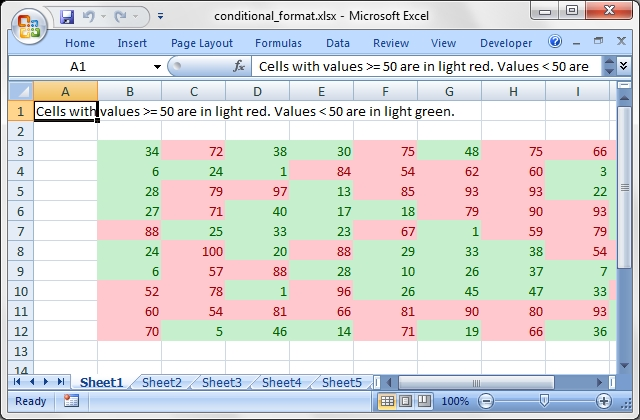Source code for this example:

``````    #!/usr/bin/perl

###############################################################################
#
# Example of how to add conditional formatting to an Excel::Writer::XLSX file.
#
# Conditional formatting allows you to apply a format to a cell or a range of
# cells based on certain criteria.
#
# Copyright 2000-2021, John McNamara, jmcnamara@cpan.org
#

use strict;
use warnings;
use Excel::Writer::XLSX;

my \$workbook   = Excel::Writer::XLSX->new( 'conditional_format.xlsx' );
my \$worksheet1 = \$workbook->add_worksheet();
my \$worksheet2 = \$workbook->add_worksheet();
my \$worksheet3 = \$workbook->add_worksheet();
my \$worksheet4 = \$workbook->add_worksheet();
my \$worksheet5 = \$workbook->add_worksheet();
my \$worksheet6 = \$workbook->add_worksheet();
my \$worksheet7 = \$workbook->add_worksheet();
my \$worksheet8 = \$workbook->add_worksheet();
my \$worksheet9 = \$workbook->add_worksheet();

# Light red fill with dark red text.
my \$format1 = \$workbook->add_format(
bg_color => '#FFC7CE',
color    => '#9C0006',

);

# Green fill with dark green text.
my \$format2 = \$workbook->add_format(
bg_color => '#C6EFCE',
color    => '#006100',

);

# Some sample data to run the conditional formatting against.
my \$data = [
[ 34, 72,  38, 30, 75, 48, 75, 66, 84, 86 ],
[ 6,  24,  1,  84, 54, 62, 60, 3,  26, 59 ],
[ 28, 79,  97, 13, 85, 93, 93, 22, 5,  14 ],
[ 27, 71,  40, 17, 18, 79, 90, 93, 29, 47 ],
[ 88, 25,  33, 23, 67, 1,  59, 79, 47, 36 ],
[ 24, 100, 20, 88, 29, 33, 38, 54, 54, 88 ],
[ 6,  57,  88, 28, 10, 26, 37, 7,  41, 48 ],
[ 52, 78,  1,  96, 26, 45, 47, 33, 96, 36 ],
[ 60, 54,  81, 66, 81, 90, 80, 93, 12, 55 ],
[ 70, 5,   46, 14, 71, 19, 66, 36, 41, 21 ],
];

###############################################################################
#
# Example 1.
#
my \$caption = 'Cells with values >= 50 are in light red. '
. 'Values < 50 are in light green.';

# Write the data.
\$worksheet1->write( 'A1', \$caption );
\$worksheet1->write_col( 'B3', \$data );

# Write a conditional format over a range.
\$worksheet1->conditional_formatting( 'B3:K12',
{
type     => 'cell',
criteria => '>=',
value    => 50,
format   => \$format1,
}
);

# Write another conditional format over the same range.
\$worksheet1->conditional_formatting( 'B3:K12',
{
type     => 'cell',
criteria => '<',
value    => 50,
format   => \$format2,
}
);

###############################################################################
#
# Example 2.
#
\$caption = 'Values between 30 and 70 are in light red. '
. 'Values outside that range are in light green.';

\$worksheet2->write( 'A1', \$caption );
\$worksheet2->write_col( 'B3', \$data );

\$worksheet2->conditional_formatting( 'B3:K12',
{
type     => 'cell',
criteria => 'between',
minimum  => 30,
maximum  => 70,
format   => \$format1,
}
);

\$worksheet2->conditional_formatting( 'B3:K12',
{
type     => 'cell',
criteria => 'not between',
minimum  => 30,
maximum  => 70,
format   => \$format2,
}
);

###############################################################################
#
# Example 3.
#
\$caption = 'Duplicate values are in light red. '
. 'Unique values are in light green.';

\$worksheet3->write( 'A1', \$caption );
\$worksheet3->write_col( 'B3', \$data );

\$worksheet3->conditional_formatting( 'B3:K12',
{
type     => 'duplicate',
format   => \$format1,
}
);

\$worksheet3->conditional_formatting( 'B3:K12',
{
type     => 'unique',
format   => \$format2,
}
);

###############################################################################
#
# Example 4.
#
\$caption = 'Above average values are in light red. '
. 'Below average values are in light green.';

\$worksheet4->write( 'A1', \$caption );
\$worksheet4->write_col( 'B3', \$data );

\$worksheet4->conditional_formatting( 'B3:K12',
{
type     => 'average',
criteria => 'above',
format   => \$format1,
}
);

\$worksheet4->conditional_formatting( 'B3:K12',
{
type     => 'average',
criteria => 'below',
format   => \$format2,
}
);

###############################################################################
#
# Example 5.
#
\$caption = 'Top 10 values are in light red. '
. 'Bottom 10 values are in light green.';

\$worksheet5->write( 'A1', \$caption );
\$worksheet5->write_col( 'B3', \$data );

\$worksheet5->conditional_formatting( 'B3:K12',
{
type     => 'top',
value    => '10',
format   => \$format1,
}
);

\$worksheet5->conditional_formatting( 'B3:K12',
{
type     => 'bottom',
value    => '10',
format   => \$format2,
}
);

###############################################################################
#
# Example 6.
#
\$caption = 'Cells with values >= 50 are in light red. '
. 'Values < 50 are in light green. Non-contiguous ranges.';

# Write the data.
\$worksheet6->write( 'A1', \$caption );
\$worksheet6->write_col( 'B3', \$data );

# Write a conditional format over a range.
\$worksheet6->conditional_formatting( 'B3:K6,B9:K12',
{
type     => 'cell',
criteria => '>=',
value    => 50,
format   => \$format1,
}
);

# Write another conditional format over the same range.
\$worksheet6->conditional_formatting( 'B3:K6,B9:K12',
{
type     => 'cell',
criteria => '<',
value    => 50,
format   => \$format2,
}
);

###############################################################################
#
# Example 7.
#
\$caption = 'Examples of color scales with default and user colors.';

\$data = [ 1 .. 12 ];

\$worksheet7->write( 'A1', \$caption );

\$worksheet7->write    ( 'B2', "2 Color Scale" );
\$worksheet7->write_col( 'B3', \$data );

\$worksheet7->write    ( 'D2', "2 Color Scale + user colors" );
\$worksheet7->write_col( 'D3', \$data );

\$worksheet7->write    ( 'G2', "3 Color Scale" );
\$worksheet7->write_col( 'G3', \$data );

\$worksheet7->write    ( 'I2', "3 Color Scale + user colors" );
\$worksheet7->write_col( 'I3', \$data );

\$worksheet7->conditional_formatting( 'B3:B14',
{
type => '2_color_scale',
}
);

\$worksheet7->conditional_formatting( 'D3:D14',
{
type => '3_color_scale',
}
);

\$worksheet7->conditional_formatting( 'G3:G14',
{
type      => '2_color_scale',
min_color => "#FF0000",
max_color => "#00FF00",

}
);

\$worksheet7->conditional_formatting( 'I3:I14',
{
type      => '3_color_scale',
min_color => "#C5D9F1",
mid_color => "#8DB4E3",
max_color => "#538ED5",
}
);

###############################################################################
#
# Example 8.
#
\$caption = 'Examples of data bars.';

\$data = [ 1 .. 12 ];

\$worksheet8->write( 'A1', \$caption );

\$worksheet8->write    ( 'B2', "Default data bars" );
\$worksheet8->write_col( 'B3', \$data );

\$worksheet8->write    ( 'D2', "Bars only" );
\$worksheet8->write_col( 'D3', \$data );

\$worksheet8->write    ( 'F2', "With user color" );
\$worksheet8->write_col( 'F3', \$data );

\$worksheet8->write    ( 'H2', "Solid bars" );
\$worksheet8->write_col( 'H3', \$data );

\$worksheet8->write    ( 'J2', "Right to left" );
\$worksheet8->write_col( 'J3', \$data );

\$data = [-1, -2, -3, -2, -1, 0, 1, 2, 3, 2, 1, 0];

\$worksheet8->write    ( 'L2', "Excel 2010 style" );
\$worksheet8->write_col( 'L3', \$data );

\$worksheet8->write    ( 'N2', "Negative same as positive" );
\$worksheet8->write_col( 'N3', \$data );

\$worksheet8->conditional_formatting( 'B3:B14',
{
type      => 'data_bar'
}
);

\$worksheet8->conditional_formatting( 'D3:D14',
{
type     => 'data_bar',
bar_only => 1
}
);

\$worksheet8->conditional_formatting( 'F3:F14',
{
type      => 'data_bar',
bar_color => '#63C384'
}
);

\$worksheet8->conditional_formatting( 'H3:H14',
{
type      => 'data_bar',
bar_solid => 1
}
);

\$worksheet8->conditional_formatting( 'J3:J14',
{
type          => 'data_bar',
bar_direction => 'right'
}
);

\$worksheet8->conditional_formatting( 'L3:L14',
{
type          => 'data_bar',
data_bar_2010 => 1
}
);

\$worksheet8->conditional_formatting( 'N3:N14',
{
type                           => 'data_bar',
bar_negative_color_same        => 1,
bar_negative_border_color_same => 1
}
);

###############################################################################
#
# Example 9.
#
\$caption = 'Examples of conditional formats with icon sets.';

\$data = [
[ 1, 2, 3 ],
[ 1, 2, 3 ],
[ 1, 2, 3 ],
[ 1, 2, 3 ],
[ 1, 2, 3, 4 ],
[ 1, 2, 3, 4, 5 ],
[ 1, 2, 3, 4, 5 ],
];

\$worksheet9->write( 'A1', \$caption );
\$worksheet9->write_col( 'B3', \$data );

\$worksheet9->conditional_formatting( 'B3:D3',
{
type         => 'icon_set',
icon_style   => '3_traffic_lights',
}
);

\$worksheet9->conditional_formatting( 'B4:D4',
{
type         => 'icon_set',
icon_style   => '3_traffic_lights',
reverse_icons => 1,
}
);

\$worksheet9->conditional_formatting( 'B5:D5',
{
type         => 'icon_set',
icon_style   => '3_traffic_lights',
icons_only   => 1,
}
);

\$worksheet9->conditional_formatting( 'B6:D6',
{
type         => 'icon_set',
icon_style   => '3_arrows',
}
);

\$worksheet9->conditional_formatting( 'B7:E8',
{
type         => 'icon_set',
icon_style   => '4_arrows',
}
);

\$worksheet9->conditional_formatting( 'B8:F8',
{
type         => 'icon_set',
icon_style   => '5_arrows',
}
);

\$worksheet9->conditional_formatting( 'B9:F9',
{
type         => 'icon_set',
icon_style   => '5_ratings',
}
);

\$workbook->close();

__END__``````

## Example: data_validate.pl

Example of how to add data validation and dropdown lists to an Excel::Writer::XLSX file.

Data validation is a feature of Excel which allows you to restrict the data that a user enters in a cell and to display help and warning messages. It also allows you to restrict input to values in a drop down list.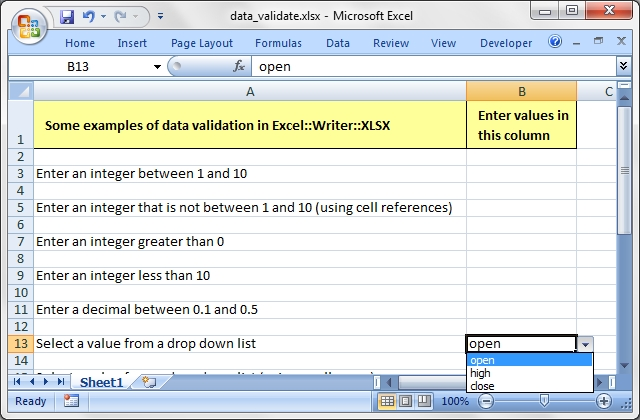Source code for this example:

``````    #!/usr/bin/perl

###############################################################################
#
# Example of how to add data validation and dropdown lists to an
# Excel::Writer::XLSX file.
#
# Data validation is a feature of Excel which allows you to restrict the data
# that a user enters in a cell and to display help and warning messages. It
# also allows you to restrict input to values in a drop down list.
#
# Copyright 2000-2021, John McNamara, jmcnamara@cpan.org
#

use strict;
use warnings;
use Excel::Writer::XLSX;

my \$workbook  = Excel::Writer::XLSX->new( 'data_validate.xlsx' );
my \$worksheet = \$workbook->add_worksheet();

# Add a format for the header cells.
border    => 1,
bg_color  => '#C6EFCE',
bold      => 1,
text_wrap => 1,
valign    => 'vcenter',
indent    => 1,
);

# Set up layout of the worksheet.
\$worksheet->set_column( 'A:A', 68 );
\$worksheet->set_column( 'B:B', 15 );
\$worksheet->set_column( 'D:D', 15 );
\$worksheet->set_row( 0, 36 );
\$worksheet->set_selection( 'B3' );

# Write the header cells and some data that will be used in the examples.
my \$row = 0;
my \$txt;
my \$heading1 = 'Some examples of data validation in Excel::Writer::XLSX';
my \$heading2 = 'Enter values in this column';
my \$heading3 = 'Sample Data';

\$worksheet->write( 'D3', [ 'Integers', 1, 10 ] );
\$worksheet->write( 'D4', [ 'List data', 'open', 'high', 'close' ] );
\$worksheet->write( 'D5', [ 'Formula', '=AND(F5=50,G5=60)', 50, 60 ] );

#
# Example 1. Limiting input to an integer in a fixed range.
#
\$txt = 'Enter an integer between 1 and 10';
\$row += 2;

\$worksheet->write( \$row, 0, \$txt );
\$worksheet->data_validation(
\$row, 1,
{
validate => 'integer',
criteria => 'between',
minimum  => 1,
maximum  => 10,
}
);

#
# Example 2. Limiting input to an integer outside a fixed range.
#
\$txt = 'Enter an integer that is not between 1 and 10 (using cell references)';
\$row += 2;

\$worksheet->write( \$row, 0, \$txt );
\$worksheet->data_validation(
\$row, 1,
{
validate => 'integer',
criteria => 'not between',
minimum  => '=E3',
maximum  => '=F3',
}
);

#
# Example 3. Limiting input to an integer greater than a fixed value.
#
\$txt = 'Enter an integer greater than 0';
\$row += 2;

\$worksheet->write( \$row, 0, \$txt );
\$worksheet->data_validation(
\$row, 1,
{
validate => 'integer',
criteria => '>',
value    => 0,
}
);

#
# Example 4. Limiting input to an integer less than a fixed value.
#
\$txt = 'Enter an integer less than 10';
\$row += 2;

\$worksheet->write( \$row, 0, \$txt );
\$worksheet->data_validation(
\$row, 1,
{
validate => 'integer',
criteria => '<',
value    => 10,
}
);

#
# Example 5. Limiting input to a decimal in a fixed range.
#
\$txt = 'Enter a decimal between 0.1 and 0.5';
\$row += 2;

\$worksheet->write( \$row, 0, \$txt );
\$worksheet->data_validation(
\$row, 1,
{
validate => 'decimal',
criteria => 'between',
minimum  => 0.1,
maximum  => 0.5,
}
);

#
# Example 6. Limiting input to a value in a dropdown list.
#
\$txt = 'Select a value from a drop down list';
\$row += 2;

\$worksheet->write( \$row, 0, \$txt );
\$worksheet->data_validation(
\$row, 1,
{
validate => 'list',
source   => [ 'open', 'high', 'close' ],
}
);

#
# Example 6. Limiting input to a value in a dropdown list.
#
\$txt = 'Select a value from a drop down list (using a cell range)';
\$row += 2;

\$worksheet->write( \$row, 0, \$txt );
\$worksheet->data_validation(
\$row, 1,
{
validate => 'list',
source   => '=\$E\$4:\$G\$4',
}
);

#
# Example 7. Limiting input to a date in a fixed range.
#
\$txt = 'Enter a date between 1/1/2008 and 12/12/2008';
\$row += 2;

\$worksheet->write( \$row, 0, \$txt );
\$worksheet->data_validation(
\$row, 1,
{
validate => 'date',
criteria => 'between',
minimum  => '2008-01-01T',
maximum  => '2008-12-12T',
}
);

#
# Example 8. Limiting input to a time in a fixed range.
#
\$txt = 'Enter a time between 6:00 and 12:00';
\$row += 2;

\$worksheet->write( \$row, 0, \$txt );
\$worksheet->data_validation(
\$row, 1,
{
validate => 'time',
criteria => 'between',
minimum  => 'T06:00',
maximum  => 'T12:00',
}
);

#
# Example 9. Limiting input to a string greater than a fixed length.
#
\$txt = 'Enter a string longer than 3 characters';
\$row += 2;

\$worksheet->write( \$row, 0, \$txt );
\$worksheet->data_validation(
\$row, 1,
{
validate => 'length',
criteria => '>',
value    => 3,
}
);

#
# Example 10. Limiting input based on a formula.
#
\$txt = 'Enter a value if the following is true "=AND(F5=50,G5=60)"';
\$row += 2;

\$worksheet->write( \$row, 0, \$txt );
\$worksheet->data_validation(
\$row, 1,
{
validate => 'custom',
value    => '=AND(F5=50,G5=60)',
}
);

#
# Example 11. Displaying and modify data validation messages.
#
\$txt = 'Displays a message when you select the cell';
\$row += 2;

\$worksheet->write( \$row, 0, \$txt );
\$worksheet->data_validation(
\$row, 1,
{
validate      => 'integer',
criteria      => 'between',
minimum       => 1,
maximum       => 100,
input_title   => 'Enter an integer:',
input_message => 'between 1 and 100',
}
);

#
# Example 12. Displaying and modify data validation messages.
#
\$txt = 'Display a custom error message when integer isn\'t between 1 and 100';
\$row += 2;

\$worksheet->write( \$row, 0, \$txt );
\$worksheet->data_validation(
\$row, 1,
{
validate      => 'integer',
criteria      => 'between',
minimum       => 1,
maximum       => 100,
input_title   => 'Enter an integer:',
input_message => 'between 1 and 100',
error_title   => 'Input value is not valid!',
error_message => 'It should be an integer between 1 and 100',
}
);

#
# Example 13. Displaying and modify data validation messages.
#
\$txt =
'Display a custom information message when integer isn\'t between 1 and 100';
\$row += 2;

\$worksheet->write( \$row, 0, \$txt );
\$worksheet->data_validation(
\$row, 1,
{
validate      => 'integer',
criteria      => 'between',
minimum       => 1,
maximum       => 100,
input_title   => 'Enter an integer:',
input_message => 'between 1 and 100',
error_title   => 'Input value is not valid!',
error_message => 'It should be an integer between 1 and 100',
error_type    => 'information',
}
);

\$workbook->close();

__END__
``````

## Example: date_time.pl

Excel::Writer::XLSX example of writing dates and times using the write_date_time() Worksheet method.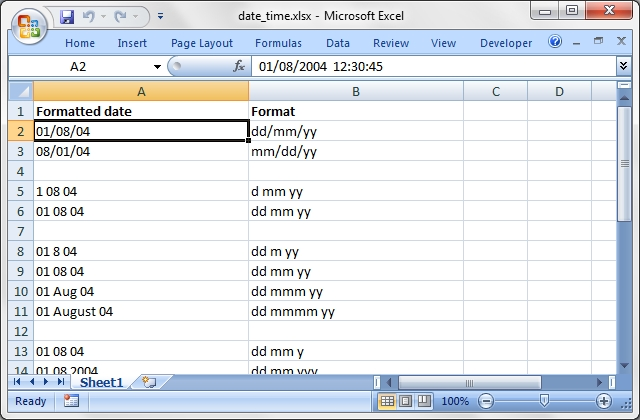Source code for this example:

``````    #!/usr/bin/perl

###############################################################################
#
# Excel::Writer::XLSX example of writing dates and times using the
# write_date_time() Worksheet method.
#
# Copyright 2000-2021, John McNamara, jmcnamara@cpan.org
#

use strict;
use warnings;
use Excel::Writer::XLSX;

# Create a new workbook and add a worksheet
my \$workbook  = Excel::Writer::XLSX->new( 'date_time.xlsx' );
my \$worksheet = \$workbook->add_worksheet();
my \$bold      = \$workbook->add_format( bold => 1 );

# Expand the first columns so that the date is visible.
\$worksheet->set_column( "A:B", 30 );

# Write the column headers
\$worksheet->write( 'A1', 'Formatted date', \$bold );
\$worksheet->write( 'B1', 'Format',         \$bold );

# Examples date and time formats. In the output file compare how changing
# the format codes change the appearance of the date.
#
my @date_formats = (
'dd/mm/yy',
'mm/dd/yy',
'',
'd mm yy',
'dd mm yy',
'',
'dd m yy',
'dd mm yy',
'dd mmm yy',
'dd mmmm yy',
'',
'dd mm y',
'dd mm yyy',
'dd mm yyyy',
'',
'd mmmm yyyy',
'',
'dd/mm/yy',
'dd/mm/yy hh:mm',
'dd/mm/yy hh:mm:ss',
'dd/mm/yy hh:mm:ss.000',
'',
'hh:mm',
'hh:mm:ss',
'hh:mm:ss.000',
);

# Write the same date and time using each of the above formats. The empty
# string formats create a blank line to make the example clearer.
#
my \$row = 0;
for my \$date_format ( @date_formats ) {
\$row++;
next if \$date_format eq '';

# Create a format for the date or time.
my \$format = \$workbook->add_format(
num_format => \$date_format,
align      => 'left'
);

# Write the same date using different formats.
\$worksheet->write_date_time( \$row, 0, '2004-08-01T12:30:45.123', \$format );
\$worksheet->write( \$row, 1, \$date_format );
}

# The following is an example of an invalid date. It is written as a string
# instead of a number. This is also Excel's default behaviour.
#
\$row += 2;
\$worksheet->write_date_time( \$row, 0, '2004-13-01T12:30:45.123' );
\$worksheet->write( \$row, 1, 'Invalid date. Written as string.', \$bold );

\$workbook->close();

__END__
``````

## Example: defined_name.pl

Example of how to create defined names in an Excel::Writer::XLSX file.

This method is used to define a user friendly name to represent a value, a single cell or a range of cells in a workbook.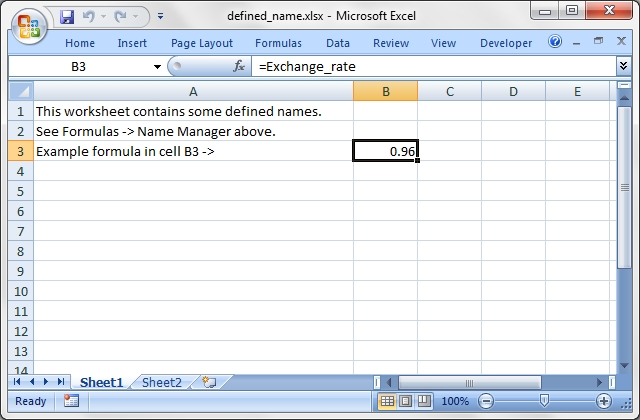Source code for this example:

``````    #!/usr/bin/perl

##############################################################################
#
# Example of how to create defined names in an Excel::Writer::XLSX file.
#
# This method is used to define a user friendly name to represent a value,
# a single cell or a range of cells in a workbook.
#
# Copyright 2000-2021, John McNamara, jmcnamara@cpan.org
#

use strict;
use warnings;
use Excel::Writer::XLSX;

my \$workbook   = Excel::Writer::XLSX->new( 'defined_name.xlsx' );
my \$worksheet1 = \$workbook->add_worksheet();
my \$worksheet2 = \$workbook->add_worksheet();

# Define some global/workbook names.
\$workbook->define_name( 'Exchange_rate', '=0.96' );
\$workbook->define_name( 'Sales',         '=Sheet1!\$G\$1:\$H\$10' );

# Define a local/worksheet name.
\$workbook->define_name( 'Sheet2!Sales', '=Sheet2!\$G\$1:\$G\$10' );

# Write some text in the file and one of the defined names in a formula.
for my \$worksheet ( \$workbook->sheets() ) {
\$worksheet->set_column( 'A:A', 45 );
\$worksheet->write( 'A1', 'This worksheet contains some defined names.' );
\$worksheet->write( 'A2', 'See Formulas -> Name Manager above.' );
\$worksheet->write( 'A3', 'Example formula in cell B3 ->' );

\$worksheet->write( 'B3', '=Exchange_rate' );
}

\$workbook->close();

__END__``````

## Example: diag_border.pl

A simple formatting example that demonstrates how to add a diagonal cell border with Excel::Writer::XLSX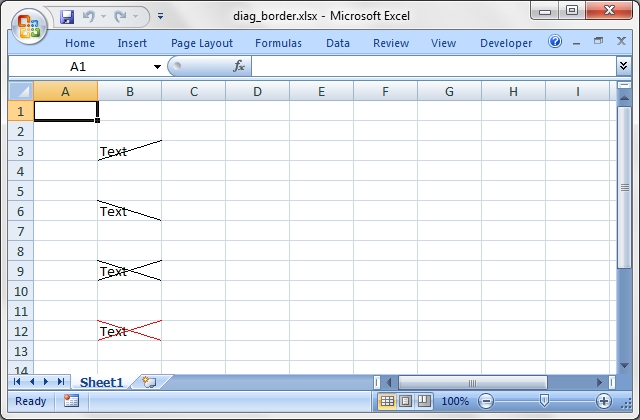Source code for this example:

``````    #!/usr/bin/perl -w

##############################################################################
#
# A simple formatting example that demonstrates how to add a diagonal cell
# border with Excel::Writer::XLSX
#
# Copyright 2000-2021, John McNamara, jmcnamara@cpan.org
#

use strict;
use Excel::Writer::XLSX;

my \$workbook  = Excel::Writer::XLSX->new( 'diag_border.xlsx' );
my \$worksheet = \$workbook->add_worksheet();

my \$format1 = \$workbook->add_format( diag_type => 1 );

my \$format2 = \$workbook->add_format( diag_type => 2 );

my \$format3 = \$workbook->add_format( diag_type => 3 );

my \$format4 = \$workbook->add_format(
diag_type   => 3,
diag_border => 7,
diag_color  => 'red',
);

\$worksheet->write( 'B3',  'Text', \$format1 );
\$worksheet->write( 'B6',  'Text', \$format2 );
\$worksheet->write( 'B9',  'Text', \$format3 );
\$worksheet->write( 'B12', 'Text', \$format4 );

\$workbook->close();

__END__
``````

## Example: filehandle.pl

Example of using Excel::Writer::XLSX to write Excel files to different filehandles.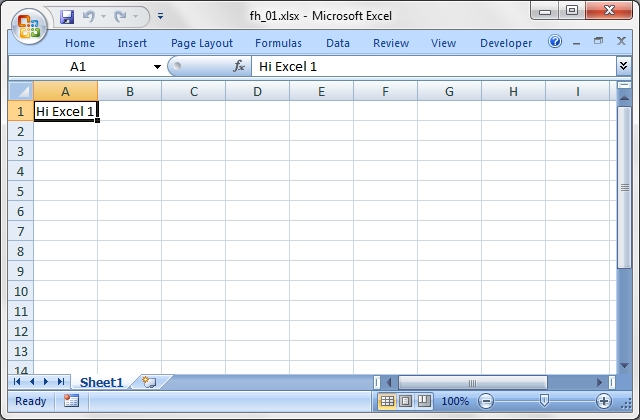Source code for this example:

``````    #!/usr/bin/perl

###############################################################################
#
# Example of using Excel::Writer::XLSX to write Excel files to different
# filehandles.
#
# Copyright 2000-2021, John McNamara, jmcnamara@cpan.org
#

use strict;
use warnings;
use Excel::Writer::XLSX;
use IO::Scalar;

###############################################################################
#
# Example 1. This demonstrates the standard way of creating an Excel file by
# specifying a file name.
#

my \$workbook1  = Excel::Writer::XLSX->new( 'fh_01.xlsx' );
my \$worksheet1 = \$workbook1->add_worksheet();

\$worksheet1->write( 0, 0, 'Hi Excel 1' );

\$workbook1->close();

###############################################################################
#
# Example 2. Write an Excel file to an existing filehandle.
#

open TEST, '>', 'fh_02.xlsx' or die "Couldn't open file: \$!";
binmode TEST;   # Always do this regardless of whether the platform requires it.

my \$workbook2  = Excel::Writer::XLSX->new( \*TEST );
my \$worksheet2 = \$workbook2->add_worksheet();

\$worksheet2->write( 0, 0, 'Hi Excel 2' );

\$workbook2->close();

###############################################################################
#
# Example 3. Write an Excel file to an existing OO style filehandle.
#

my \$fh = FileHandle->new( '> fh_03.xlsx' ) or die "Couldn't open file: \$!";

binmode( \$fh );

my \$workbook3  = Excel::Writer::XLSX->new( \$fh );
my \$worksheet3 = \$workbook3->add_worksheet();

\$worksheet3->write( 0, 0, 'Hi Excel 3' );

\$workbook3->close();

###############################################################################
#
# Example 4. Write an Excel file to a string via IO::Scalar. Please refer to
# the IO::Scalar documentation for further details.
#

my \$xlsx_str;

tie *XLSX, 'IO::Scalar', \\$xlsx_str;

my \$workbook4  = Excel::Writer::XLSX->new( \*XLSX );
my \$worksheet4 = \$workbook4->add_worksheet();

\$worksheet4->write( 0, 0, 'Hi Excel 4' );
\$workbook4->close();    # This is required before we use the scalar

# The Excel file is now in \$xlsx_str. As a demonstration, print it to a file.
open TMP, '>', 'fh_04.xlsx' or die "Couldn't open file: \$!";
binmode TMP;
print TMP \$xlsx_str;
close TMP;

###############################################################################
#
# Example 5. Write an Excel file to a string via IO::Scalar's newer interface.
# Please refer to the IO::Scalar documentation for further details.
#
my \$xlsx_str2;

my \$fh5 = IO::Scalar->new( \\$xlsx_str2 );

my \$workbook5  = Excel::Writer::XLSX->new( \$fh5 );
my \$worksheet5 = \$workbook5->add_worksheet();

\$worksheet5->write( 0, 0, 'Hi Excel 5' );
\$workbook5->close();    # This is required before we use the scalar

# The Excel file is now in \$xlsx_str. As a demonstration, print it to a file.
open TMP, '>', 'fh_05.xlsx' or die "Couldn't open file: \$!";
binmode TMP;
print TMP \$xlsx_str2;
close TMP;

__END__``````

This program shows several examples of how to set up headers and footers with Excel::Writer::XLSX.

The control characters used in the header/footer strings are:

``````    Control             Category            Description
=======             ========            ===========
&L                  Justification       Left
&C                                      Center
&R                                      Right

&P                  Information         Page number
&N                                      Total number of pages
&D                                      Date
&T                                      Time
&F                                      File name
&A                                      Worksheet name

&fontsize           Font                Font size
&"font,style"                           Font name and style
&U                                      Single underline
&E                                      Double underline
&S                                      Strikethrough
&X                                      Superscript
&Y                                      Subscript

&[Picture]          Images              Image placeholder
&G                                      Same as &[Picture]

&&                  Miscellaneous       Literal ampersand &``````

See the main Excel::Writer::XLSX documentation for more information.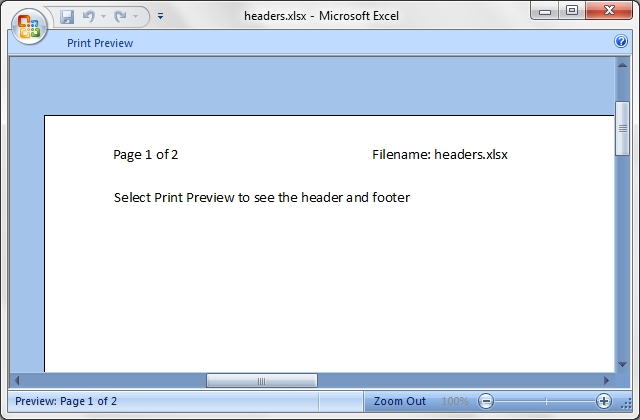Source code for this example:

``````    #!/usr/bin/perl

######################################################################
#
# This program shows several examples of how to set up headers and
# footers with Excel::Writer::XLSX.
#
# The control characters used in the header/footer strings are:
#
#     Control             Category            Description
#     =======             ========            ===========
#     &L                  Justification       Left
#     &C                                      Center
#     &R                                      Right
#
#     &P                  Information         Page number
#     &N                                      Total number of pages
#     &D                                      Date
#     &T                                      Time
#     &F                                      File name
#     &A                                      Worksheet name
#
#     &fontsize           Font                Font size
#     &"font,style"                           Font name and style
#     &U                                      Single underline
#     &E                                      Double underline
#     &S                                      Strikethrough
#     &X                                      Superscript
#     &Y                                      Subscript
#
#     &[Picture]          Images              Image placeholder
#     &G                                      Same as &[Picture]
#
#     &&                  Miscellaneous       Literal ampersand &
#
# See the main Excel::Writer::XLSX documentation for more information.
#
# Copyright 2000-2021, John McNamara, jmcnamara@cpan.org
#

use strict;
use warnings;
use Excel::Writer::XLSX;

my \$workbook = Excel::Writer::XLSX->new( 'headers.xlsx' );
my \$preview  = 'Select Print Preview to see the header and footer';

######################################################################
#
# A simple example to start
#
my \$worksheet1 = \$workbook->add_worksheet( 'Simple' );
my \$header1    = '&CHere is some centred text.';
my \$footer1    = '&LHere is some left aligned text.';

\$worksheet1->set_footer( \$footer1 );

\$worksheet1->set_column( 'A:A', 50 );
\$worksheet1->write( 'A1', \$preview );

######################################################################
#
# A simple example to start
#
my \$worksheet2 = \$workbook->add_worksheet( 'Image' );
my \$header2    = '&L&[Picture]';

# Adjust the page top margin to allow space for the header image.
\$worksheet2->set_margin_top(1.75);

\$worksheet2->set_column( 'A:A', 50 );
\$worksheet2->write( 'A1', \$preview );

######################################################################
#
# This is an example of some of the header/footer variables.
#
my \$worksheet3 = \$workbook->add_worksheet( 'Variables' );
my \$header3    = '&LPage &P of &N' . '&CFilename: &F' . '&RSheetname: &A';
my \$footer3    = '&LCurrent date: &D' . '&RCurrent time: &T';

\$worksheet3->set_footer( \$footer3 );

\$worksheet3->set_column( 'A:A', 50 );
\$worksheet3->write( 'A1',  \$preview );
\$worksheet3->write( 'A21', 'Next sheet' );
\$worksheet3->set_h_pagebreaks( 20 );

######################################################################
#
# This example shows how to use more than one font
#
my \$worksheet4 = \$workbook->add_worksheet( 'Mixed fonts' );
my \$header4    = q(&C&"Courier New,Bold"Hello &"Arial,Italic"World);
my \$footer4    = q(&C&"Symbol"e&"Arial" = mc&X2);

\$worksheet4->set_footer( \$footer4 );

\$worksheet4->set_column( 'A:A', 50 );
\$worksheet4->write( 'A1', \$preview );

######################################################################
#
# Example of line wrapping
#
my \$worksheet5 = \$workbook->add_worksheet( 'Word wrap' );

\$worksheet5->set_column( 'A:A', 50 );
\$worksheet5->write( 'A1', \$preview );

######################################################################
#
# Example of inserting a literal ampersand &
#
my \$worksheet6 = \$workbook->add_worksheet( 'Ampersand' );
my \$header6    = '&CCuriouser && Curiouser - Attorneys at Law';

\$worksheet6->set_column( 'A:A', 50 );
\$worksheet6->write( 'A1', \$preview );

\$workbook->close();

__END__``````

## Example: hide_row_col.pl

Example of how to hide rows and columns in Excel::Writer::XLSX. In order to hide rows without setting each one, (of approximately 1 million rows), Excel uses an optimisation to hide all rows that don't have data.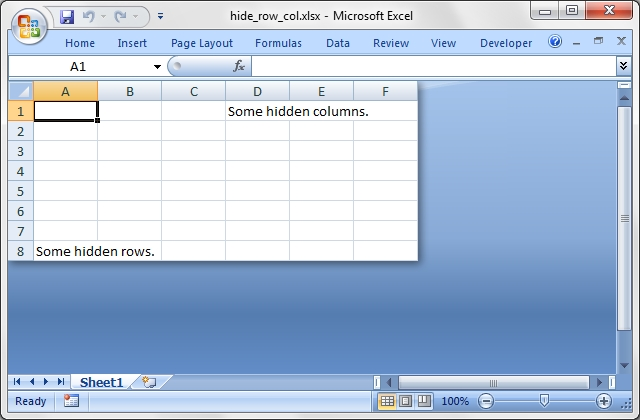Source code for this example:

``````    #!/usr/bin/perl

###############################################################################
#
# Example of how to hide rows and columns in Excel::Writer::XLSX. In order to
# hide rows without setting each one, (of approximately 1 million rows),
# Excel uses an optimisation to hide all rows that don't have data.
#
# Copyright 2000-2021, John McNamara, jmcnamara@cpan.org
#

use strict;
use warnings;
use Excel::Writer::XLSX;

my \$workbook  = Excel::Writer::XLSX->new( 'hide_row_col.xlsx' );
my \$worksheet = \$workbook->add_worksheet();

# Write some data.
\$worksheet->write( 'D1', 'Some hidden columns.' );
\$worksheet->write( 'A8', 'Some hidden rows.' );

# Hide all rows without data.
\$worksheet->set_default_row( undef, 1 );

# Set emptys row that we do want to display. All other will be hidden.
for my \$row (1 .. 6) {
\$worksheet->set_row( \$row, 15 );
}

# Hide a range of columns.
\$worksheet->set_column( 'G:XFD', undef, undef, 1);

\$workbook->close();

__END__

``````

## Example: hide_sheet.pl

Example of how to hide a worksheet with Excel::Writer::XLSX.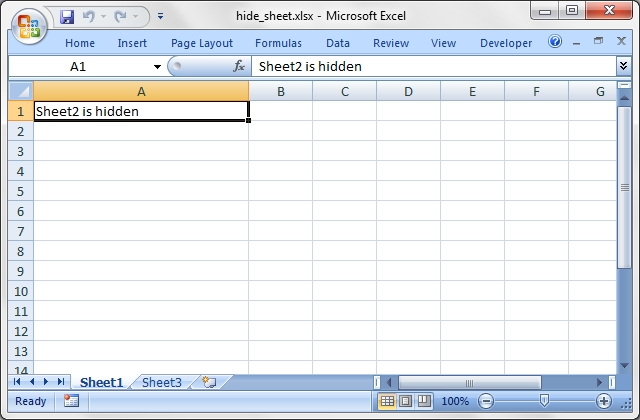Source code for this example:

``````    #!/usr/bin/perl

#######################################################################
#
# Example of how to hide a worksheet with Excel::Writer::XLSX.
#
# Copyright 2000-2021, John McNamara, jmcnamara@cpan.org
#

use strict;
use warnings;
use Excel::Writer::XLSX;

my \$workbook   = Excel::Writer::XLSX->new( 'hide_sheet.xlsx' );
my \$worksheet1 = \$workbook->add_worksheet();
my \$worksheet2 = \$workbook->add_worksheet();
my \$worksheet3 = \$workbook->add_worksheet();

\$worksheet1->set_column( 'A:A', 30 );
\$worksheet2->set_column( 'A:A', 30 );
\$worksheet3->set_column( 'A:A', 30 );

# Sheet2 won't be visible until it is unhidden in Excel.
\$worksheet2->hide();

\$worksheet1->write( 0, 0, 'Sheet2 is hidden' );
\$worksheet2->write( 0, 0, "Now it's my turn to find you." );
\$worksheet3->write( 0, 0, 'Sheet2 is hidden' );

\$workbook->close();

__END__``````

Example of how to use the Excel::Writer::XLSX module to write hyperlinks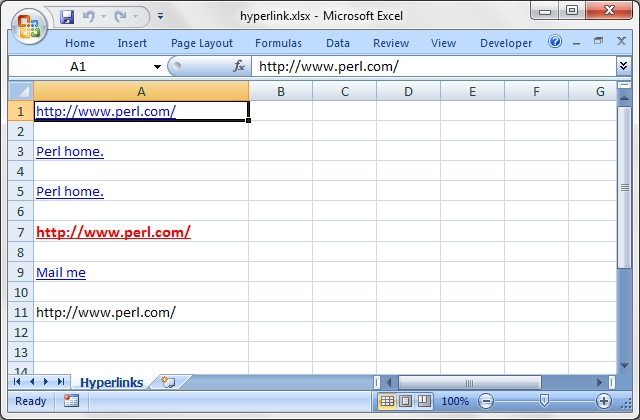Source code for this example:

``````    #!/usr/bin/perl

###############################################################################
#
# Example of how to use the Excel::Writer::XLSX module to write hyperlinks
#
#
# Copyright 2000-2021, John McNamara, jmcnamara@cpan.org
#

use strict;
use warnings;
use Excel::Writer::XLSX;

# Create a new workbook and add a worksheet
my \$workbook = Excel::Writer::XLSX->new( 'hyperlink.xlsx' );

# Format the first column
\$worksheet->set_column( 'A:A', 30 );
\$worksheet->set_selection( 'B1' );

# Add a user defined hyperlink format.
my \$red_format = \$workbook->add_format(
color     => 'red',
bold      => 1,
underline => 1,
size      => 12,
);

# Add an alternate description string to the URL.
my \$str = 'Perl home.';

# Add a "tool tip" to the URL.
my \$tip = 'Get the latest Perl news here.';

# Write some hyperlinks. Unspecified or undefined format paraamters will be
# replace with the defuault Excel hyperlink style.
\$worksheet->write( 'A1', 'http://www.perl.com/' );
\$worksheet->write( 'A3', 'http://www.perl.com/', undef, \$str );
\$worksheet->write( 'A5', 'http://www.perl.com/', undef, \$str, \$tip );
\$worksheet->write( 'A7', 'http://www.perl.com/', \$red_format );
\$worksheet->write( 'A9', 'mailto:jmcnamara@cpan.org', undef, 'Mail me' );

# Write a URL that isn't a hyperlink
\$worksheet->write_string( 'A11', 'http://www.perl.com/' );

\$workbook->close();

__END__``````

Example of how to use the Excel::Writer::XLSX module to write internal and external hyperlinks.

If you wish to run this program and follow the hyperlinks you should create the following directory structure:

C:\ -- Temp --+-- Europe | \-- Asia

``````    #!/usr/bin/perl

###############################################################################
#
# Example of how to use the Excel::Writer::XLSX module to write internal and
#
# If you wish to run this program and follow the hyperlinks you should create
# the following directory structure:
#
# C:\ -- Temp --+-- Europe
#               |
#               \-- Asia
#
#
#
# Copyright 2000-2021, John McNamara, jmcnamara@cpan.org
#

use strict;
use warnings;
use Excel::Writer::XLSX;

# Create three workbooks:
#   C:\Temp\Europe\Ireland.xlsx
#   C:\Temp\Europe\Italy.xlsx
#   C:\Temp\Asia\China.xlsx
#

my \$ireland = Excel::Writer::XLSX->new( 'C:\Temp\Europe\Ireland.xlsx' );

my \$ire_sales      = \$ireland->add_worksheet( 'Sales' );
my \$ire_data       = \$ireland->add_worksheet( 'Product Data' );
my \$ire_url_format = \$ireland->get_default_url_format();

my \$italy = Excel::Writer::XLSX->new( 'C:\Temp\Europe\Italy.xlsx' );

my \$ita_sales      = \$italy->add_worksheet( 'Sales' );
my \$ita_data       = \$italy->add_worksheet( 'Product Data' );
my \$ita_url_format = \$italy->get_default_url_format();

my \$china = Excel::Writer::XLSX->new( 'C:\Temp\Asia\China.xlsx' );

my \$cha_sales      = \$china->add_worksheet( 'Sales' );
my \$cha_data       = \$china->add_worksheet( 'Product Data' );
my \$cha_url_format = \$china->get_default_url_format();

# Add an alternative format
my \$format = \$ireland->add_format( color => 'green', bold => 1 );
\$ire_links->set_column( 'A:B', 25 );

###############################################################################
#
# Examples of internal links
#

\$ire_links->write_url( 'A2', 'internal:Sales!A2', \$ire_url_format );

# Internal link to a range
\$ire_links->write_url( 'A3', 'internal:Sales!A3:D3', \$ire_url_format );

# Internal link with an alternative string

# Internal link with an alternative format
\$ire_links->write_url( 'A5', 'internal:Sales!A5', \$format );

# Internal link with an alternative string and format

# Internal link (spaces in worksheet name)
\$ire_links->write_url( 'A7', q{internal:'Product Data'!A7}, \$ire_url_format );

###############################################################################
#
# Examples of external links
#

# External link to a local file
\$ire_links->write_url( 'B2', 'external:Italy.xlsx', \$ire_url_format );

# External link to a local file with worksheet
\$ire_links->write_url( 'B3', 'external:Italy.xlsx#Sales!B3', \$ire_url_format );

# External link to a local file with worksheet and alternative string

# External link to a local file with worksheet and format
\$ire_links->write_url( 'B5', 'external:Italy.xlsx#Sales!B5', \$format );

# External link to a remote file, absolute path
\$ire_links->write_url( 'B6', 'external:C:/Temp/Asia/China.xlsx', \$ire_url_format );

# External link to a remote file, relative path
\$ire_links->write_url( 'B7', 'external:../Asia/China.xlsx', \$ire_url_format );

# External link to a remote file with worksheet
\$ire_links->write_url( 'B8', 'external:C:/Temp/Asia/China.xlsx#Sales!B8', \$ire_url_format );

# External link to a remote file with worksheet (with spaces in the name)
\$ire_links->write_url( 'B9', q{external:C:/Temp/Asia/China.xlsx#'Product Data'!B9}, \$ire_url_format );

###############################################################################
#
# Some utility links to return to the main sheet
#
\$ire_sales->write_url( 'A2', 'internal:Links!A2', \$ire_url_format, 'Back' );
\$ire_sales->write_url( 'A3', 'internal:Links!A3', \$ire_url_format, 'Back' );
\$ire_sales->write_url( 'A4', 'internal:Links!A4', \$ire_url_format, 'Back' );
\$ire_sales->write_url( 'A5', 'internal:Links!A5', \$ire_url_format, 'Back' );
\$ire_sales->write_url( 'A6', 'internal:Links!A6', \$ire_url_format, 'Back' );
\$ire_data->write_url ( 'A7', 'internal:Links!A7', \$ire_url_format, 'Back' );

\$ita_sales->write_url( 'B3', 'external:Ireland.xlsx#Links!B3', \$ita_url_format, 'Back' );
\$ita_sales->write_url( 'B4', 'external:Ireland.xlsx#Links!B4', \$ita_url_format, 'Back' );
\$ita_sales->write_url( 'B5', 'external:Ireland.xlsx#Links!B5', \$ita_url_format, 'Back' );
\$cha_sales->write_url( 'B8', 'external:C:/Temp/Europe/Ireland.xlsx#Links!B8', \$cha_url_format, 'Back' );
\$cha_data->write_url ( 'B9', 'external:C:/Temp/Europe/Ireland.xlsx#Links!B9', \$cha_url_format, 'Back' );

\$ireland->close();
\$italy->close();
\$china->close();

__END__``````

## Example: indent.pl

A simple formatting example using Excel::Writer::XLSX.

This program demonstrates the indentation cell format.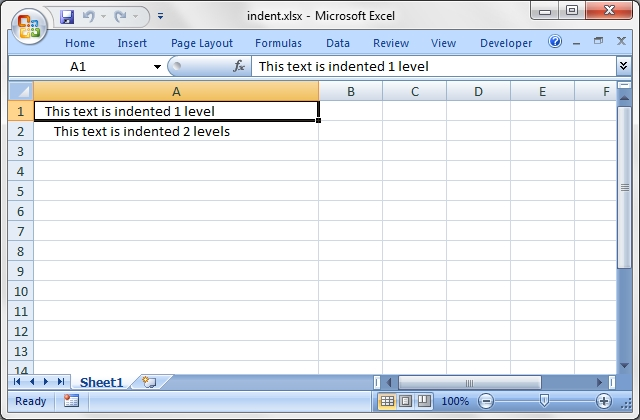Source code for this example:

``````    #!/usr/bin/perl -w

##############################################################################
#
# A simple formatting example using Excel::Writer::XLSX.
#
# This program demonstrates the indentation cell format.
#
# Copyright 2000-2021, John McNamara, jmcnamara@cpan.org
#

use strict;
use Excel::Writer::XLSX;

my \$workbook = Excel::Writer::XLSX->new( 'indent.xlsx' );

my \$worksheet = \$workbook->add_worksheet();
my \$indent1   = \$workbook->add_format( indent => 1 );
my \$indent2   = \$workbook->add_format( indent => 2 );

\$worksheet->set_column( 'A:A', 40 );

\$worksheet->write( 'A1', "This text is indented 1 level",  \$indent1 );
\$worksheet->write( 'A2', "This text is indented 2 levels", \$indent2 );

\$workbook->close();

__END__``````

## Example: ignore_errors.pl

An example of turning off worksheet cells errors/warnings using the Excel::Writer::XLSX module.

``````    #!/usr/bin/perl -w

#######################################################################
#
# An example of turning off worksheet cells errors/warnings using the
# Excel::Writer::XLSX module.
#
# Copyright 2000-2021, John McNamara, jmcnamara@cpan.org
#

use strict;
use Excel::Writer::XLSX;

my \$workbook  = Excel::Writer::XLSX->new( 'ignore_errors.xlsx' );
my \$worksheet = \$workbook->add_worksheet();

# Write strings that looks like numbers. This will cause an Excel warning.
\$worksheet->write_string('C2', '123');
\$worksheet->write_string('C3', '123');

# Write a divide by zero formula. This will also cause an Excel warning.
\$worksheet->write_formula('C5', '=1/0');
\$worksheet->write_formula('C6', '=1/0');

# Turn off some of the warnings:
\$worksheet->ignore_errors({number_stored_as_text => 'C3', eval_error => 'C6'});

# Write some descriptions for the cells and make the column wider for clarity.
\$worksheet->set_column('B:B', 16);
\$worksheet->write('B2', 'Warning:');
\$worksheet->write('B3', 'Warning turned off:');
\$worksheet->write('B5', 'Warning:');
\$worksheet->write('B6', 'Warning turned off:');

\$workbook->close();

__END__``````

## Example: macros.pl

An example of adding macros to an Excel::Writer::XLSX file using a VBA project file extracted from an existing Excel xlsm file.

The `extract_vba` utility supplied with Excel::Writer::XLSX can be used to extract the vbaProject.bin file.

An embedded macro is connected to a form button on the worksheet.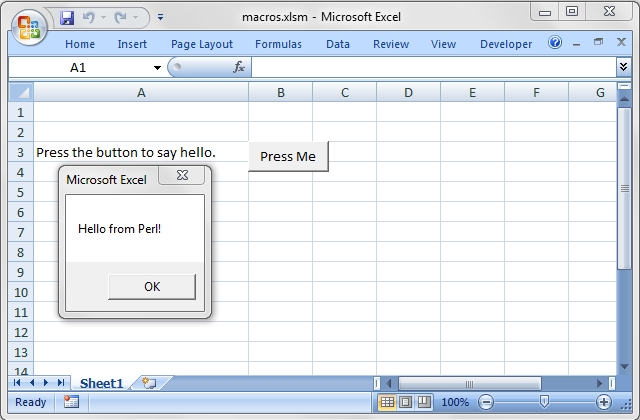Source code for this example:

``````    #!/usr/bin/perl

#######################################################################
#
# An example of adding macros to an Excel::Writer::XLSX file using
# a VBA project file extracted from an existing Excel xlsm file.
#
# The C<extract_vba> utility supplied with Excel::Writer::XLSX can be
# used to extract the vbaProject.bin file.
#
# An embedded macro is connected to a form button on the worksheet.
#
# Copyright 2000-2021, John McNamara, jmcnamara@cpan.org
#

use strict;
use warnings;
use Excel::Writer::XLSX;

# Note the file extension should be .xlsm.
my \$workbook  = Excel::Writer::XLSX->new( 'macros.xlsm' );
my \$worksheet = \$workbook->add_worksheet();

\$worksheet->set_column( 'A:A', 30 );

# Add the VBA project binary.

# Show text for the end user.
\$worksheet->write( 'A3', 'Press the button to say hello.' );

# Add a button tied to a macro in the VBA project.
\$worksheet->insert_button(
'B3',
{
macro   => 'say_hello',
caption => 'Press Me',
width   => 80,
height  => 30
}
);

\$workbook->close();

__END__``````

## Example: merge1.pl

Simple example of merging cells using the Excel::Writer::XLSX module.

This example merges three cells using the "Centre Across Selection" alignment which was the Excel 5 method of achieving a merge. For a more modern approach use the merge_range() worksheet method instead. See the merge3.pl - merge6.pl programs.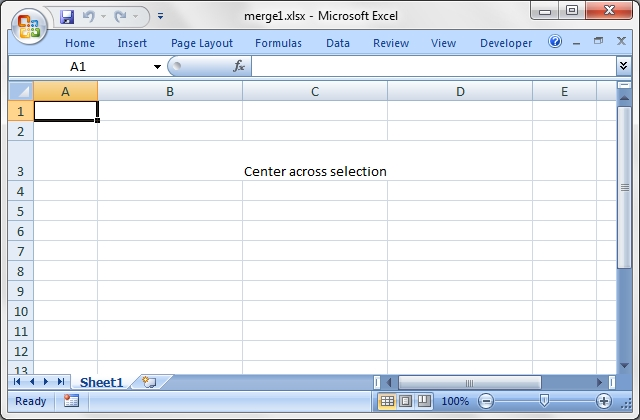Source code for this example:

``````    #!/usr/bin/perl

###############################################################################
#
# Simple example of merging cells using the Excel::Writer::XLSX module.
#
# This example merges three cells using the "Centre Across Selection"
# alignment which was the Excel 5 method of achieving a merge. For a more
# modern approach use the merge_range() worksheet method instead.
# See the merge3.pl - merge6.pl programs.
#
# Copyright 2000-2021, John McNamara, jmcnamara@cpan.org
#

use strict;
use warnings;
use Excel::Writer::XLSX;

# Create a new workbook and add a worksheet
my \$workbook  = Excel::Writer::XLSX->new( 'merge1.xlsx' );
my \$worksheet = \$workbook->add_worksheet();

# Increase the cell size of the merged cells to highlight the formatting.
\$worksheet->set_column( 'B:D', 20 );
\$worksheet->set_row( 2, 30 );

# Create a merge format
my \$format = \$workbook->add_format( center_across => 1 );

# Only one cell should contain text, the others should be blank.
\$worksheet->write( 2, 1, "Center across selection", \$format );
\$worksheet->write_blank( 2, 2, \$format );
\$worksheet->write_blank( 2, 3, \$format );

\$workbook->close();

__END__``````

## Example: merge2.pl

Simple example of merging cells using the Excel::Writer::XLSX module

This example merges three cells using the "Centre Across Selection" alignment which was the Excel 5 method of achieving a merge. For a more modern approach use the merge_range() worksheet method instead. See the merge3.pl - merge6.pl programs.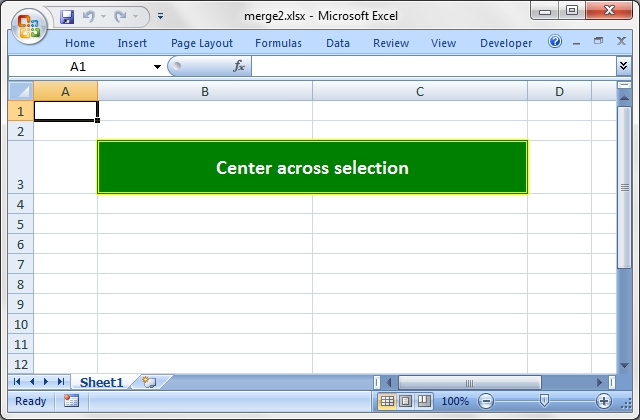Source code for this example:

``````    #!/usr/bin/perl

###############################################################################
#
# Simple example of merging cells using the Excel::Writer::XLSX module
#
# This example merges three cells using the "Centre Across Selection"
# alignment which was the Excel 5 method of achieving a merge. For a more
# modern approach use the merge_range() worksheet method instead.
# See the merge3.pl - merge6.pl programs.
#
# Copyright 2000-2021, John McNamara, jmcnamara@cpan.org
#

use strict;
use warnings;
use Excel::Writer::XLSX;

# Create a new workbook and add a worksheet
my \$workbook  = Excel::Writer::XLSX->new( 'merge2.xlsx' );
my \$worksheet = \$workbook->add_worksheet();

# Increase the cell size of the merged cells to highlight the formatting.
\$worksheet->set_column( 1, 2, 30 );
\$worksheet->set_row( 2, 40 );

# Create a merged format
my \$format = \$workbook->add_format(
center_across => 1,
bold          => 1,
size          => 15,
pattern       => 1,
border        => 6,
color         => 'white',
fg_color      => 'green',
border_color  => 'yellow',
align         => 'vcenter',
);

# Only one cell should contain text, the others should be blank.
\$worksheet->write( 2, 1, "Center across selection", \$format );
\$worksheet->write_blank( 2, 2, \$format );

\$workbook->close();

__END__``````

## Example: merge3.pl

Example of how to use Excel::Writer::XLSX to write a hyperlink in a merged cell.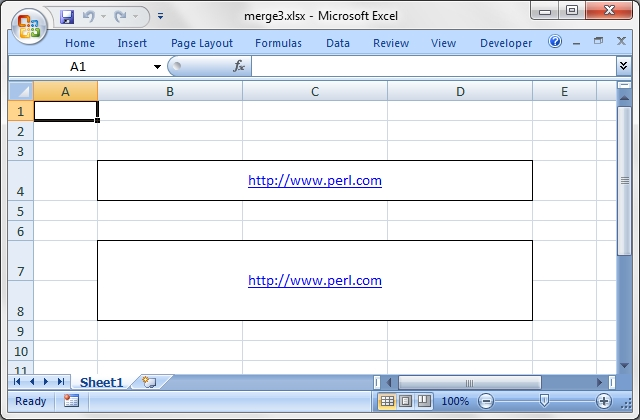Source code for this example:

``````    #!/usr/bin/perl

###############################################################################
#
# Example of how to use Excel::Writer::XLSX to write a hyperlink in a
# merged cell.
#
# Copyright 2000-2021, John McNamara, jmcnamara@cpan.org
#

use strict;
use warnings;
use Excel::Writer::XLSX;

# Create a new workbook and add a worksheet
my \$workbook  = Excel::Writer::XLSX->new( 'merge3.xlsx' );
my \$worksheet = \$workbook->add_worksheet();

# Increase the cell size of the merged cells to highlight the formatting.
\$worksheet->set_row( \$_, 30 ) for ( 3, 6, 7 );
\$worksheet->set_column( 'B:D', 20 );

###############################################################################
#
# Example: Merge cells containing a hyperlink using merge_range().
#
my \$format = \$workbook->add_format(
border    => 1,
underline => 1,
color     => 'blue',
align     => 'center',
valign    => 'vcenter',
);

# Merge 3 cells
\$worksheet->merge_range( 'B4:D4', 'http://www.perl.com', \$format );

# Merge 3 cells over two rows
\$worksheet->merge_range( 'B7:D8', 'http://www.perl.com', \$format );

\$workbook->close();

__END__``````

## Example: merge4.pl

Example of how to use the Excel::Writer::XLSX merge_range() workbook method with complex formatting.Source code for this example:

``````    #!/usr/bin/perl

###############################################################################
#
# Example of how to use the Excel::Writer::XLSX merge_range() workbook
# method with complex formatting.
#
# Copyright 2000-2021, John McNamara, jmcnamara@cpan.org
#

use strict;
use warnings;
use Excel::Writer::XLSX;

# Create a new workbook and add a worksheet
my \$workbook  = Excel::Writer::XLSX->new( 'merge4.xlsx' );
my \$worksheet = \$workbook->add_worksheet();

# Increase the cell size of the merged cells to highlight the formatting.
\$worksheet->set_row( \$_, 30 ) for ( 1 .. 11 );
\$worksheet->set_column( 'B:D', 20 );

###############################################################################
#
# Example 1: Text centered vertically and horizontally
#
my \$format1 = \$workbook->add_format(
border => 6,
bold   => 1,
color  => 'red',
valign => 'vcenter',
align  => 'center',
);

\$worksheet->merge_range( 'B2:D3', 'Vertical and horizontal', \$format1 );

###############################################################################
#
# Example 2: Text aligned to the top and left
#
my \$format2 = \$workbook->add_format(
border => 6,
bold   => 1,
color  => 'red',
valign => 'top',
align  => 'left',
);

\$worksheet->merge_range( 'B5:D6', 'Aligned to the top and left', \$format2 );

###############################################################################
#
# Example 3:  Text aligned to the bottom and right
#
my \$format3 = \$workbook->add_format(
border => 6,
bold   => 1,
color  => 'red',
valign => 'bottom',
align  => 'right',
);

\$worksheet->merge_range( 'B8:D9', 'Aligned to the bottom and right', \$format3 );

###############################################################################
#
# Example 4:  Text justified (i.e. wrapped) in the cell
#
my \$format4 = \$workbook->add_format(
border => 6,
bold   => 1,
color  => 'red',
valign => 'top',
align  => 'justify',
);

\$worksheet->merge_range( 'B11:D12', 'Justified: ' . 'so on and ' x 18,
\$format4 );

\$workbook->close();

__END__``````

## Example: merge5.pl

Example of how to use the Excel::Writer::XLSX merge_cells() workbook method with complex formatting and rotation.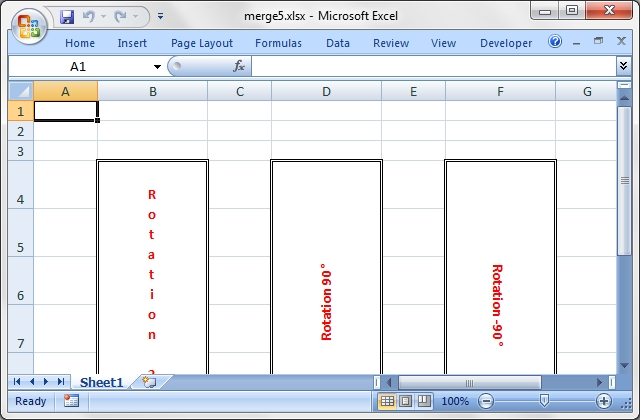Source code for this example:

``````    #!/usr/bin/perl

###############################################################################
#
# Example of how to use the Excel::Writer::XLSX merge_cells() workbook
# method with complex formatting and rotation.
#
#
# Copyright 2000-2021, John McNamara, jmcnamara@cpan.org
#

use strict;
use warnings;
use Excel::Writer::XLSX;

# Create a new workbook and add a worksheet
my \$workbook  = Excel::Writer::XLSX->new( 'merge5.xlsx' );
my \$worksheet = \$workbook->add_worksheet();

# Increase the cell size of the merged cells to highlight the formatting.
\$worksheet->set_row( \$_, 36 ) for ( 3 .. 8 );
\$worksheet->set_column( \$_, \$_, 15 ) for ( 1, 3, 5 );

###############################################################################
#
# Rotation 1, letters run from top to bottom
#
my \$format1 = \$workbook->add_format(
border   => 6,
bold     => 1,
color    => 'red',
valign   => 'vcentre',
align    => 'centre',
rotation => 270,
);

\$worksheet->merge_range( 'B4:B9', 'Rotation 270', \$format1 );

###############################################################################
#
# Rotation 2, 90° anticlockwise
#
my \$format2 = \$workbook->add_format(
border   => 6,
bold     => 1,
color    => 'red',
valign   => 'vcentre',
align    => 'centre',
rotation => 90,
);

\$worksheet->merge_range( 'D4:D9', 'Rotation 90°', \$format2 );

###############################################################################
#
# Rotation 3, 90° clockwise
#
my \$format3 = \$workbook->add_format(
border   => 6,
bold     => 1,
color    => 'red',
valign   => 'vcentre',
align    => 'centre',
rotation => -90,
);

\$worksheet->merge_range( 'F4:F9', 'Rotation -90°', \$format3 );

\$workbook->close();

__END__``````

## Example: merge6.pl

Example of how to use the Excel::Writer::XLSX merge_cells() workbook method with Unicode strings.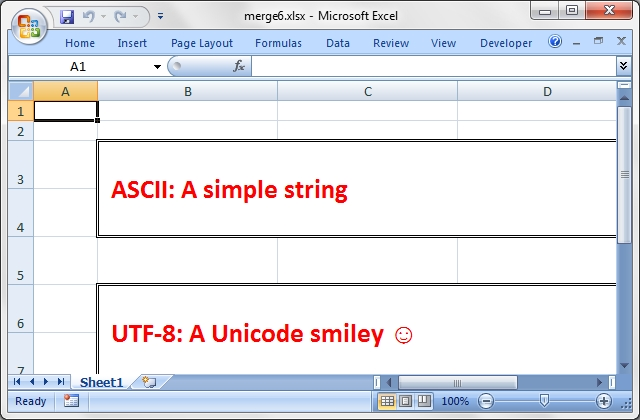Source code for this example:

``````    #!/usr/bin/perl

###############################################################################
#
# Example of how to use the Excel::Writer::XLSX merge_cells() workbook
# method with Unicode strings.
#
#
# Copyright 2000-2021, John McNamara, jmcnamara@cpan.org
#

use strict;
use warnings;
use Excel::Writer::XLSX;

# Create a new workbook and add a worksheet
my \$workbook  = Excel::Writer::XLSX->new( 'merge6.xlsx' );
my \$worksheet = \$workbook->add_worksheet();

# Increase the cell size of the merged cells to highlight the formatting.
\$worksheet->set_row( \$_, 36 ) for 2 .. 9;
\$worksheet->set_column( 'B:D', 25 );

# Format for the merged cells.
my \$format = \$workbook->add_format(
border => 6,
bold   => 1,
color  => 'red',
size   => 20,
valign => 'vcentre',
align  => 'left',
indent => 1,
);

###############################################################################
#
# Write an Ascii string.
#
\$worksheet->merge_range( 'B3:D4', 'ASCII: A simple string', \$format );

###############################################################################
#
# Write a UTF-8 Unicode string.
#
my \$smiley = chr 0x263a;
\$worksheet->merge_range( 'B6:D7', "UTF-8: A Unicode smiley \$smiley", \$format );

\$workbook->close();

__END__``````

## Example: mod_perl1.pl

Example of how to use the Excel::Writer::XLSX module to send an Excel file to a browser using mod_perl 1 and Apache

This module ties *XLSX directly to Apache, and with the correct content-disposition/types it will prompt the user to save the file, or open it at this location.

This script is a modification of the Excel::Writer::XLSX cgi.pl example.

Change the name of this file to Cgi.pm. Change the package location to wherever you locate this package. In the example below it is located in the Excel::Writer::XLSX directory.

Your httpd.conf entry for this module, should you choose to use it as a stand alone app, should look similar to the following:

``````    <Location /spreadsheet-test>
SetHandler perl-script
PerlHandler Excel::Writer::XLSX::Cgi
</Location>``````

The PerlHandler name above and the package name below *have* to match.

``````    ###############################################################################
#
# Example of how to use the Excel::Writer::XLSX module to send an Excel
# file to a browser using mod_perl 1 and Apache
#
# This module ties *XLSX directly to Apache, and with the correct
# content-disposition/types it will prompt the user to save
# the file, or open it at this location.
#
# This script is a modification of the Excel::Writer::XLSX cgi.pl example.
#
# Change the name of this file to Cgi.pm.
# Change the package location to wherever you locate this package.
# In the example below it is located in the Excel::Writer::XLSX directory.
#
# Your httpd.conf entry for this module, should you choose to use it
# as a stand alone app, should look similar to the following:
#
#       SetHandler perl-script
#       PerlHandler Excel::Writer::XLSX::Cgi
#     </Location>
#
# The PerlHandler name above and the package name below *have* to match.

# Apr 2001, Thomas Sullivan, webmaster@860.org
# Feb 2001, John McNamara, jmcnamara@cpan.org

package Excel::Writer::XLSX::Cgi;

##########################################
# Pragma Definitions
##########################################
use strict;

##########################################
# Required Modules
##########################################
use Apache::Constants qw(:common);
use Apache::Request;
use Apache::URI;    # This may not be needed
use Excel::Writer::XLSX;

##########################################
# Main App Body
##########################################
sub handler {

# New apache object
# Should you decide to use it.
my \$r = Apache::Request->new( shift );

# Set the filename and send the content type
# This will appear when they save the spreadsheet
my \$filename = "cgitest.xlsx";

####################################################
## Send the content type headers
####################################################
print "Content-disposition: attachment;filename=\$filename\n";

####################################################
# Tie a filehandle to Apache's STDOUT.
# Create a new workbook and add a worksheet.
####################################################
tie *XLSX => 'Apache';
binmode( *XLSX );

my \$workbook  = Excel::Writer::XLSX->new( \*XLSX );
my \$worksheet = \$workbook->add_worksheet();

# Set the column width for column 1
\$worksheet->set_column( 0, 0, 20 );

# Create a format
my \$format = \$workbook->add_format();
\$format->set_bold();
\$format->set_size( 15 );
\$format->set_color( 'blue' );

# Write to the workbook
\$worksheet->write( 0, 0, "Hi Excel!", \$format );

# You must close the workbook for Content-disposition
\$workbook->close();
}

1;``````

## Example: mod_perl2.pl

Example of how to use the Excel::Writer::XLSX module to send an Excel file to a browser using mod_perl 2 and Apache.

This module ties *XLSX directly to Apache, and with the correct content-disposition/types it will prompt the user to save the file, or open it at this location.

This script is a modification of the Excel::Writer::XLSX cgi.pl example.

Change the name of this file to MP2Test.pm. Change the package location to wherever you locate this package. In the example below it is located in the Excel::Writer::XLSX directory.

Your httpd.conf entry for this module, should you choose to use it as a stand alone app, should look similar to the following:

``````    PerlModule Apache2::RequestRec
PerlModule APR::Table
PerlModule Apache2::RequestIO

SetHandler perl-script
PerlResponseHandler Excel::Writer::XLSX::MP2Test
</Location>``````

The PerlResponseHandler must match the package name below.

``````    ###############################################################################
#
# Example of how to use the Excel::Writer::XLSX module to send an Excel
# file to a browser using mod_perl 2 and Apache.
#
# This module ties *XLSX directly to Apache, and with the correct
# content-disposition/types it will prompt the user to save
# the file, or open it at this location.
#
# This script is a modification of the Excel::Writer::XLSX cgi.pl example.
#
# Change the name of this file to MP2Test.pm.
# Change the package location to wherever you locate this package.
# In the example below it is located in the Excel::Writer::XLSX directory.
#
# Your httpd.conf entry for this module, should you choose to use it
# as a stand alone app, should look similar to the following:
#
#     PerlModule Apache2::RequestRec
#     PerlModule APR::Table
#     PerlModule Apache2::RequestIO
#
#        SetHandler perl-script
#        PerlResponseHandler Excel::Writer::XLSX::MP2Test
#     </Location>
#
# The PerlResponseHandler must match the package name below.

# Jun 2004, Matisse Enzer, matisse@matisse.net  (mod_perl 2 version)
# Apr 2001, Thomas Sullivan, webmaster@860.org
# Feb 2001, John McNamara, jmcnamara@cpan.org

package Excel::Writer::XLSX::MP2Test;

##########################################
# Pragma Definitions
##########################################
use strict;

##########################################
# Required Modules
##########################################
use Apache2::Const -compile => qw( :common );
use Excel::Writer::XLSX;

##########################################
# Main App Body
##########################################
sub handler {
my ( \$r ) = @_;   # Apache request object is passed to handler in mod_perl 2

# Set the filename and send the content type
# This will appear when they save the spreadsheet
my \$filename = "mod_perl2_test.xlsx";

####################################################
## Send the content type headers the mod_perl 2 way
####################################################

####################################################
# Tie a filehandle to Apache's STDOUT.
# Create a new workbook and add a worksheet.
####################################################
tie *XLSX => \$r;  # The mod_perl 2 way. Tie to the Apache::RequestRec object
binmode( *XLSX );

my \$workbook  = Excel::Writer::XLSX->new( \*XLSX );
my \$worksheet = \$workbook->add_worksheet();

# Set the column width for column 1
\$worksheet->set_column( 0, 0, 20 );

# Create a format
my \$format = \$workbook->add_format();
\$format->set_bold();
\$format->set_size( 15 );
\$format->set_color( 'blue' );

# Write to the workbook
\$worksheet->write( 0, 0, 'Hi Excel! from ' . \$r->hostname, \$format );

# You must close the workbook for Content-disposition
\$workbook->close();
return Apache2::Const::OK;
}

1;``````

## Example: outline.pl

Example of how use Excel::Writer::XLSX to generate Excel outlines and grouping.

Excel allows you to group rows or columns so that they can be hidden or displayed with a single mouse click. This feature is referred to as outlines.

Outlines can reduce complex data down to a few salient sub-totals or summaries.

This feature is best viewed in Excel but the following is an ASCII representation of what a worksheet with three outlines might look like. Rows 3-4 and rows 7-8 are grouped at level 2. Rows 2-9 are grouped at level 1. The lines at the left hand side are called outline level bars.

``````            ------------------------------------------
1 2 3 |   |   A   |   B   |   C   |   D   |  ...
------------------------------------------
_    | 1 |   A   |       |       |       |  ...
|  _  | 2 |   B   |       |       |       |  ...
| |   | 3 |  (C)  |       |       |       |  ...
| |   | 4 |  (D)  |       |       |       |  ...
| -   | 5 |   E   |       |       |       |  ...
|  _  | 6 |   F   |       |       |       |  ...
| |   | 7 |  (G)  |       |       |       |  ...
| |   | 8 |  (H)  |       |       |       |  ...
| -   | 9 |   I   |       |       |       |  ...
-     | . |  ...  |  ...  |  ...  |  ...  |  ...``````

Clicking the minus sign on each of the level 2 outlines will collapse and hide the data as shown in the next figure. The minus sign changes to a plus sign to indicate that the data in the outline is hidden.

``````            ------------------------------------------
1 2 3 |   |   A   |   B   |   C   |   D   |  ...
------------------------------------------
_    | 1 |   A   |       |       |       |  ...
|     | 2 |   B   |       |       |       |  ...
| +   | 5 |   E   |       |       |       |  ...
|     | 6 |   F   |       |       |       |  ...
| +   | 9 |   I   |       |       |       |  ...
-     | . |  ...  |  ...  |  ...  |  ...  |  ...``````

Clicking on the minus sign on the level 1 outline will collapse the remaining rows as follows:

``````            ------------------------------------------
1 2 3 |   |   A   |   B   |   C   |   D   |  ...
------------------------------------------
| 1 |   A   |       |       |       |  ...
+     | . |  ...  |  ...  |  ...  |  ...  |  ...``````

See the main Excel::Writer::XLSX documentation for more information.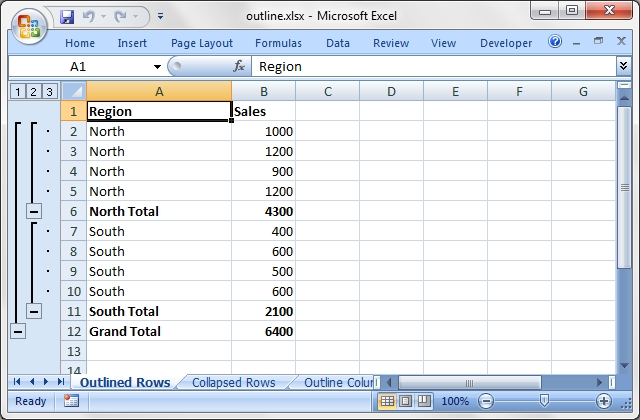Source code for this example:

``````    #!/usr/bin/perl

###############################################################################
#
# Example of how use Excel::Writer::XLSX to generate Excel outlines and
# grouping.
#
#
# Excel allows you to group rows or columns so that they can be hidden or
# displayed with a single mouse click. This feature is referred to as outlines.
#
# Outlines can reduce complex data down to a few salient sub-totals or
# summaries.
#
# This feature is best viewed in Excel but the following is an ASCII
# representation of what a worksheet with three outlines might look like.
# Rows 3-4 and rows 7-8 are grouped at level 2. Rows 2-9 are grouped at
# level 1. The lines at the left hand side are called outline level bars.
#
#
#             ------------------------------------------
#      1 2 3 |   |   A   |   B   |   C   |   D   |  ...
#             ------------------------------------------
#       _    | 1 |   A   |       |       |       |  ...
#      |  _  | 2 |   B   |       |       |       |  ...
#      | |   | 3 |  (C)  |       |       |       |  ...
#      | |   | 4 |  (D)  |       |       |       |  ...
#      | -   | 5 |   E   |       |       |       |  ...
#      |  _  | 6 |   F   |       |       |       |  ...
#      | |   | 7 |  (G)  |       |       |       |  ...
#      | |   | 8 |  (H)  |       |       |       |  ...
#      | -   | 9 |   I   |       |       |       |  ...
#      -     | . |  ...  |  ...  |  ...  |  ...  |  ...
#
#
# Clicking the minus sign on each of the level 2 outlines will collapse and
# hide the data as shown in the next figure. The minus sign changes to a plus
# sign to indicate that the data in the outline is hidden.
#
#             ------------------------------------------
#      1 2 3 |   |   A   |   B   |   C   |   D   |  ...
#             ------------------------------------------
#       _    | 1 |   A   |       |       |       |  ...
#      |     | 2 |   B   |       |       |       |  ...
#      | +   | 5 |   E   |       |       |       |  ...
#      |     | 6 |   F   |       |       |       |  ...
#      | +   | 9 |   I   |       |       |       |  ...
#      -     | . |  ...  |  ...  |  ...  |  ...  |  ...
#
#
# Clicking on the minus sign on the level 1 outline will collapse the remaining
# rows as follows:
#
#             ------------------------------------------
#      1 2 3 |   |   A   |   B   |   C   |   D   |  ...
#             ------------------------------------------
#            | 1 |   A   |       |       |       |  ...
#      +     | . |  ...  |  ...  |  ...  |  ...  |  ...
#
# See the main Excel::Writer::XLSX documentation for more information.
#
# Copyright 2000-2021, John McNamara, jmcnamara@cpan.org
#

use strict;
use warnings;
use Excel::Writer::XLSX;

# Create a new workbook and add some worksheets
my \$workbook   = Excel::Writer::XLSX->new( 'outline.xlsx' );
my \$worksheet1 = \$workbook->add_worksheet( 'Outlined Rows' );
my \$worksheet2 = \$workbook->add_worksheet( 'Collapsed Rows' );
my \$worksheet3 = \$workbook->add_worksheet( 'Outline Columns' );
my \$worksheet4 = \$workbook->add_worksheet( 'Outline levels' );

# Add a general format
my \$bold = \$workbook->add_format( bold => 1 );

###############################################################################
#
# Example 1: Create a worksheet with outlined rows. It also includes SUBTOTAL()
# functions so that it looks like the type of automatic outlines that are
# generated when you use the Excel Data->SubTotals menu item.
#

# For outlines the important parameters are \$hidden and \$level. Rows with the
# same \$level are grouped together. The group will be collapsed if \$hidden is
# non-zero. \$height and \$XF are assigned default values if they are undef.
#
# The syntax is: set_row(\$row, \$height, \$XF, \$hidden, \$level, \$collapsed)
#
\$worksheet1->set_row( 1, undef, undef, 0, 2 );
\$worksheet1->set_row( 2, undef, undef, 0, 2 );
\$worksheet1->set_row( 3, undef, undef, 0, 2 );
\$worksheet1->set_row( 4, undef, undef, 0, 2 );
\$worksheet1->set_row( 5, undef, undef, 0, 1 );

\$worksheet1->set_row( 6,  undef, undef, 0, 2 );
\$worksheet1->set_row( 7,  undef, undef, 0, 2 );
\$worksheet1->set_row( 8,  undef, undef, 0, 2 );
\$worksheet1->set_row( 9,  undef, undef, 0, 2 );
\$worksheet1->set_row( 10, undef, undef, 0, 1 );

# Add a column format for clarity
\$worksheet1->set_column( 'A:A', 20 );

# Add the data, labels and formulas
\$worksheet1->write( 'A1', 'Region', \$bold );
\$worksheet1->write( 'A2', 'North' );
\$worksheet1->write( 'A3', 'North' );
\$worksheet1->write( 'A4', 'North' );
\$worksheet1->write( 'A5', 'North' );
\$worksheet1->write( 'A6', 'North Total', \$bold );

\$worksheet1->write( 'B1', 'Sales', \$bold );
\$worksheet1->write( 'B2', 1000 );
\$worksheet1->write( 'B3', 1200 );
\$worksheet1->write( 'B4', 900 );
\$worksheet1->write( 'B5', 1200 );
\$worksheet1->write( 'B6', '=SUBTOTAL(9,B2:B5)', \$bold );

\$worksheet1->write( 'A7',  'South' );
\$worksheet1->write( 'A8',  'South' );
\$worksheet1->write( 'A9',  'South' );
\$worksheet1->write( 'A10', 'South' );
\$worksheet1->write( 'A11', 'South Total', \$bold );

\$worksheet1->write( 'B7',  400 );
\$worksheet1->write( 'B8',  600 );
\$worksheet1->write( 'B9',  500 );
\$worksheet1->write( 'B10', 600 );
\$worksheet1->write( 'B11', '=SUBTOTAL(9,B7:B10)', \$bold );

\$worksheet1->write( 'A12', 'Grand Total',         \$bold );
\$worksheet1->write( 'B12', '=SUBTOTAL(9,B2:B10)', \$bold );

###############################################################################
#
# Example 2: Create a worksheet with outlined rows. This is the same as the
# previous example except that the rows are collapsed.
# Note: We need to indicate the row that contains the collapsed symbol '+'
# with the optional parameter, \$collapsed.

# The group will be collapsed if \$hidden is non-zero.
# The syntax is: set_row(\$row, \$height, \$XF, \$hidden, \$level, \$collapsed)
#
\$worksheet2->set_row( 1, undef, undef, 1, 2 );
\$worksheet2->set_row( 2, undef, undef, 1, 2 );
\$worksheet2->set_row( 3, undef, undef, 1, 2 );
\$worksheet2->set_row( 4, undef, undef, 1, 2 );
\$worksheet2->set_row( 5, undef, undef, 1, 1 );

\$worksheet2->set_row( 6,  undef, undef, 1, 2 );
\$worksheet2->set_row( 7,  undef, undef, 1, 2 );
\$worksheet2->set_row( 8,  undef, undef, 1, 2 );
\$worksheet2->set_row( 9,  undef, undef, 1, 2 );
\$worksheet2->set_row( 10, undef, undef, 1, 1 );
\$worksheet2->set_row( 11, undef, undef, 0, 0, 1 );

# Add a column format for clarity
\$worksheet2->set_column( 'A:A', 20 );

# Add the data, labels and formulas
\$worksheet2->write( 'A1', 'Region', \$bold );
\$worksheet2->write( 'A2', 'North' );
\$worksheet2->write( 'A3', 'North' );
\$worksheet2->write( 'A4', 'North' );
\$worksheet2->write( 'A5', 'North' );
\$worksheet2->write( 'A6', 'North Total', \$bold );

\$worksheet2->write( 'B1', 'Sales', \$bold );
\$worksheet2->write( 'B2', 1000 );
\$worksheet2->write( 'B3', 1200 );
\$worksheet2->write( 'B4', 900 );
\$worksheet2->write( 'B5', 1200 );
\$worksheet2->write( 'B6', '=SUBTOTAL(9,B2:B5)', \$bold );

\$worksheet2->write( 'A7',  'South' );
\$worksheet2->write( 'A8',  'South' );
\$worksheet2->write( 'A9',  'South' );
\$worksheet2->write( 'A10', 'South' );
\$worksheet2->write( 'A11', 'South Total', \$bold );

\$worksheet2->write( 'B7',  400 );
\$worksheet2->write( 'B8',  600 );
\$worksheet2->write( 'B9',  500 );
\$worksheet2->write( 'B10', 600 );
\$worksheet2->write( 'B11', '=SUBTOTAL(9,B7:B10)', \$bold );

\$worksheet2->write( 'A12', 'Grand Total',         \$bold );
\$worksheet2->write( 'B12', '=SUBTOTAL(9,B2:B10)', \$bold );

###############################################################################
#
# Example 3: Create a worksheet with outlined columns.
#
my \$data = [
[ 'Month', 'Jan', 'Feb', 'Mar', 'Apr', 'May', 'Jun', ' Total' ],
[ 'North', 50,    20,    15,    25,    65,    80,    '=SUM(B2:G2)' ],
[ 'South', 10,    20,    30,    50,    50,    50,    '=SUM(B3:G3)' ],
[ 'East',  45,    75,    50,    15,    75,    100,   '=SUM(B4:G4)' ],
[ 'West',  15,    15,    55,    35,    20,    50,    '=SUM(B5:G5)' ],
];

# Add bold format to the first row
\$worksheet3->set_row( 0, undef, \$bold );

# Syntax: set_column(\$col1, \$col2, \$width, \$XF, \$hidden, \$level, \$collapsed)
\$worksheet3->set_column( 'A:A', 10, \$bold );
\$worksheet3->set_column( 'B:G', 5, undef, 0, 1 );
\$worksheet3->set_column( 'H:H', 10 );

# Write the data and a formula
\$worksheet3->write_col( 'A1', \$data );
\$worksheet3->write( 'H6', '=SUM(H2:H5)', \$bold );

###############################################################################
#
# Example 4: Show all possible outline levels.
#
my \$levels = [
"Level 1", "Level 2", "Level 3", "Level 4", "Level 5", "Level 6",
"Level 7", "Level 6", "Level 5", "Level 4", "Level 3", "Level 2",
"Level 1"
];

\$worksheet4->write_col( 'A1', \$levels );

\$worksheet4->set_row( 0,  undef, undef, undef, 1 );
\$worksheet4->set_row( 1,  undef, undef, undef, 2 );
\$worksheet4->set_row( 2,  undef, undef, undef, 3 );
\$worksheet4->set_row( 3,  undef, undef, undef, 4 );
\$worksheet4->set_row( 4,  undef, undef, undef, 5 );
\$worksheet4->set_row( 5,  undef, undef, undef, 6 );
\$worksheet4->set_row( 6,  undef, undef, undef, 7 );
\$worksheet4->set_row( 7,  undef, undef, undef, 6 );
\$worksheet4->set_row( 8,  undef, undef, undef, 5 );
\$worksheet4->set_row( 9,  undef, undef, undef, 4 );
\$worksheet4->set_row( 10, undef, undef, undef, 3 );
\$worksheet4->set_row( 11, undef, undef, undef, 2 );
\$worksheet4->set_row( 12, undef, undef, undef, 1 );

\$workbook->close();

__END__``````

## Example: outline_collapsed.pl

Example of how to use Excel::Writer::XLSX to generate Excel outlines and grouping.

These examples focus mainly on collapsed outlines. See also the outlines.pl example program for more general examples.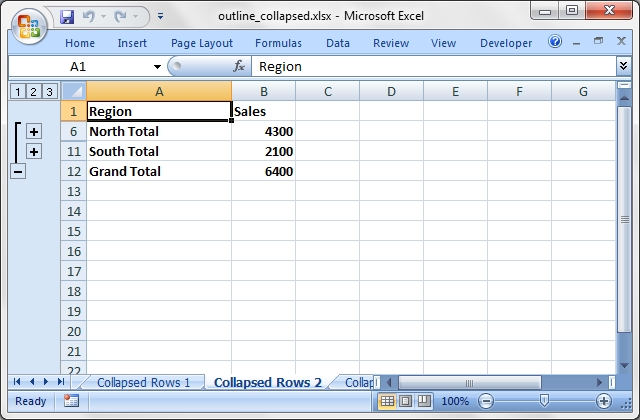Source code for this example:

``````    #!/usr/bin/perl

###############################################################################
#
# Example of how to use Excel::Writer::XLSX to generate Excel outlines and
# grouping.
#
# These examples focus mainly on collapsed outlines. See also the
# outlines.pl example program for more general examples.
#
# Copyright 2000-2021, John McNamara, jmcnamara@cpan.org
#

use strict;
use warnings;
use Excel::Writer::XLSX;

# Create a new workbook and add some worksheets
my \$workbook   = Excel::Writer::XLSX->new( 'outline_collapsed.xlsx' );
my \$worksheet1 = \$workbook->add_worksheet( 'Outlined Rows' );
my \$worksheet2 = \$workbook->add_worksheet( 'Collapsed Rows 1' );
my \$worksheet3 = \$workbook->add_worksheet( 'Collapsed Rows 2' );
my \$worksheet4 = \$workbook->add_worksheet( 'Collapsed Rows 3' );
my \$worksheet5 = \$workbook->add_worksheet( 'Outline Columns' );
my \$worksheet6 = \$workbook->add_worksheet( 'Collapsed Columns' );

# Add a general format
my \$bold = \$workbook->add_format( bold => 1 );

#
# This function will generate the same data and sub-totals on each worksheet.
#
sub create_sub_totals {

my \$worksheet = \$_;

# Add a column format for clarity
\$worksheet->set_column( 'A:A', 20 );

# Add the data, labels and formulas
\$worksheet->write( 'A1', 'Region', \$bold );
\$worksheet->write( 'A2', 'North' );
\$worksheet->write( 'A3', 'North' );
\$worksheet->write( 'A4', 'North' );
\$worksheet->write( 'A5', 'North' );
\$worksheet->write( 'A6', 'North Total', \$bold );

\$worksheet->write( 'B1', 'Sales', \$bold );
\$worksheet->write( 'B2', 1000 );
\$worksheet->write( 'B3', 1200 );
\$worksheet->write( 'B4', 900 );
\$worksheet->write( 'B5', 1200 );
\$worksheet->write( 'B6', '=SUBTOTAL(9,B2:B5)', \$bold );

\$worksheet->write( 'A7',  'South' );
\$worksheet->write( 'A8',  'South' );
\$worksheet->write( 'A9',  'South' );
\$worksheet->write( 'A10', 'South' );
\$worksheet->write( 'A11', 'South Total', \$bold );

\$worksheet->write( 'B7',  400 );
\$worksheet->write( 'B8',  600 );
\$worksheet->write( 'B9',  500 );
\$worksheet->write( 'B10', 600 );
\$worksheet->write( 'B11', '=SUBTOTAL(9,B7:B10)', \$bold );

\$worksheet->write( 'A12', 'Grand Total',         \$bold );
\$worksheet->write( 'B12', '=SUBTOTAL(9,B2:B10)', \$bold );

}

###############################################################################
#
# Example 1: Create a worksheet with outlined rows. It also includes SUBTOTAL()
# functions so that it looks like the type of automatic outlines that are
# generated when you use the Excel Data->SubTotals menu item.
#

# The syntax is: set_row(\$row, \$height, \$XF, \$hidden, \$level, \$collapsed)
\$worksheet1->set_row( 1, undef, undef, 0, 2 );
\$worksheet1->set_row( 2, undef, undef, 0, 2 );
\$worksheet1->set_row( 3, undef, undef, 0, 2 );
\$worksheet1->set_row( 4, undef, undef, 0, 2 );
\$worksheet1->set_row( 5, undef, undef, 0, 1 );

\$worksheet1->set_row( 6,  undef, undef, 0, 2 );
\$worksheet1->set_row( 7,  undef, undef, 0, 2 );
\$worksheet1->set_row( 8,  undef, undef, 0, 2 );
\$worksheet1->set_row( 9,  undef, undef, 0, 2 );
\$worksheet1->set_row( 10, undef, undef, 0, 1 );

# Write the sub-total data that is common to the row examples.
create_sub_totals( \$worksheet1 );

###############################################################################
#
# Example 2: Create a worksheet with collapsed outlined rows.
# This is the same as the example 1  except that the all rows are collapsed.
# Note: We need to indicate the row that contains the collapsed symbol '+' with
# the optional parameter, \$collapsed.

\$worksheet2->set_row( 1, undef, undef, 1, 2 );
\$worksheet2->set_row( 2, undef, undef, 1, 2 );
\$worksheet2->set_row( 3, undef, undef, 1, 2 );
\$worksheet2->set_row( 4, undef, undef, 1, 2 );
\$worksheet2->set_row( 5, undef, undef, 1, 1 );

\$worksheet2->set_row( 6,  undef, undef, 1, 2 );
\$worksheet2->set_row( 7,  undef, undef, 1, 2 );
\$worksheet2->set_row( 8,  undef, undef, 1, 2 );
\$worksheet2->set_row( 9,  undef, undef, 1, 2 );
\$worksheet2->set_row( 10, undef, undef, 1, 1 );

\$worksheet2->set_row( 11, undef, undef, 0, 0, 1 );

# Write the sub-total data that is common to the row examples.
create_sub_totals( \$worksheet2 );

###############################################################################
#
# Example 3: Create a worksheet with collapsed outlined rows.
# Same as the example 1  except that the two sub-totals are collapsed.

\$worksheet3->set_row( 1, undef, undef, 1, 2 );
\$worksheet3->set_row( 2, undef, undef, 1, 2 );
\$worksheet3->set_row( 3, undef, undef, 1, 2 );
\$worksheet3->set_row( 4, undef, undef, 1, 2 );
\$worksheet3->set_row( 5, undef, undef, 0, 1, 1 );

\$worksheet3->set_row( 6,  undef, undef, 1, 2 );
\$worksheet3->set_row( 7,  undef, undef, 1, 2 );
\$worksheet3->set_row( 8,  undef, undef, 1, 2 );
\$worksheet3->set_row( 9,  undef, undef, 1, 2 );
\$worksheet3->set_row( 10, undef, undef, 0, 1, 1 );

# Write the sub-total data that is common to the row examples.
create_sub_totals( \$worksheet3 );

###############################################################################
#
# Example 4: Create a worksheet with outlined rows.
# Same as the example 1  except that the two sub-totals are collapsed.

\$worksheet4->set_row( 1, undef, undef, 1, 2 );
\$worksheet4->set_row( 2, undef, undef, 1, 2 );
\$worksheet4->set_row( 3, undef, undef, 1, 2 );
\$worksheet4->set_row( 4, undef, undef, 1, 2 );
\$worksheet4->set_row( 5, undef, undef, 1, 1, 1 );

\$worksheet4->set_row( 6,  undef, undef, 1, 2 );
\$worksheet4->set_row( 7,  undef, undef, 1, 2 );
\$worksheet4->set_row( 8,  undef, undef, 1, 2 );
\$worksheet4->set_row( 9,  undef, undef, 1, 2 );
\$worksheet4->set_row( 10, undef, undef, 1, 1, 1 );

\$worksheet4->set_row( 11, undef, undef, 0, 0, 1 );

# Write the sub-total data that is common to the row examples.
create_sub_totals( \$worksheet4 );

###############################################################################
#
# Example 5: Create a worksheet with outlined columns.
#
my \$data = [
[ 'Month', 'Jan', 'Feb', 'Mar', 'Apr', 'May', 'Jun', 'Total' ],
[ 'North', 50,    20,    15,    25,    65,    80,,   '=SUM(B2:G2)' ],
[ 'South', 10,    20,    30,    50,    50,    50,,   '=SUM(B3:G3)' ],
[ 'East',  45,    75,    50,    15,    75,    100,,  '=SUM(B4:G4)' ],
[ 'West',  15,    15,    55,    35,    20,    50,,   '=SUM(B5:G6)' ],
];

# Add bold format to the first row
\$worksheet5->set_row( 0, undef, \$bold );

# Syntax: set_column(\$col1, \$col2, \$width, \$XF, \$hidden, \$level, \$collapsed)
\$worksheet5->set_column( 'A:A', 10, \$bold );
\$worksheet5->set_column( 'B:G', 5, undef, 0, 1 );
\$worksheet5->set_column( 'H:H', 10 );

# Write the data and a formula
\$worksheet5->write_col( 'A1', \$data );
\$worksheet5->write( 'H6', '=SUM(H2:H5)', \$bold );

###############################################################################
#
# Example 6: Create a worksheet with collapsed outlined columns.
# This is the same as the previous example except collapsed columns.

# Add bold format to the first row
\$worksheet6->set_row( 0, undef, \$bold );

# Syntax: set_column(\$col1, \$col2, \$width, \$XF, \$hidden, \$level, \$collapsed)
\$worksheet6->set_column( 'A:A', 10, \$bold );
\$worksheet6->set_column( 'B:G', 5,  undef, 1, 1 );
\$worksheet6->set_column( 'H:H', 10, undef, 0, 0, 1 );

# Write the data and a formula
\$worksheet6->write_col( 'A1', \$data );
\$worksheet6->write( 'H6', '=SUM(H2:H5)', \$bold );

\$workbook->close();

__END__``````

## Example: panes.pl

Example of using the Excel::Writer::XLSX module to create worksheet panes.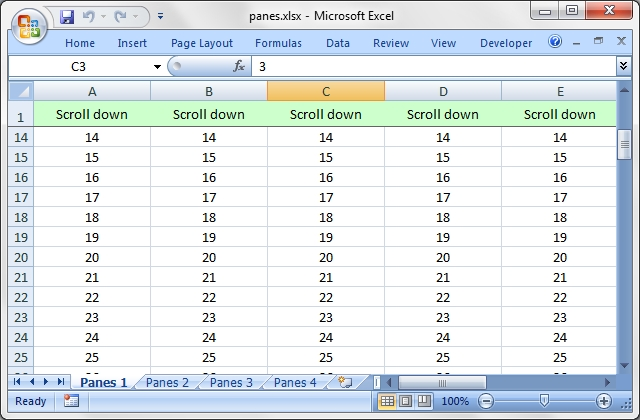Source code for this example:

``````    #!/usr/bin/perl

#######################################################################
#
# Example of using the Excel::Writer::XLSX module to create worksheet panes.
#
# Copyright 2000-2021, John McNamara, jmcnamara@cpan.org
#

use strict;
use warnings;
use Excel::Writer::XLSX;

my \$workbook = Excel::Writer::XLSX->new( 'panes.xlsx' );

my \$worksheet1 = \$workbook->add_worksheet( 'Panes 1' );
my \$worksheet2 = \$workbook->add_worksheet( 'Panes 2' );
my \$worksheet3 = \$workbook->add_worksheet( 'Panes 3' );
my \$worksheet4 = \$workbook->add_worksheet( 'Panes 4' );

# Freeze panes
\$worksheet1->freeze_panes( 1, 0 );    # 1 row

\$worksheet2->freeze_panes( 0, 1 );    # 1 column
\$worksheet3->freeze_panes( 1, 1 );    # 1 row and column

# Split panes.
# The divisions must be specified in terms of row and column dimensions.
# The default row height is 15 and the default column width is 8.43
#
\$worksheet4->split_panes( 15, 8.43 );    # 1 row and column

#######################################################################
#
# Set up some formatting and text to highlight the panes
#

align    => 'center',
valign   => 'vcenter',
fg_color => '#C3FFC0',
);

my \$center = \$workbook->add_format( align => 'center' );

#######################################################################
#
# Sheet 1
#

\$worksheet1->set_column( 'A:I', 16 );
\$worksheet1->set_row( 0, 20 );
\$worksheet1->set_selection( 'C3' );

for my \$i ( 0 .. 8 ) {
\$worksheet1->write( 0, \$i, 'Scroll down', \$header );
}

for my \$i ( 1 .. 100 ) {
for my \$j ( 0 .. 8 ) {
\$worksheet1->write( \$i, \$j, \$i + 1, \$center );
}
}

#######################################################################
#
# Sheet 2
#

\$worksheet2->set_column( 'A:A', 16 );
\$worksheet2->set_selection( 'C3' );

for my \$i ( 0 .. 49 ) {
\$worksheet2->set_row( \$i, 15 );
\$worksheet2->write( \$i, 0, 'Scroll right', \$header );
}

for my \$i ( 0 .. 49 ) {
for my \$j ( 1 .. 25 ) {
\$worksheet2->write( \$i, \$j, \$j, \$center );
}
}

#######################################################################
#
# Sheet 3
#

\$worksheet3->set_column( 'A:Z', 16 );
\$worksheet3->set_selection( 'C3' );

\$worksheet3->write( 0, 0, '', \$header );

for my \$i ( 1 .. 25 ) {
\$worksheet3->write( 0, \$i, 'Scroll down', \$header );
}

for my \$i ( 1 .. 49 ) {
\$worksheet3->write( \$i, 0, 'Scroll right', \$header );
}

for my \$i ( 1 .. 49 ) {
for my \$j ( 1 .. 25 ) {
\$worksheet3->write( \$i, \$j, \$j, \$center );
}
}

#######################################################################
#
# Sheet 4
#

\$worksheet4->set_selection( 'C3' );

for my \$i ( 1 .. 25 ) {
\$worksheet4->write( 0, \$i, 'Scroll', \$center );
}

for my \$i ( 1 .. 49 ) {
\$worksheet4->write( \$i, 0, 'Scroll', \$center );
}

for my \$i ( 1 .. 49 ) {
for my \$j ( 1 .. 25 ) {
\$worksheet4->write( \$i, \$j, \$j, \$center );
}
}

\$workbook->close();

__END__``````

## Example: properties.pl

An example of adding document properties to a Excel::Writer::XLSX file.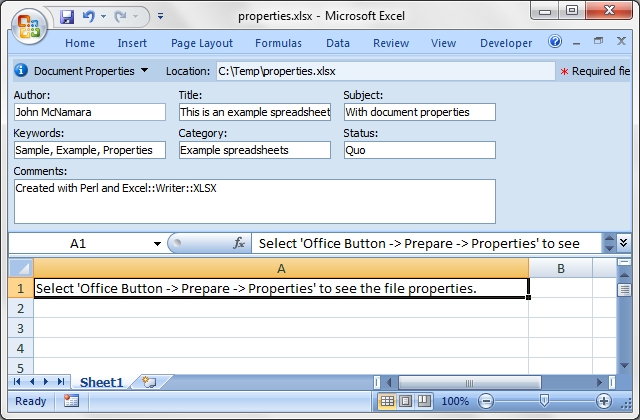Source code for this example:

``````    #!/usr/bin/perl

##############################################################################
#
# An example of adding document properties to a Excel::Writer::XLSX file.
#
# Copyright 2000-2021, John McNamara, jmcnamara@cpan.org
#

use strict;
use warnings;
use Excel::Writer::XLSX;

my \$workbook  = Excel::Writer::XLSX->new( 'properties.xlsx' );
my \$worksheet = \$workbook->add_worksheet();

\$workbook->set_properties(
title    => 'This is an example spreadsheet',
subject  => 'With document properties',
author   => 'John McNamara',
manager  => 'Dr. Heinz Doofenshmirtz',
company  => 'of Wolves',
category => 'Example spreadsheets',
keywords => 'Sample, Example, Properties',
comments => 'Created with Perl and Excel::Writer::XLSX',
status   => 'Quo',
);

\$worksheet->set_column( 'A:A', 70 );
\$worksheet->write( 'A1', qq{Select 'Office Button -> Prepare -> Properties' to see the file properties.} );

\$workbook->close();

__END__``````

## Example: protection.pl

Example of cell locking and formula hiding in an Excel worksheet via the Excel::Writer::XLSX module.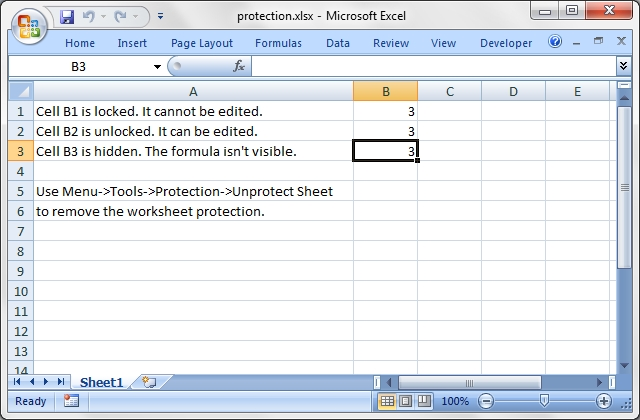Source code for this example:

``````    #!/usr/bin/perl

########################################################################
#
# Example of cell locking and formula hiding in an Excel worksheet via
# the Excel::Writer::XLSX module.
#
# Copyright 2000-2021, John McNamara, jmcnamara@cpan.org
#

use strict;
use warnings;
use Excel::Writer::XLSX;

my \$workbook  = Excel::Writer::XLSX->new( 'protection.xlsx' );
my \$worksheet = \$workbook->add_worksheet();

# Create some format objects
my \$unlocked = \$workbook->add_format( locked => 0 );
my \$hidden   = \$workbook->add_format( hidden => 1 );

# Format the columns
\$worksheet->set_column( 'A:A', 45 );
\$worksheet->set_selection( 'B3' );

# Protect the worksheet
\$worksheet->protect();

# Examples of cell locking and hiding.
\$worksheet->write( 'A1', 'Cell B1 is locked. It cannot be edited.' );
\$worksheet->write_formula( 'B1', '=1+2', undef, 3 );    # Locked by default.

\$worksheet->write( 'A2', 'Cell B2 is unlocked. It can be edited.' );
\$worksheet->write_formula( 'B2', '=1+2', \$unlocked, 3 );

\$worksheet->write( 'A3', "Cell B3 is hidden. The formula isn't visible." );
\$worksheet->write_formula( 'B3', '=1+2', \$hidden, 3 );

\$worksheet->write( 'A5', 'Use Menu->Tools->Protection->Unprotect Sheet' );
\$worksheet->write( 'A6', 'to remove the worksheet protection.' );

\$workbook->close();

__END__
``````

## Example: rich_strings.pl

An Excel::Writer::XLSX example showing how to use "rich strings", i.e., strings with multiple formatting.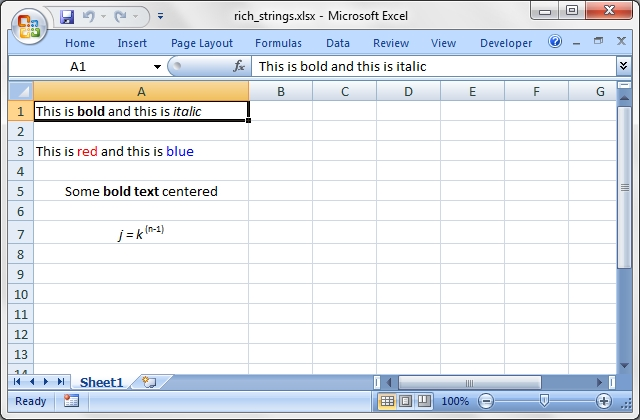Source code for this example:

``````    #!/usr/bin/perl

#######################################################################
#
# An Excel::Writer::XLSX example showing how to use "rich strings", i.e.,
# strings with multiple formatting.
#
# Copyright 2000-2021, John McNamara, jmcnamara@cpan.org
#

use strict;
use warnings;
use Excel::Writer::XLSX;

my \$workbook  = Excel::Writer::XLSX->new( 'rich_strings.xlsx' );
my \$worksheet = \$workbook->add_worksheet();

\$worksheet->set_column( 'A:A', 30 );

# Set some formats to use.
my \$bold   = \$workbook->add_format( bold        => 1 );
my \$italic = \$workbook->add_format( italic      => 1 );
my \$red    = \$workbook->add_format( color       => 'red' );
my \$blue   = \$workbook->add_format( color       => 'blue' );
my \$center = \$workbook->add_format( align       => 'center' );
my \$super  = \$workbook->add_format( font_script => 1 );

# Write some strings with multiple formats.
\$worksheet->write_rich_string( 'A1',
'This is ', \$bold, 'bold', ' and this is ', \$italic, 'italic' );

\$worksheet->write_rich_string( 'A3',
'This is ', \$red, 'red', ' and this is ', \$blue, 'blue' );

\$worksheet->write_rich_string( 'A5',
'Some ', \$bold, 'bold text', ' centered', \$center );

\$worksheet->write_rich_string( 'A7',
\$italic, 'j = k', \$super, '(n-1)', \$center );

\$workbook->close();

__END__``````

## Example: right_to_left.pl

Example of how to change the default worksheet direction from left-to-right to right-to-left as required by some eastern verions of Excel.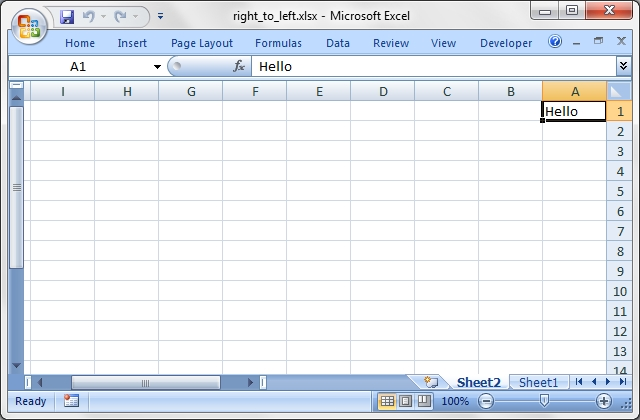Source code for this example:

``````    #!/usr/bin/perl

#######################################################################
#
# Example of how to change the default worksheet direction from
# left-to-right to right-to-left as required by some eastern verions
# of Excel.
#
# Copyright 2000-2021, John McNamara, jmcnamara@cpan.org
#

use strict;
use warnings;
use Excel::Writer::XLSX;

my \$workbook   = Excel::Writer::XLSX->new( 'right_to_left.xlsx' );
my \$worksheet1 = \$workbook->add_worksheet();
my \$worksheet2 = \$workbook->add_worksheet();

\$worksheet2->right_to_left();

\$worksheet1->write( 0, 0, 'Hello' );    #  A1, B1, C1, ...
\$worksheet2->write( 0, 0, 'Hello' );    # ..., C1, B1, A1

\$workbook->close();

__END__``````

## Example: sales.pl

Example of a sales worksheet to demonstrate several different features. Also uses functions from the Excel::Writer::XLSX::Utility module.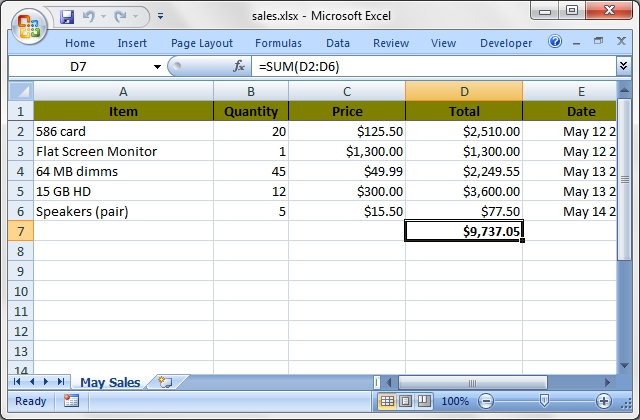Source code for this example:

``````    #!/usr/bin/perl -w

###############################################################################
#
# Example of a sales worksheet to demonstrate several different features.
# Also uses functions from the L<Excel::Writer::XLSX::Utility> module.
#
# Copyright 2000-2021, John McNamara, jmcnamara@cpan.org
#

use strict;
use Excel::Writer::XLSX;
use Excel::Writer::XLSX::Utility;

# Create a new workbook and add a worksheet
my \$workbook  = Excel::Writer::XLSX->new( 'sales.xlsx' );
my \$worksheet = \$workbook->add_worksheet( 'May Sales' );

# Set up some formats
my %heading = (
bold     => 1,
pattern  => 1,
fg_color => '#C3FFC0',
border   => 1,
align    => 'center',
);

my %total = (
bold       => 1,
top        => 1,
num_format => '\$#,##0.00'
);

my \$total_format = \$workbook->add_format( %total );
my \$price_format = \$workbook->add_format( num_format => '\$#,##0.00' );
my \$date_format  = \$workbook->add_format( num_format => 'mmm d yyy' );

# Write the main headings
\$worksheet->freeze_panes( 1 );    # Freeze the first row
\$worksheet->write( 'A1', 'Item',     \$heading );
\$worksheet->write( 'B1', 'Quantity', \$heading );
\$worksheet->write( 'C1', 'Price',    \$heading );
\$worksheet->write( 'D1', 'Total',    \$heading );
\$worksheet->write( 'E1', 'Date',     \$heading );

# Set the column widths
\$worksheet->set_column( 'A:A', 25 );
\$worksheet->set_column( 'B:B', 10 );
\$worksheet->set_column( 'C:E', 16 );

# Extract the sales data from the __DATA__ section at the end of the file.
# In reality this information would probably come from a database
my @sales;

foreach my \$line ( <DATA> ) {
chomp \$line;
next if \$line eq '';

# Simple-minded processing of CSV data. Refer to the Text::CSV_XS
# and Text::xSV modules for a more complete CSV handling.
my @items = split /,/, \$line;
push @sales, \@items;
}

# Write out the items from each row
my \$row = 1;
foreach my \$sale ( @sales ) {

\$worksheet->write( \$row, 0, @\$sale );
\$worksheet->write( \$row, 1, @\$sale );
\$worksheet->write( \$row, 2, @\$sale, \$price_format );

# Create a formula like '=B2*C2'
my \$formula =
'=' . xl_rowcol_to_cell( \$row, 1 ) . "*" . xl_rowcol_to_cell( \$row, 2 );

\$worksheet->write( \$row, 3, \$formula, \$price_format );

# Parse the date
my \$date = xl_decode_date_US( @\$sale );
\$worksheet->write( \$row, 4, \$date, \$date_format );
\$row++;
}

# Create a formula to sum the totals, like '=SUM(D2:D6)'
my \$total = '=SUM(D2:' . xl_rowcol_to_cell( \$row - 1, 3 ) . ")";

\$worksheet->write( \$row, 3, \$total, \$total_format );

\$workbook->close();

__DATA__
586 card,20,125.50,5/12/01
Flat Screen Monitor,1,1300.00,5/12/01
64 MB dimms,45,49.99,5/13/01
15 GB HD,12,300.00,5/13/01
Speakers (pair),5,15.50,5/14/01
``````

## Example: shape1.pl

A simple example of how to use the Excel::Writer::XLSX module to add shapes to an Excel xlsx file.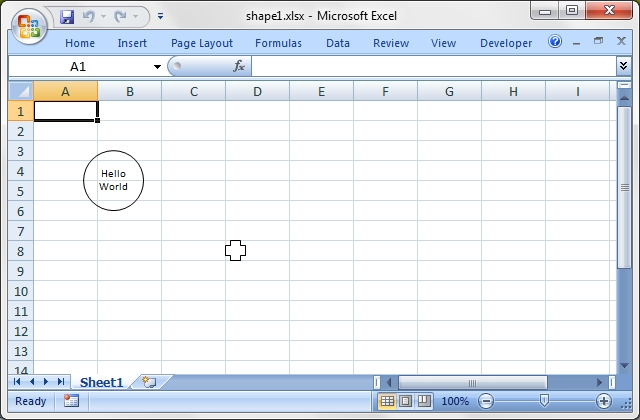Source code for this example:

``````    #!/usr/bin/perl

#######################################################################
#
# A simple example of how to use the Excel::Writer::XLSX module to
# add shapes to an Excel xlsx file.
#
# Copyright 2000-2021, John McNamara, jmcnamara@cpan.org
#

use strict;
use warnings;
use Excel::Writer::XLSX;

my \$workbook  = Excel::Writer::XLSX->new( 'shape1.xlsx' );
my \$worksheet = \$workbook->add_worksheet();

# Add a circle, with centered text.
my \$ellipse = \$workbook->add_shape(
type   => 'ellipse',
text   => "Hello\nWorld",
width  => 60,
height => 60
);

\$worksheet->insert_shape( 'A1', \$ellipse, 50, 50 );

# Add a plus sign.
my \$plus = \$workbook->add_shape( type => 'plus', width => 20, height => 20 );
\$worksheet->insert_shape( 'D8', \$plus );

\$workbook->close();

__END__``````

## Example: shape2.pl

A simple example of how to use the Excel::Writer::XLSX module to modify shape properties in an Excel xlsx file.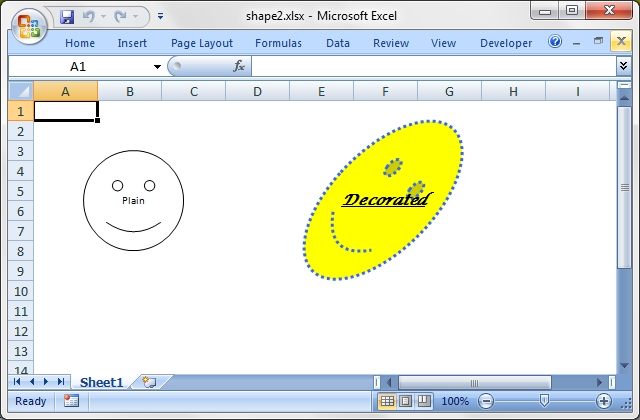Source code for this example:

``````    #!/usr/bin/perl

#######################################################################
#
# A simple example of how to use the Excel::Writer::XLSX module to
# modify shape properties in an Excel xlsx file.
#
# Copyright 2000-2021, John McNamara, jmcnamara@cpan.org
#

use strict;
use warnings;
use Excel::Writer::XLSX;

my \$workbook  = Excel::Writer::XLSX->new( 'shape2.xlsx' );
my \$worksheet = \$workbook->add_worksheet();

\$worksheet->hide_gridlines( 2 );

my \$plain = \$workbook->add_shape(
type   => 'smileyFace',
text   => "Plain",
width  => 100,
height => 100,
);

my \$bbformat = \$workbook->add_format(
color => 'red',
font  => 'Lucida Calligraphy',
);

\$bbformat->set_bold();
\$bbformat->set_underline();
\$bbformat->set_italic();

my \$decor = \$workbook->add_shape(
type        => 'smileyFace',
text        => "Decorated",
rotation    => 45,
width       => 200,
height      => 100,
format      => \$bbformat,
line_type   => 'sysDot',
line_weight => 3,
fill        => 'FFFF00',
line        => '3366FF',
);

\$worksheet->insert_shape( 'A1', \$plain, 50,  50 );
\$worksheet->insert_shape( 'A1', \$decor, 250, 50 );

\$workbook->close();

__END__``````

## Example: shape3.pl

A simple example of how to use the Excel::Writer::XLSX module to scale shapes in an Excel xlsx file.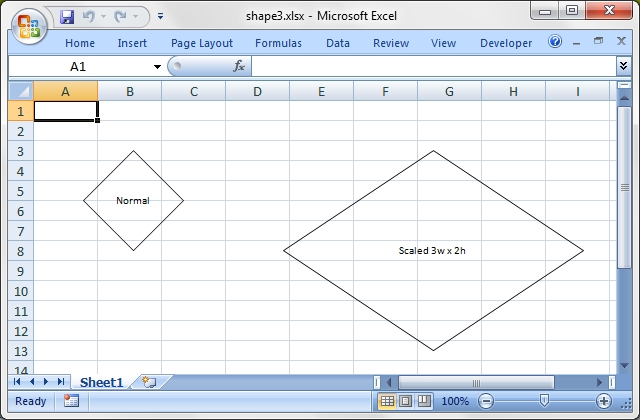Source code for this example:

``````    #!/usr/bin/perl

#######################################################################
#
# A simple example of how to use the Excel::Writer::XLSX module to
# scale shapes in an Excel xlsx file.
#
# Copyright 2000-2021, John McNamara, jmcnamara@cpan.org
#

use strict;
use warnings;
use Excel::Writer::XLSX;

my \$workbook  = Excel::Writer::XLSX->new( 'shape3.xlsx' );
my \$worksheet = \$workbook->add_worksheet();

my \$normal = \$workbook->add_shape(
name   => 'chip',
type   => 'diamond',
text   => "Normal",
width  => 100,
height => 100,
);

\$worksheet->insert_shape( 'A1', \$normal, 50, 50 );
\$normal->set_text( 'Scaled 3w x 2h' );
\$normal->set_name( 'Hope' );
\$worksheet->insert_shape( 'A1', \$normal, 250, 50, 3, 2 );

\$workbook->close();

__END__``````

## Example: shape4.pl

A simple example of how to use the Excel::Writer::XLSX module to demonstrate stenciling in an Excel xlsx file.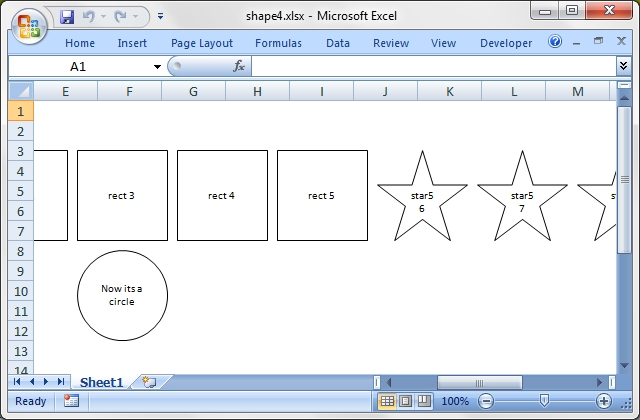Source code for this example:

``````    #!/usr/bin/perl

#######################################################################
#
# A simple example of how to use the Excel::Writer::XLSX module to
# demonstrate stenciling in an Excel xlsx file.
#
# Copyright 2000-2021, John McNamara, jmcnamara@cpan.org
#

use strict;
use warnings;
use Excel::Writer::XLSX;

my \$workbook  = Excel::Writer::XLSX->new( 'shape4.xlsx' );
my \$worksheet = \$workbook->add_worksheet();

\$worksheet->hide_gridlines( 2 );

my \$type  = 'rect';
my \$shape = \$workbook->add_shape(
type   => \$type,
width  => 90,
height => 90,
);

for my \$n ( 1 .. 10 ) {

# Change the last 5 rectangles to stars. Previously inserted shapes stay
# as rectangles.
\$type = 'star5' if \$n == 6;
\$shape->set_type( \$type );
\$shape->set_text( "\$type \$n" );
\$worksheet->insert_shape( 'A1', \$shape, \$n * 100, 50 );
}

my \$stencil = \$workbook->add_shape(
stencil => 1,                    # The default.
width   => 90,
height  => 90,
text    => 'started as a box',
);
\$worksheet->insert_shape( 'A1', \$stencil, 100, 150 );

\$stencil->set_stencil( 0 );
\$worksheet->insert_shape( 'A1', \$stencil, 200, 150 );
\$worksheet->insert_shape( 'A1', \$stencil, 300, 150 );

# Ooops!  Changed my mind.  Change the rectangle to an ellipse (circle),
# for the last two shapes.
\$stencil->set_type( 'ellipse' );
\$stencil->set_text( 'Now its a circle' );

\$workbook->close();

__END__``````

## Example: shape5.pl

A simple example of how to use the Excel::Writer::XLSX module to add shapes (objects and top/bottom connectors) to an Excel xlsx file.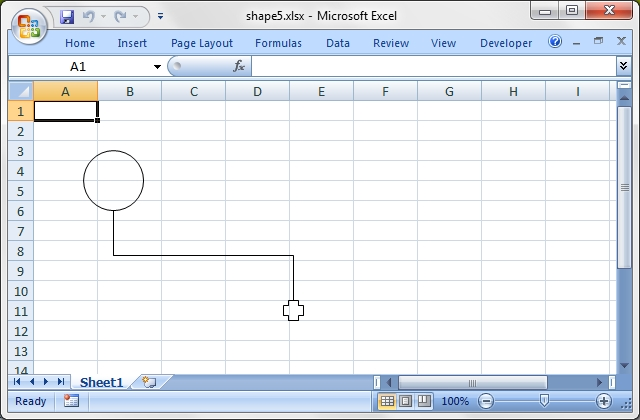Source code for this example:

``````    #!/usr/bin/perl

#######################################################################
#
# A simple example of how to use the Excel::Writer::XLSX module to
# add shapes (objects and top/bottom connectors) to an Excel xlsx file.
#
# Copyright 2000-2021, John McNamara, jmcnamara@cpan.org
#

use strict;
use warnings;
use Excel::Writer::XLSX;

my \$workbook  = Excel::Writer::XLSX->new( 'shape5.xlsx' );
my \$worksheet = \$workbook->add_worksheet();

my \$s1 = \$workbook->add_shape( type => 'ellipse', width => 60, height => 60 );
\$worksheet->insert_shape( 'A1', \$s1, 50, 50 );

my \$s2 = \$workbook->add_shape( type => 'plus', width => 20, height => 20 );
\$worksheet->insert_shape( 'A1', \$s2, 250, 200 );

# Create a connector to link the two shapes.
my \$cxn_shape = \$workbook->add_shape( type => 'bentConnector3' );

# Link the start of the connector to the right side.
\$cxn_shape->set_start( \$s1->get_id() );
\$cxn_shape->set_start_index( 4 );  # 4th connection pt, clockwise from top(0).
\$cxn_shape->set_start_side( 'b' ); # r)ight or b)ottom.

# Link the end of the connector to the left side.
\$cxn_shape->set_end( \$s2->get_id() );
\$cxn_shape->set_end_index( 0 );     # clockwise from top(0).
\$cxn_shape->set_end_side( 't' );    # t)top.

\$worksheet->insert_shape( 'A1', \$cxn_shape, 0, 0 );

\$workbook->close();

__END__``````

## Example: shape6.pl

A simple example of how to use the Excel::Writer::XLSX module to add shapes (objects and right/left connectors) to an Excel xlsx file.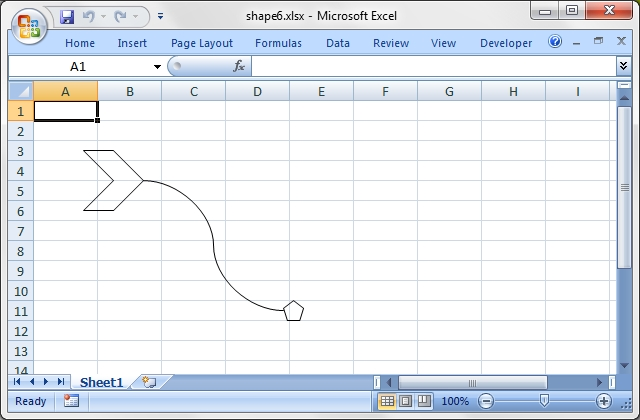Source code for this example:

``````    #!/usr/bin/perl

#######################################################################
#
# A simple example of how to use the Excel::Writer::XLSX module to
# add shapes (objects and right/left connectors) to an Excel xlsx file.
#
# Copyright 2000-2021, John McNamara, jmcnamara@cpan.org
#

use strict;
use warnings;
use Excel::Writer::XLSX;

my \$workbook  = Excel::Writer::XLSX->new( 'shape6.xlsx' );
my \$worksheet = \$workbook->add_worksheet();

my \$s1 = \$workbook->add_shape( type => 'chevron', width => 60, height => 60 );
\$worksheet->insert_shape( 'A1', \$s1, 50, 50 );

my \$s2 = \$workbook->add_shape( type => 'pentagon', width => 20, height => 20 );
\$worksheet->insert_shape( 'A1', \$s2, 250, 200 );

# Create a connector to link the two shapes.
my \$cxn_shape = \$workbook->add_shape( type => 'curvedConnector3' );

# Link the start of the connector to the right side.
\$cxn_shape->set_start( \$s1->get_id() );
\$cxn_shape->set_start_index( 2 );    # 2nd connection pt, clockwise from top(0).
\$cxn_shape->set_start_side( 'r' );   # r)ight or b)ottom.

# Link the end of the connector to the left side.
\$cxn_shape->set_end( \$s2->get_id() );
\$cxn_shape->set_end_index( 4 );      # 4th connection pt, clockwise from top(0).
\$cxn_shape->set_end_side( 'l' );     # l)eft or t)op.

\$worksheet->insert_shape( 'A1', \$cxn_shape, 0, 0 );

\$workbook->close();

__END__``````

## Example: shape7.pl

A simple example of how to use the Excel::Writer::XLSX module to add shapes and one-to-many connectors to an Excel xlsx file.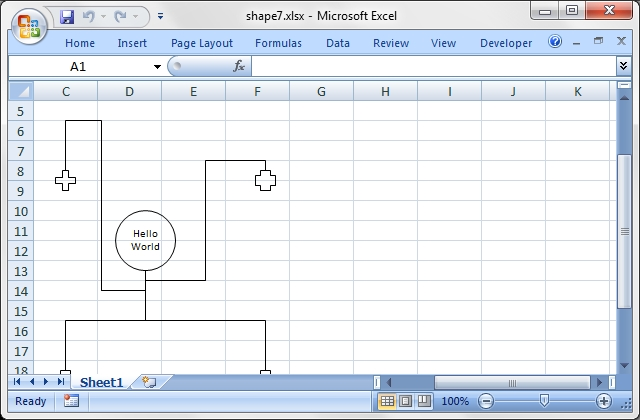Source code for this example:

``````    #!/usr/bin/perl

#######################################################################
#
# A simple example of how to use the Excel::Writer::XLSX module to
# add shapes and one-to-many connectors to an Excel xlsx file.
#
# Copyright 2000-2021, John McNamara, jmcnamara@cpan.org
#

use strict;
use warnings;
use Excel::Writer::XLSX;

my \$workbook  = Excel::Writer::XLSX->new( 'shape7.xlsx' );
my \$worksheet = \$workbook->add_worksheet();

# Add a circle, with centered text. c is for circle, not center.
my \$cw = 60;
my \$ch = 60;
my \$cx = 210;
my \$cy = 190;

my \$ellipse = \$workbook->add_shape(
type   => 'ellipse',
id     => 2,
text   => "Hello\nWorld",
width  => \$cw,
height => \$ch
);
\$worksheet->insert_shape( 'A1', \$ellipse, \$cx, \$cy );

# Add a plus sign at 4 different positions around the circle.
my \$pw = 20;
my \$ph = 20;
my \$px = 120;
my \$py = 250;
my \$plus =
\$workbook->add_shape( type => 'plus', id => 3, width => \$pw, height => \$ph );
my \$p1 = \$worksheet->insert_shape( 'A1', \$plus, 350, 350 );
my \$p2 = \$worksheet->insert_shape( 'A1', \$plus, 150, 350 );
my \$p3 = \$worksheet->insert_shape( 'A1', \$plus, 350, 150 );
\$plus->set_adjustments( 35 );    # change shape of plus symbol.
my \$p4 = \$worksheet->insert_shape( 'A1', \$plus, 150, 150 );

my \$cxn_shape = \$workbook->add_shape( type => 'bentConnector3', fill => 0 );

\$cxn_shape->set_start( \$ellipse->get_id() );
\$cxn_shape->set_start_index( 4 );    # 4nd connection pt, clockwise from top(0).
\$cxn_shape->set_start_side( 'b' );   # r)ight or b)ottom.

\$cxn_shape->set_end( \$p1->get_id() );
\$cxn_shape->set_end_index( 0 );
\$cxn_shape->set_end_side( 't' );
\$worksheet->insert_shape( 'A1', \$cxn_shape, 0, 0 );

\$cxn_shape->set_end( \$p2->get_id() );
\$worksheet->insert_shape( 'A1', \$cxn_shape, 0, 0 );

\$cxn_shape->set_end( \$p3->get_id() );
\$worksheet->insert_shape( 'A1', \$cxn_shape, 0, 0 );

\$cxn_shape->set_end( \$p4->get_id() );
\$cxn_shape->set_adjustments( -50, 45, 120 );
\$worksheet->insert_shape( 'A1', \$cxn_shape, 0, 0 );

\$workbook->close();

__END__
``````

## Example: shape8.pl

A simple example of how to use the Excel::Writer::XLSX module to add shapes and one-to-many connectors to an Excel xlsx file.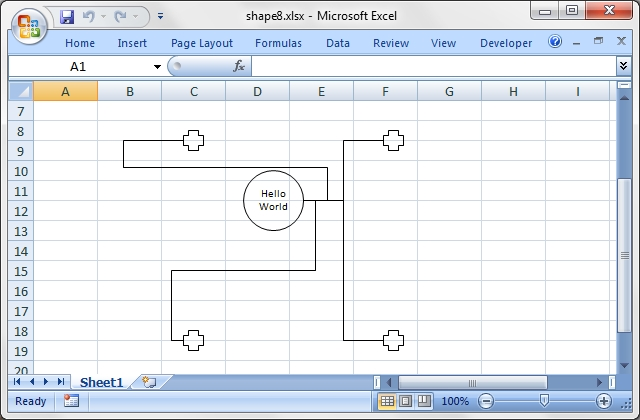Source code for this example:

``````    #!/usr/bin/perl

#######################################################################
#
# A simple example of how to use the Excel::Writer::XLSX module to
# add shapes and one-to-many connectors to an Excel xlsx file.
#
# Copyright 2000-2021, John McNamara, jmcnamara@cpan.org
#

use strict;
use warnings;
use Excel::Writer::XLSX;

my \$workbook  = Excel::Writer::XLSX->new( 'shape8.xlsx' );
my \$worksheet = \$workbook->add_worksheet();

# Add a circle, with centered text. c is for circle, not center.
my \$cw = 60;
my \$ch = 60;
my \$cx = 210;
my \$cy = 190;

my \$ellipse = \$workbook->add_shape(
type   => 'ellipse',
id     => 2,
text   => "Hello\nWorld",
width  => \$cw,
height => \$ch
);
\$worksheet->insert_shape( 'A1', \$ellipse, \$cx, \$cy );

# Add a plus sign at 4 different positions around the circle.
my \$pw = 20;
my \$ph = 20;
my \$px = 120;
my \$py = 250;
my \$plus =
\$workbook->add_shape( type => 'plus', id => 3, width => \$pw, height => \$ph );
my \$p1 = \$worksheet->insert_shape( 'A1', \$plus, 350, 150 );    #  2:00
my \$p2 = \$worksheet->insert_shape( 'A1', \$plus, 350, 350 );    #  4:00
my \$p3 = \$worksheet->insert_shape( 'A1', \$plus, 150, 350 );    #  8:00
my \$p4 = \$worksheet->insert_shape( 'A1', \$plus, 150, 150 );    # 10:00

my \$cxn_shape = \$workbook->add_shape( type => 'bentConnector3', fill => 0 );

\$cxn_shape->set_start( \$ellipse->get_id() );
\$cxn_shape->set_start_index( 2 );    # 2nd connection pt, clockwise from top(0).
\$cxn_shape->set_start_side( 'r' );   # r)ight or b)ottom.

\$cxn_shape->set_end( \$p1->get_id() );
\$cxn_shape->set_end_index( 3 );      # 3rd connection point on plus, right side
\$cxn_shape->set_end_side( 'l' );
\$worksheet->insert_shape( 'A1', \$cxn_shape, 0, 0 );

\$cxn_shape->set_end( \$p2->get_id() );
\$worksheet->insert_shape( 'A1', \$cxn_shape, 0, 0 );

\$cxn_shape->set_end( \$p3->get_id() );
\$worksheet->insert_shape( 'A1', \$cxn_shape, 0, 0 );

\$cxn_shape->set_end( \$p4->get_id() );
\$cxn_shape->set_adjustments( -50, 45, 120 );
\$worksheet->insert_shape( 'A1', \$cxn_shape, 0, 0 );

\$workbook->close();

__END__
``````

## Example: shape_all.pl

A simple example of how to use the Excel::Writer::XLSX module to add all shapes (as currently implemented) to an Excel xlsx file.

The list at the end consists of all the shape types defined as ST_ShapeType in ECMA-376, Office Open XML File Formats Part 4.

The grouping by worksheet name is for illustration only. It isn't part of the ECMA-376 standard.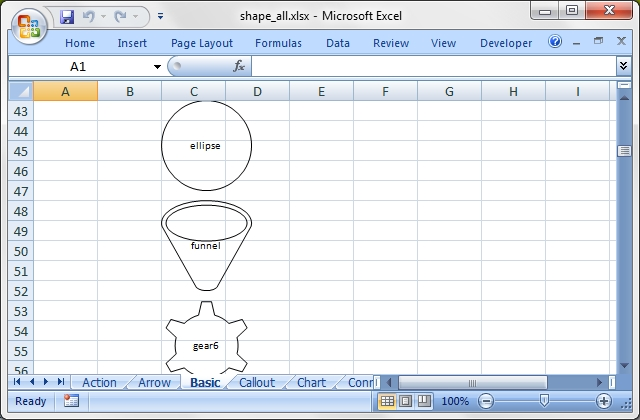Source code for this example:

``````    #!/usr/bin/perl

#######################################################################
#
# A simple example of how to use the Excel::Writer::XLSX module to
# add all shapes (as currently implemented) to an Excel xlsx file.
#
# The list at the end consists of all the shape types defined as
# ST_ShapeType in ECMA-376, Office Open XML File Formats Part 4.
#
# The grouping by worksheet name is for illustration only. It isn't
# part of the ECMA-376 standard.
#
# Copyright 2000-2021, John McNamara, jmcnamara@cpan.org
#

use strict;
use warnings;
use Excel::Writer::XLSX;

my \$workbook = Excel::Writer::XLSX->new( 'shape_all.xlsx' );

my ( \$worksheet, \$last_sheet, \$shape, \$r ) = ( 0, '', '', undef, 0 );

while ( <DATA> ) {
chomp;
next unless m/^\w/;    # Skip blank lines and comments.

my ( \$sheet, \$name ) = split( /\t/, \$_ );
if ( \$last_sheet ne \$sheet ) {
\$worksheet = \$workbook->add_worksheet( \$sheet );
\$r         = 2;
}
\$last_sheet = \$sheet;
type   => \$name,
text   => \$name,
width  => 90,
height => 90
);

# Connectors can not have labels, so write the connector name in the cell
# to the left.
\$worksheet->write( \$r, 0, \$name ) if \$sheet eq 'Connector';
\$worksheet->insert_shape( \$r, 2, \$shape, 0, 0 );
\$r += 5;
}

\$workbook->close();

__END__
Action      actionButtonBackPrevious
Action      actionButtonBeginning
Action      actionButtonBlank
Action      actionButtonDocument
Action      actionButtonEnd
Action      actionButtonForwardNext
Action      actionButtonHelp
Action      actionButtonHome
Action      actionButtonInformation
Action      actionButtonMovie
Action      actionButtonReturn
Action      actionButtonSound
Arrow       bentArrow
Arrow       bentUpArrow
Arrow       circularArrow
Arrow       curvedDownArrow
Arrow       curvedLeftArrow
Arrow       curvedRightArrow
Arrow       curvedUpArrow
Arrow       downArrow
Arrow       leftArrow
Arrow       leftCircularArrow
Arrow       leftRightArrow
Arrow       leftRightCircularArrow
Arrow       leftRightUpArrow
Arrow       leftUpArrow
Arrow       notchedRightArrow
Arrow       rightArrow
Arrow       stripedRightArrow
Arrow       swooshArrow
Arrow       upArrow
Arrow       upDownArrow
Arrow       uturnArrow
Basic       blockArc
Basic       can
Basic       chevron
Basic       cube
Basic       decagon
Basic       diamond
Basic       dodecagon
Basic       donut
Basic       ellipse
Basic       funnel
Basic       gear6
Basic       gear9
Basic       heart
Basic       heptagon
Basic       hexagon
Basic       homePlate
Basic       lightningBolt
Basic       line
Basic       lineInv
Basic       moon
Basic       nonIsoscelesTrapezoid
Basic       noSmoking
Basic       octagon
Basic       parallelogram
Basic       pentagon
Basic       pie
Basic       pieWedge
Basic       plaque
Basic       rect
Basic       round1Rect
Basic       round2DiagRect
Basic       round2SameRect
Basic       roundRect
Basic       rtTriangle
Basic       smileyFace
Basic       snip1Rect
Basic       snip2DiagRect
Basic       snip2SameRect
Basic       snipRoundRect
Basic       star10
Basic       star12
Basic       star16
Basic       star24
Basic       star32
Basic       star4
Basic       star5
Basic       star6
Basic       star7
Basic       star8
Basic       sun
Basic       teardrop
Basic       trapezoid
Basic       triangle
Callout     accentBorderCallout1
Callout     accentBorderCallout2
Callout     accentBorderCallout3
Callout     accentCallout1
Callout     accentCallout2
Callout     accentCallout3
Callout     borderCallout1
Callout     borderCallout2
Callout     borderCallout3
Callout     callout1
Callout     callout2
Callout     callout3
Callout     cloudCallout
Callout     downArrowCallout
Callout     leftArrowCallout
Callout     leftRightArrowCallout
Callout     rightArrowCallout
Callout     upArrowCallout
Callout     upDownArrowCallout
Callout     wedgeEllipseCallout
Callout     wedgeRectCallout
Callout     wedgeRoundRectCallout
Chart       chartPlus
Chart       chartStar
Chart       chartX
Connector   bentConnector2
Connector   bentConnector3
Connector   bentConnector4
Connector   bentConnector5
Connector   curvedConnector2
Connector   curvedConnector3
Connector   curvedConnector4
Connector   curvedConnector5
Connector   straightConnector1
FlowChart   flowChartAlternateProcess
FlowChart   flowChartCollate
FlowChart   flowChartConnector
FlowChart   flowChartDecision
FlowChart   flowChartDelay
FlowChart   flowChartDisplay
FlowChart   flowChartDocument
FlowChart   flowChartExtract
FlowChart   flowChartInputOutput
FlowChart   flowChartInternalStorage
FlowChart   flowChartMagneticDisk
FlowChart   flowChartMagneticDrum
FlowChart   flowChartMagneticTape
FlowChart   flowChartManualInput
FlowChart   flowChartManualOperation
FlowChart   flowChartMerge
FlowChart   flowChartMultidocument
FlowChart   flowChartOfflineStorage
FlowChart   flowChartOffpageConnector
FlowChart   flowChartOnlineStorage
FlowChart   flowChartOr
FlowChart   flowChartPredefinedProcess
FlowChart   flowChartPreparation
FlowChart   flowChartProcess
FlowChart   flowChartPunchedCard
FlowChart   flowChartPunchedTape
FlowChart   flowChartSort
FlowChart   flowChartSummingJunction
FlowChart   flowChartTerminator
Math        mathDivide
Math        mathEqual
Math        mathMinus
Math        mathMultiply
Math        mathNotEqual
Math        mathPlus
Star_Banner arc
Star_Banner bevel
Star_Banner bracePair
Star_Banner bracketPair
Star_Banner chord
Star_Banner cloud
Star_Banner corner
Star_Banner diagStripe
Star_Banner doubleWave
Star_Banner ellipseRibbon
Star_Banner ellipseRibbon2
Star_Banner foldedCorner
Star_Banner frame
Star_Banner halfFrame
Star_Banner horizontalScroll
Star_Banner irregularSeal1
Star_Banner irregularSeal2
Star_Banner leftBrace
Star_Banner leftBracket
Star_Banner leftRightRibbon
Star_Banner plus
Star_Banner ribbon
Star_Banner ribbon2
Star_Banner rightBrace
Star_Banner rightBracket
Star_Banner verticalScroll
Star_Banner wave
Tabs        cornerTabs
Tabs        plaqueTabs
Tabs        squareTabs``````

## Example: sparklines1.pl

Example of how to add sparklines to an Excel::Writer::XLSX file.

Sparklines are small charts that fit in a single cell and are used to show trends in data. See sparklines2.pl for examples of more complex sparkline formatting.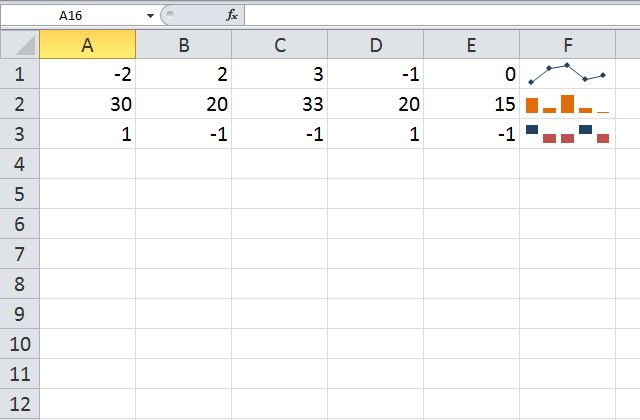Source code for this example:

``````    #!/usr/bin/perl

###############################################################################
#
# Example of how to add sparklines to an Excel::Writer::XLSX file.
#
# Sparklines are small charts that fit in a single cell and are
# used to show trends in data. See sparklines2.pl for examples
# of more complex sparkline formatting.
#
# Copyright 2000-2021, John McNamara, jmcnamara@cpan.org
#

use strict;
use warnings;
use Excel::Writer::XLSX;

my \$workbook  = Excel::Writer::XLSX->new( 'sparklines1.xlsx' );
my \$worksheet = \$workbook->add_worksheet();

# Some sample data to plot.
my \$data = [

[ -2, 2,  3,  -1, 0 ],
[ 30, 20, 33, 20, 15 ],
[ 1,  -1, -1, 1,  -1 ],

];

# Write the sample data to the worksheet.
\$worksheet->write_col( 'A1', \$data );

# Add a line sparkline (the default) with markers.
{
location => 'F1',
range    => 'Sheet1!A1:E1',
markers  => 1,
}
);

# Add a column sparkline with non-default style.
{
location => 'F2',
range    => 'Sheet1!A2:E2',
type     => 'column',
style    => 12,
}
);

# Add a win/loss sparkline with negative values highlighted.
{
location        => 'F3',
range           => 'Sheet1!A3:E3',
type            => 'win_loss',
negative_points => 1,
}
);

\$workbook->close();

__END__``````

## Example: sparklines2.pl

Example of how to add sparklines to an Excel::Writer::XLSX file.

Sparklines are small charts that fit in a single cell and are used to show trends in data. This example shows the majority of options that can be applied to sparklines.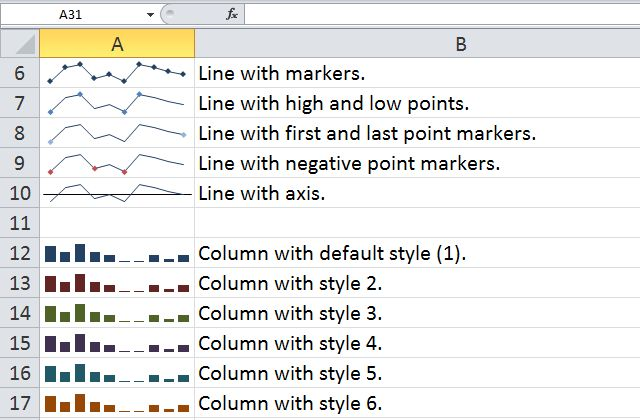Source code for this example:

``````    #!/usr/bin/perl

###############################################################################
#
# Example of how to add sparklines to an Excel::Writer::XLSX file.
#
# Sparklines are small charts that fit in a single cell and are
# used to show trends in data. This example shows the majority of
# options that can be applied to sparklines.
#
# Copyright 2000-2021, John McNamara, jmcnamara@cpan.org
#

use strict;
use warnings;
use Excel::Writer::XLSX;

my \$workbook    = Excel::Writer::XLSX->new( 'sparklines2.xlsx' );
my \$worksheet1  = \$workbook->add_worksheet();
my \$worksheet2  = \$workbook->add_worksheet();
my \$bold        = \$workbook->add_format( bold => 1 );
my \$str;
my \$row = 1;

# Set the columns widths to make the output clearer.
\$worksheet1->set_column( 'A:A', 14 );
\$worksheet1->set_column( 'B:B', 50 );
\$worksheet1->set_zoom( 150 );

\$worksheet1->write( 'A1', 'Sparkline',   \$bold );
\$worksheet1->write( 'B1', 'Description', \$bold );

###############################################################################
#
\$str = 'A default "line" sparkline.';

{
location => 'A2',
range    => 'Sheet2!A1:J1',
}
);

\$worksheet1->write( \$row++, 1, \$str );

###############################################################################
#
\$str = 'A default "column" sparkline.';

{
location => 'A3',
range    => 'Sheet2!A2:J2',
type     => 'column',
}
);

\$worksheet1->write( \$row++, 1, \$str );

###############################################################################
#
\$str = 'A default "win/loss" sparkline.';

{
location => 'A4',
range    => 'Sheet2!A3:J3',
type     => 'win_loss',
}
);

\$worksheet1->write( \$row++, 1, \$str );
\$row++;

###############################################################################
#
\$str = 'Line with markers.';

{
location => 'A6',
range    => 'Sheet2!A1:J1',
markers  => 1,
}
);

\$worksheet1->write( \$row++, 1, \$str );

###############################################################################
#
\$str = 'Line with high and low points.';

{
location   => 'A7',
range      => 'Sheet2!A1:J1',
high_point => 1,
low_point  => 1,
}
);

\$worksheet1->write( \$row++, 1, \$str );

###############################################################################
#
\$str = 'Line with first and last point markers.';

{
location    => 'A8',
range       => 'Sheet2!A1:J1',
first_point => 1,
last_point  => 1,
}
);

\$worksheet1->write( \$row++, 1, \$str );

###############################################################################
#
\$str = 'Line with negative point markers.';

{
location        => 'A9',
range           => 'Sheet2!A1:J1',
negative_points => 1,
}
);

\$worksheet1->write( \$row++, 1, \$str );

###############################################################################
#
\$str = 'Line with axis.';

{
location => 'A10',
range    => 'Sheet2!A1:J1',
axis     => 1,
}
);

\$worksheet1->write( \$row++, 1, \$str );
\$row++;

###############################################################################
#
\$str = 'Column with default style (1).';

{
location => 'A12',
range    => 'Sheet2!A2:J2',
type     => 'column',
}
);

\$worksheet1->write( \$row++, 1, \$str );

###############################################################################
#
\$str = 'Column with style 2.';

{
location => 'A13',
range    => 'Sheet2!A2:J2',
type     => 'column',
style    => 2,
}
);

\$worksheet1->write( \$row++, 1, \$str );

###############################################################################
#
\$str = 'Column with style 3.';

{
location => 'A14',
range    => 'Sheet2!A2:J2',
type     => 'column',
style    => 3,
}
);

\$worksheet1->write( \$row++, 1, \$str );

###############################################################################
#
\$str = 'Column with style 4.';

{
location => 'A15',
range    => 'Sheet2!A2:J2',
type     => 'column',
style    => 4,
}
);

\$worksheet1->write( \$row++, 1, \$str );

###############################################################################
#
\$str = 'Column with style 5.';

{
location => 'A16',
range    => 'Sheet2!A2:J2',
type     => 'column',
style    => 5,
}
);

\$worksheet1->write( \$row++, 1, \$str );

###############################################################################
#
\$str = 'Column with style 6.';

{
location => 'A17',
range    => 'Sheet2!A2:J2',
type     => 'column',
style    => 6,
}
);

\$worksheet1->write( \$row++, 1, \$str );

###############################################################################
#
\$str = 'Column with a user defined colour.';

{
location     => 'A18',
range        => 'Sheet2!A2:J2',
type         => 'column',
series_color => '#E965E0',
}
);

\$worksheet1->write( \$row++, 1, \$str );
\$row++;

###############################################################################
#
\$str = 'A win/loss sparkline.';

{
location => 'A20',
range    => 'Sheet2!A3:J3',
type     => 'win_loss',
}
);

\$worksheet1->write( \$row++, 1, \$str );

###############################################################################
#
\$str = 'A win/loss sparkline with negative points highlighted.';

{
location        => 'A21',
range           => 'Sheet2!A3:J3',
type            => 'win_loss',
negative_points => 1,
}
);

\$worksheet1->write( \$row++, 1, \$str );
\$row++;

###############################################################################
#
\$str = 'A left to right column (the default).';

{
location => 'A23',
range    => 'Sheet2!A4:J4',
type     => 'column',
style    => 20,
}
);

\$worksheet1->write( \$row++, 1, \$str );

###############################################################################
#
\$str = 'A right to left column.';

{
location => 'A24',
range    => 'Sheet2!A4:J4',
type     => 'column',
style    => 20,
reverse  => 1,
}
);

\$worksheet1->write( \$row++, 1, \$str );

###############################################################################
#
\$str = 'Sparkline and text in one cell.';

{
location => 'A25',
range    => 'Sheet2!A4:J4',
type     => 'column',
style    => 20,
}
);

\$worksheet1->write( \$row,   0, 'Growth' );
\$worksheet1->write( \$row++, 1, \$str );
\$row++;

###############################################################################
#
\$str = 'A grouped sparkline. Changes are applied to all three.';

{
location => [ 'A27',          'A28',          'A29' ],
range    => [ 'Sheet2!A5:J5', 'Sheet2!A6:J6', 'Sheet2!A7:J7' ],
markers  => 1,
}
);

\$worksheet1->write( \$row++, 1, \$str );

###############################################################################
#
# Create a second worksheet with data to plot.
#

\$worksheet2->set_column( 'A:J', 11 );

my \$data = [

# Simple line data.
[ -2, 2, 3, -1, 0, -2, 3, 2, 1, 0 ],

# Simple column data.
[ 30, 20, 33, 20, 15, 5, 5, 15, 10, 15 ],

# Simple win/loss data.
[ 1, 1, -1, -1, 1, -1, 1, 1, 1, -1 ],

# Unbalanced histogram.
[ 5, 6, 7, 10, 15, 20, 30, 50, 70, 100 ],

# Data for the grouped sparkline example.
[ -2, 2,  3, -1, 0, -2, 3, 2, 1, 0 ],
[ 3,  -1, 0, -2, 3, 2,  1, 0, 2, 1 ],
[ 0,  -2, 3, 2,  1, 0,  1, 2, 3, 1 ],

];

# Write the sample data to the worksheet.
\$worksheet2->write_col( 'A1', \$data );

\$workbook->close();

__END__``````

## Example: stats_ext.pl

Example of formatting using the Excel::Writer::XLSX module

This is a simple example of how to use functions that reference cells in other worksheets within the same workbook.Source code for this example:

``````    #!/usr/bin/perl -w

###############################################################################
#
# Example of formatting using the Excel::Writer::XLSX module
#
# This is a simple example of how to use functions that reference cells in
# other worksheets within the same workbook.
#
# Copyright 2000-2021, John McNamara, jmcnamara@cpan.org
#

use strict;
use Excel::Writer::XLSX;

# Create a new workbook and add a worksheet
my \$workbook   = Excel::Writer::XLSX->new( 'stats_ext.xlsx' );
my \$worksheet1 = \$workbook->add_worksheet( 'Test results' );
my \$worksheet2 = \$workbook->add_worksheet( 'Data' );

# Set the column width for columns 1
\$worksheet1->set_column( 'A:A', 20 );

# Create a format for the headings

# Create a numerical format
my \$numformat = \$workbook->add_format();
\$numformat->set_num_format( '0.00' );

# Write some statistical functions
\$worksheet1->write( 'A1', 'Count', \$heading );
\$worksheet1->write( 'B1', '=COUNT(Data!B2:B9)' );

\$worksheet1->write( 'A2', 'Sum', \$heading );
\$worksheet1->write( 'B2', '=SUM(Data!B2:B9)' );

\$worksheet1->write( 'A3', 'Average', \$heading );
\$worksheet1->write( 'B3', '=AVERAGE(Data!B2:B9)' );

\$worksheet1->write( 'A4', 'Min', \$heading );
\$worksheet1->write( 'B4', '=MIN(Data!B2:B9)' );

\$worksheet1->write( 'A5', 'Max', \$heading );
\$worksheet1->write( 'B5', '=MAX(Data!B2:B9)' );

\$worksheet1->write( 'A6', 'Standard Deviation', \$heading );
\$worksheet1->write( 'B6', '=STDEV(Data!B2:B9)' );

\$worksheet1->write( 'A7', 'Kurtosis', \$heading );
\$worksheet1->write( 'B7', '=KURT(Data!B2:B9)' );

# Write the sample data
\$worksheet2->write( 'A1', 'Sample', \$heading );
\$worksheet2->write( 'A2', 1 );
\$worksheet2->write( 'A3', 2 );
\$worksheet2->write( 'A4', 3 );
\$worksheet2->write( 'A5', 4 );
\$worksheet2->write( 'A6', 5 );
\$worksheet2->write( 'A7', 6 );
\$worksheet2->write( 'A8', 7 );
\$worksheet2->write( 'A9', 8 );

\$worksheet2->write( 'B1', 'Length', \$heading );
\$worksheet2->write( 'B2', 25.4,     \$numformat );
\$worksheet2->write( 'B3', 25.4,     \$numformat );
\$worksheet2->write( 'B4', 24.8,     \$numformat );
\$worksheet2->write( 'B5', 25.0,     \$numformat );
\$worksheet2->write( 'B6', 25.3,     \$numformat );
\$worksheet2->write( 'B7', 24.9,     \$numformat );
\$worksheet2->write( 'B8', 25.2,     \$numformat );
\$worksheet2->write( 'B9', 24.8,     \$numformat );

\$workbook->close();

__END__``````

## Example: stocks.pl

Example of formatting using the Excel::Writer::XLSX module

This example shows how to use a conditional numerical format with colours to indicate if a share price has gone up or down.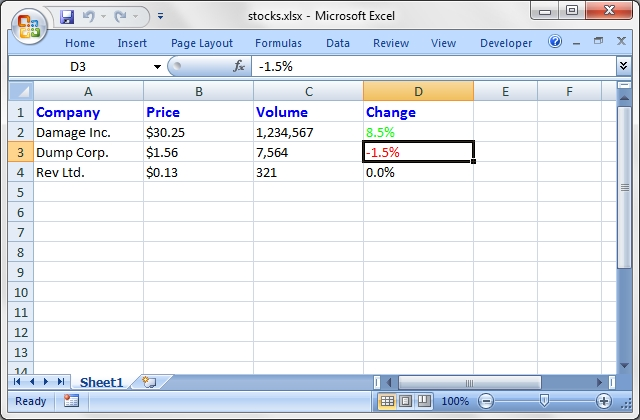Source code for this example:

``````    #!/usr/bin/perl -w

###############################################################################
#
# Example of formatting using the Excel::Writer::XLSX module
#
# This example shows how to use a conditional numerical format
# with colours to indicate if a share price has gone up or down.
#
# Copyright 2000-2021, John McNamara, jmcnamara@cpan.org
#

use strict;
use Excel::Writer::XLSX;

# Create a new workbook and add a worksheet
my \$workbook  = Excel::Writer::XLSX->new( 'stocks.xlsx' );
my \$worksheet = \$workbook->add_worksheet();

# Set the column width for columns 1, 2, 3 and 4
\$worksheet->set_column( 0, 3, 15 );

# Create a format for the column headings

# Create a format for the stock price
my \$f_price = \$workbook->add_format();
\$f_price->set_align( 'left' );
\$f_price->set_num_format( '\$0.00' );

# Create a format for the stock volume
my \$f_volume = \$workbook->add_format();
\$f_volume->set_align( 'left' );
\$f_volume->set_num_format( '#,##0' );

# Create a format for the price change. This is an example of a conditional
# format. The number is formatted as a percentage. If it is positive it is
# formatted in green, if it is negative it is formatted in red and if it is
# zero it is formatted as the default font colour (in this case black).
# Note: the [Green] format produces an unappealing lime green. Try
# [Color 10] instead for a dark green.
#
my \$f_change = \$workbook->add_format();
\$f_change->set_align( 'left' );
\$f_change->set_num_format( '[Green]0.0%;[Red]-0.0%;0.0%' );

# Write out the data
\$worksheet->write( 0, 0, 'Company', \$header );
\$worksheet->write( 0, 1, 'Price',   \$header );
\$worksheet->write( 0, 2, 'Volume',  \$header );
\$worksheet->write( 0, 3, 'Change',  \$header );

\$worksheet->write( 1, 0, 'Damage Inc.' );
\$worksheet->write( 1, 1, 30.25, \$f_price );       # \$30.25
\$worksheet->write( 1, 2, 1234567, \$f_volume );    # 1,234,567
\$worksheet->write( 1, 3, 0.085, \$f_change );      # 8.5% in green

\$worksheet->write( 2, 0, 'Dump Corp.' );
\$worksheet->write( 2, 1, 1.56, \$f_price );        # \$1.56
\$worksheet->write( 2, 2, 7564, \$f_volume );       # 7,564
\$worksheet->write( 2, 3, -0.015, \$f_change );     # -1.5% in red

\$worksheet->write( 3, 0, 'Rev Ltd.' );
\$worksheet->write( 3, 1, 0.13, \$f_price );        # \$0.13
\$worksheet->write( 3, 2, 321, \$f_volume );        # 321
\$worksheet->write( 3, 3, 0, \$f_change );          # 0 in the font color (black)

\$workbook->close();

__END__``````

## Example: background.pl

An example of setting a worksheet background image with Excel::Writer::XLSX.

``````    #!/usr/bin/perl -w

#######################################################################
#
# An example of setting a worksheet background image with Excel::Writer::XLSX.
#
# Copyright 2000-2021, John McNamara, jmcnamara@cpan.org
#

use strict;
use Excel::Writer::XLSX;

my \$workbook   = Excel::Writer::XLSX->new( 'background.xlsx' );
my \$worksheet  = \$workbook->add_worksheet();

\$worksheet->set_background( 'republic.png' );

\$workbook->close();

__END__``````

## Example: tab_colors.pl

Example of how to set Excel worksheet tab colours.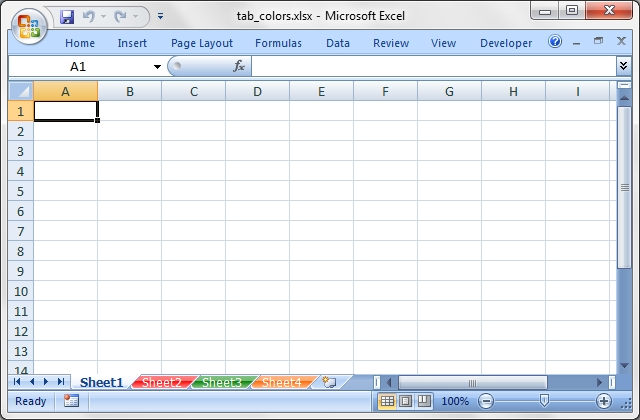Source code for this example:

``````    #!/usr/bin/perl

#######################################################################
#
# Example of how to set Excel worksheet tab colours.
#
# Copyright 2000-2021, John McNamara, jmcnamara@cpan.org
#

use strict;
use warnings;
use Excel::Writer::XLSX;

my \$workbook = Excel::Writer::XLSX->new( 'tab_colors.xlsx' );

my \$worksheet1 = \$workbook->add_worksheet();
my \$worksheet2 = \$workbook->add_worksheet();
my \$worksheet3 = \$workbook->add_worksheet();
my \$worksheet4 = \$workbook->add_worksheet();

# Worksheet1 will have the default tab colour.
\$worksheet2->set_tab_color( 'red' );
\$worksheet3->set_tab_color( 'green' );
\$worksheet4->set_tab_color( '#FF6600'); # Orange

\$workbook->close();

__END__``````

## Example: tables.pl

Example of how to add tables to an Excel::Writer::XLSX worksheet.

Tables in Excel are used to group rows and columns of data into a single structure that can be referenced in a formula or formatted collectively.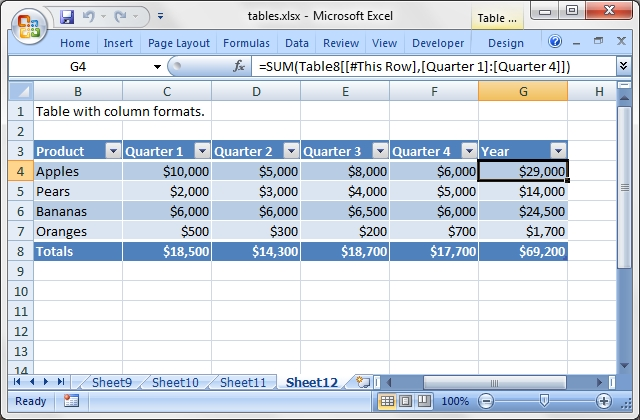Source code for this example:

``````    #!/usr/bin/perl

###############################################################################
#
# Example of how to add tables to an Excel::Writer::XLSX worksheet.
#
# Tables in Excel are used to group rows and columns of data into a single
# structure that can be referenced in a formula or formatted collectively.
#
# Copyright 2000-2021, John McNamara, jmcnamara@cpan.org
#

use strict;
use warnings;
use Excel::Writer::XLSX;

my \$workbook    = Excel::Writer::XLSX->new( 'tables.xlsx' );
my \$worksheet1  = \$workbook->add_worksheet();
my \$worksheet2  = \$workbook->add_worksheet();
my \$worksheet3  = \$workbook->add_worksheet();
my \$worksheet4  = \$workbook->add_worksheet();
my \$worksheet5  = \$workbook->add_worksheet();
my \$worksheet6  = \$workbook->add_worksheet();
my \$worksheet7  = \$workbook->add_worksheet();
my \$worksheet8  = \$workbook->add_worksheet();
my \$worksheet9  = \$workbook->add_worksheet();
my \$worksheet10 = \$workbook->add_worksheet();
my \$worksheet11 = \$workbook->add_worksheet();
my \$worksheet12 = \$workbook->add_worksheet();
my \$worksheet13 = \$workbook->add_worksheet();

my \$currency_format = \$workbook->add_format( num_format => '\$#,##0' );

# Some sample data for the table.
my \$data = [
[ 'Apples',  10000, 5000, 8000, 6000 ],
[ 'Pears',   2000,  3000, 4000, 5000 ],
[ 'Bananas', 6000,  6000, 6500, 6000 ],
[ 'Oranges', 500,   300,  200,  700 ],

];

###############################################################################
#
# Example 1.
#
my \$caption = 'Default table with no data.';

# Set the columns widths.
\$worksheet1->set_column( 'B:G', 12 );

# Write the caption.
\$worksheet1->write( 'B1', \$caption );

# Add a table to the worksheet.

###############################################################################
#
# Example 2.
#
\$caption = 'Default table with data.';

# Set the columns widths.
\$worksheet2->set_column( 'B:G', 12 );

# Write the caption.
\$worksheet2->write( 'B1', \$caption );

# Add a table to the worksheet.
\$worksheet2->add_table( 'B3:F7', { data => \$data } );

###############################################################################
#
# Example 3.
#
\$caption = 'Table without default autofilter.';

# Set the columns widths.
\$worksheet3->set_column( 'B:G', 12 );

# Write the caption.
\$worksheet3->write( 'B1', \$caption );

# Add a table to the worksheet.
\$worksheet3->add_table( 'B3:F7', { autofilter => 0 } );

# Table data can also be written separately, as an array or individual cells.
\$worksheet3->write_col( 'B4', \$data );

###############################################################################
#
# Example 4.
#
\$caption = 'Table without default header row.';

# Set the columns widths.
\$worksheet4->set_column( 'B:G', 12 );

# Write the caption.
\$worksheet4->write( 'B1', \$caption );

# Add a table to the worksheet.
\$worksheet4->add_table( 'B4:F7', { header_row => 0 } );

# Table data can also be written separately, as an array or individual cells.
\$worksheet4->write_col( 'B4', \$data );

###############################################################################
#
# Example 5.
#
\$caption = 'Default table with "First Column" and "Last Column" options.';

# Set the columns widths.
\$worksheet5->set_column( 'B:G', 12 );

# Write the caption.
\$worksheet5->write( 'B1', \$caption );

# Add a table to the worksheet.
\$worksheet5->add_table( 'B3:F7', { first_column => 1, last_column => 1 } );

# Table data can also be written separately, as an array or individual cells.
\$worksheet5->write_col( 'B4', \$data );

###############################################################################
#
# Example 6.
#
\$caption = 'Table with banded columns but without default banded rows.';

# Set the columns widths.
\$worksheet6->set_column( 'B:G', 12 );

# Write the caption.
\$worksheet6->write( 'B1', \$caption );

# Add a table to the worksheet.
\$worksheet6->add_table( 'B3:F7', { banded_rows => 0, banded_columns => 1 } );

# Table data can also be written separately, as an array or individual cells.
\$worksheet6->write_col( 'B4', \$data );

###############################################################################
#
# Example 7.
#
\$caption = 'Table with user defined column headers';

# Set the columns widths.
\$worksheet7->set_column( 'B:G', 12 );

# Write the caption.
\$worksheet7->write( 'B1', \$caption );

# Add a table to the worksheet.
'B3:F7',
{
data    => \$data,
columns => [
{ header => 'Product' },
{ header => 'Quarter 1' },
{ header => 'Quarter 2' },
{ header => 'Quarter 3' },
{ header => 'Quarter 4' },
]
}
);

###############################################################################
#
# Example 8.
#
\$caption = 'Table with user defined column headers';

# Set the columns widths.
\$worksheet8->set_column( 'B:G', 12 );

# Write the caption.
\$worksheet8->write( 'B1', \$caption );

# Add a table to the worksheet.
'B3:G7',
{
data    => \$data,
columns => [
{ header => 'Product' },
{ header => 'Quarter 1' },
{ header => 'Quarter 2' },
{ header => 'Quarter 3' },
{ header => 'Quarter 4' },
{
formula => '=SUM(Table8[@[Quarter 1]:[Quarter 4]])'
},
]
}
);

###############################################################################
#
# Example 9.
#
\$caption = 'Table with totals row (but no caption or totals).';

# Set the columns widths.
\$worksheet9->set_column( 'B:G', 12 );

# Write the caption.
\$worksheet9->write( 'B1', \$caption );

# Add a table to the worksheet.
'B3:G8',
{
data      => \$data,
total_row => 1,
columns   => [
{ header => 'Product' },
{ header => 'Quarter 1' },
{ header => 'Quarter 2' },
{ header => 'Quarter 3' },
{ header => 'Quarter 4' },
{
formula => '=SUM(Table9[@[Quarter 1]:[Quarter 4]])'
},
]
}
);

###############################################################################
#
# Example 10.
#
\$caption = 'Table with totals row with user captions and functions.';

# Set the columns widths.
\$worksheet10->set_column( 'B:G', 12 );

# Write the caption.
\$worksheet10->write( 'B1', \$caption );

# Add a table to the worksheet.
'B3:G8',
{
data      => \$data,
total_row => 1,
columns   => [
{ header => 'Product',   total_string   => 'Totals' },
{ header => 'Quarter 1', total_function => 'sum' },
{ header => 'Quarter 2', total_function => 'sum' },
{ header => 'Quarter 3', total_function => 'sum' },
{ header => 'Quarter 4', total_function => 'sum' },
{
formula        => '=SUM(Table10[@[Quarter 1]:[Quarter 4]])',
total_function => 'sum'
},
]
}
);

###############################################################################
#
# Example 11.
#
\$caption = 'Table with alternative Excel style.';

# Set the columns widths.
\$worksheet11->set_column( 'B:G', 12 );

# Write the caption.
\$worksheet11->write( 'B1', \$caption );

# Add a table to the worksheet.
'B3:G8',
{
data      => \$data,
style     => 'Table Style Light 11',
total_row => 1,
columns   => [
{ header => 'Product',   total_string   => 'Totals' },
{ header => 'Quarter 1', total_function => 'sum' },
{ header => 'Quarter 2', total_function => 'sum' },
{ header => 'Quarter 3', total_function => 'sum' },
{ header => 'Quarter 4', total_function => 'sum' },
{
formula        => '=SUM(Table11[@[Quarter 1]:[Quarter 4]])',
total_function => 'sum'
},
]
}
);

###############################################################################
#
# Example 12.
#
\$caption = 'Table with no Excel style.';

# Set the columns widths.
\$worksheet12->set_column( 'B:G', 12 );

# Write the caption.
\$worksheet12->write( 'B1', \$caption );

# Add a table to the worksheet.
'B3:G8',
{
data      => \$data,
style     => 'None',
total_row => 1,
columns   => [
{ header => 'Product',   total_string   => 'Totals' },
{ header => 'Quarter 1', total_function => 'sum' },
{ header => 'Quarter 2', total_function => 'sum' },
{ header => 'Quarter 3', total_function => 'sum' },
{ header => 'Quarter 4', total_function => 'sum' },
{
formula        => '=SUM(Table12[@[Quarter 1]:[Quarter 4]])',
total_function => 'sum'
},
]
}
);

###############################################################################
#
# Example 13.
#
\$caption = 'Table with column formats.';

# Set the columns widths.
\$worksheet13->set_column( 'B:G', 12 );

# Write the caption.
\$worksheet13->write( 'B1', \$caption );

# Add a table to the worksheet.
'B3:G8',
{
data      => \$data,
total_row => 1,
columns   => [
{ header => 'Product', total_string => 'Totals' },
{
header         => 'Quarter 1',
total_function => 'sum',
format         => \$currency_format,
},
{
header         => 'Quarter 2',
total_function => 'sum',
format         => \$currency_format,
},
{
header         => 'Quarter 3',
total_function => 'sum',
format         => \$currency_format,
},
{
header         => 'Quarter 4',
total_function => 'sum',
format         => \$currency_format,
},
{
formula        => '=SUM(Table13[@[Quarter 1]:[Quarter 4]])',
total_function => 'sum',
format         => \$currency_format,
},
]
}
);

\$workbook->close();

__END__

``````

## Example: write_handler1.pl

Example of how to add a user defined data handler to the Excel::Writer::XLSX write() method.

The following example shows how to add a handler for a 7 digit ID number.Source code for this example:

``````    #!/usr/bin/perl -w

###############################################################################
#
# Example of how to add a user defined data handler to the
# Excel::Writer::XLSX write() method.
#
# The following example shows how to add a handler for a 7 digit ID number.
#
#
# Copyright 2000-2021, John McNamara, jmcnamara@cpan.org
#

use strict;
use Excel::Writer::XLSX;

my \$workbook  = Excel::Writer::XLSX->new( 'write_handler1.xlsx' );
my \$worksheet = \$workbook->add_worksheet();

###############################################################################
#
# Add a handler for 7 digit id numbers. This is useful when you want a string
# such as 0000001 written as a string instead of a number and thus preserve
# the leading zeroes.
#
# Note: you can get the same effect using the keep_leading_zeros() method but
# this serves as a simple example.
#
\$worksheet->add_write_handler( qr[^\d{7}\$], \&write_my_id );

###############################################################################
#
# The following function processes the data when a match is found.
#
sub write_my_id {

my \$worksheet = shift;

return \$worksheet->write_string( @_ );
}

# This format maintains the cell as text even if it is edited.
my \$id_format = \$workbook->add_format( num_format => '@' );

# Write some numbers in the user defined format
\$worksheet->write( 'A1', '0000000', \$id_format );
\$worksheet->write( 'A2', '0000001', \$id_format );
\$worksheet->write( 'A3', '0004000', \$id_format );
\$worksheet->write( 'A4', '1234567', \$id_format );

# Write some numbers that don't match the defined format
\$worksheet->write( 'A6', '000000', \$id_format );
\$worksheet->write( 'A7', '000001', \$id_format );
\$worksheet->write( 'A8', '004000', \$id_format );
\$worksheet->write( 'A9', '123456', \$id_format );

\$workbook->close();

__END__
``````

## Example: write_handler2.pl

Example of how to add a user defined data handler to the Excel::Writer::XLSX write() method.

The following example shows how to add a handler for a 7 digit ID number. It adds an additional constraint to the write_handler1.pl in that it only filters data that isn't in the third column.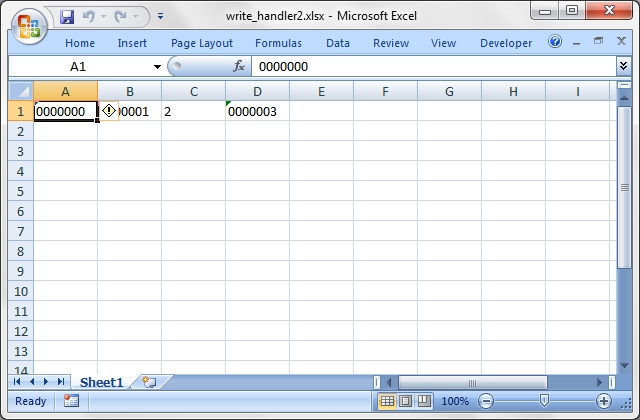Source code for this example:

``````    #!/usr/bin/perl -w

###############################################################################
#
# Example of how to add a user defined data handler to the
# Excel::Writer::XLSX write() method.
#
# The following example shows how to add a handler for a 7 digit ID number.
# It adds an additional constraint to the write_handler1.pl in that it only
# filters data that isn't in the third column.
#
#
# Copyright 2000-2021, John McNamara, jmcnamara@cpan.org
#

use strict;
use Excel::Writer::XLSX;

my \$workbook  = Excel::Writer::XLSX->new( 'write_handler2.xlsx' );
my \$worksheet = \$workbook->add_worksheet();

###############################################################################
#
# Add a handler for 7 digit id numbers. This is useful when you want a string
# such as 0000001 written as a string instead of a number and thus preserve
# the leading zeroes.
#
# Note: you can get the same effect using the keep_leading_zeros() method but
# this serves as a simple example.
#
\$worksheet->add_write_handler( qr[^\d{7}\$], \&write_my_id );

###############################################################################
#
# The following function processes the data when a match is found. The handler
# is set up so that it only filters data if it is in the third column.
#
sub write_my_id {

my \$worksheet = shift;
my \$col       = \$_;

# col is zero based
if ( \$col != 2 ) {
return \$worksheet->write_string( @_ );
}
else {

# Reject the match and return control to write()
return undef;
}

}

# This format maintains the cell as text even if it is edited.
my \$id_format = \$workbook->add_format( num_format => '@' );

# Write some numbers in the user defined format
\$worksheet->write( 'A1', '0000000', \$id_format );
\$worksheet->write( 'B1', '0000001', \$id_format );
\$worksheet->write( 'C1', '0000002', \$id_format );
\$worksheet->write( 'D1', '0000003', \$id_format );

\$workbook->close();

__END__
``````

## Example: write_handler3.pl

Example of how to add a user defined data handler to the Excel::Writer::XLSX write() method.

The following example shows how to add a handler for dates in a specific format.

See write_handler4.pl for a more rigorous example with error handling.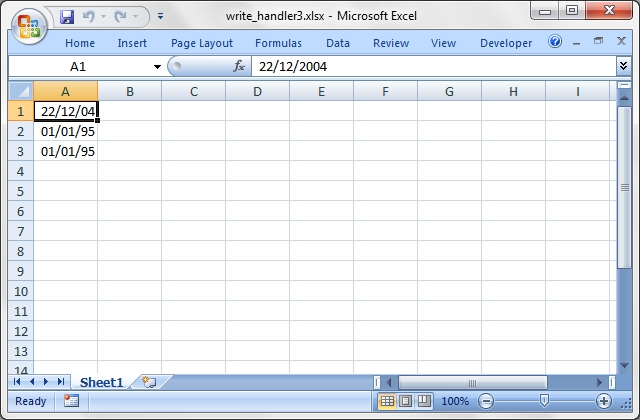Source code for this example:

``````    #!/usr/bin/perl -w

###############################################################################
#
# Example of how to add a user defined data handler to the
# Excel::Writer::XLSX write() method.
#
# The following example shows how to add a handler for dates in a specific
# format.
#
# See write_handler4.pl for a more rigorous example with error handling.
#
# Copyright 2000-2021, John McNamara, jmcnamara@cpan.org
#

use strict;
use Excel::Writer::XLSX;

my \$workbook    = Excel::Writer::XLSX->new( 'write_handler3.xlsx' );
my \$worksheet   = \$workbook->add_worksheet();
my \$date_format = \$workbook->add_format( num_format => 'dd/mm/yy' );

###############################################################################
#
# Add a handler to match dates in the following format: d/m/yyyy
#
# The day and month can be single or double digits.
#
\$worksheet->add_write_handler( qr[^\d{1,2}/\d{1,2}/\d{4}\$], \&write_my_date );

###############################################################################
#
# The following function processes the data when a match is found.
# See write_handler4.pl for a more rigorous example with error handling.
#
sub write_my_date {

my \$worksheet = shift;
my @args      = @_;

my \$token = \$args;
\$token =~ qr[^(\d{1,2})/(\d{1,2})/(\d{4})\$];

# Change to the date format required by write_date_time().
my \$date = sprintf "%4d-%02d-%02dT", \$3, \$2, \$1;

\$args = \$date;

return \$worksheet->write_date_time( @args );
}

# Write some dates in the user defined format
\$worksheet->write( 'A1', '22/12/2004', \$date_format );
\$worksheet->write( 'A2', '1/1/1995',   \$date_format );
\$worksheet->write( 'A3', '01/01/1995', \$date_format );

\$workbook->close();

__END__
``````

## Example: write_handler4.pl

Example of how to add a user defined data handler to the Excel::Writer::XLSX write() method.

The following example shows how to add a handler for dates in a specific format.

This is a more rigorous version of write_handler3.pl.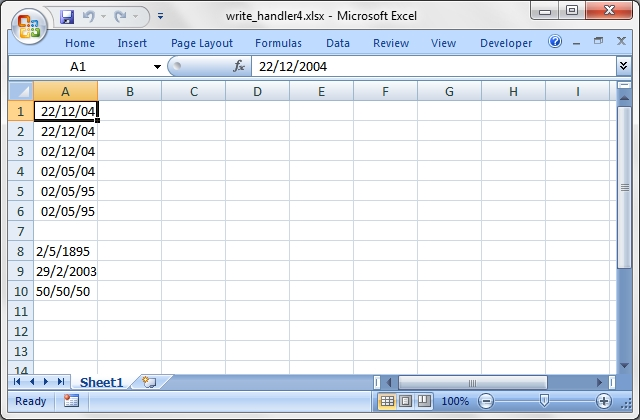Source code for this example:

``````    #!/usr/bin/perl -w

###############################################################################
#
# Example of how to add a user defined data handler to the
# Excel::Writer::XLSX write() method.
#
# The following example shows how to add a handler for dates in a specific
# format.
#
# This is a more rigorous version of write_handler3.pl.
#
# Copyright 2000-2021, John McNamara, jmcnamara@cpan.org
#

use strict;
use Excel::Writer::XLSX;

my \$workbook    = Excel::Writer::XLSX->new( 'write_handler4.xlsx' );
my \$worksheet   = \$workbook->add_worksheet();
my \$date_format = \$workbook->add_format( num_format => 'dd/mm/yy' );

###############################################################################
#
# Add a handler to match dates in the following formats: d/m/yy, d/m/yyyy
#
# The day and month can be single or double digits and the year can be  2 or 4
# digits.
#
\$worksheet->add_write_handler( qr[^\d{1,2}/\d{1,2}/\d{2,4}\$], \&write_my_date );

###############################################################################
#
# The following function processes the data when a match is found.
#
sub write_my_date {

my \$worksheet = shift;
my @args      = @_;

my \$token = \$args;

if ( \$token =~ qr[^(\d{1,2})/(\d{1,2})/(\d{2,4})\$] ) {

my \$day  = \$1;
my \$mon  = \$2;
my \$year = \$3;

# Use a window for 2 digit dates. This will keep some ragged Perl
# programmer employed in thirty years time. :-)
if ( length \$year == 2 ) {
if ( \$year < 50 ) {
\$year += 2000;
}
else {
\$year += 1900;
}
}

my \$date = sprintf "%4d-%02d-%02dT", \$year, \$mon, \$day;

# Convert the ISO ISO8601 style string to an Excel date
\$date = \$worksheet->convert_date_time( \$date );

if ( defined \$date ) {

# Date was valid
\$args = \$date;
return \$worksheet->write_number( @args );
}
else {

# Not a valid date therefore write as a string
return \$worksheet->write_string( @args );
}
}
else {

# Shouldn't happen if the same match is used in the re and sub.
return undef;
}
}

# Write some dates in the user defined format
\$worksheet->write( 'A1', '22/12/2004', \$date_format );
\$worksheet->write( 'A2', '22/12/04',   \$date_format );
\$worksheet->write( 'A3', '2/12/04',    \$date_format );
\$worksheet->write( 'A4', '2/5/04',     \$date_format );
\$worksheet->write( 'A5', '2/5/95',     \$date_format );
\$worksheet->write( 'A6', '2/5/1995',   \$date_format );

# Some erroneous dates
\$worksheet->write( 'A8', '2/5/1895',  \$date_format ); # Date out of Excel range
\$worksheet->write( 'A9', '29/2/2003', \$date_format ); # Invalid leap day
\$worksheet->write( 'A10', '50/50/50', \$date_format ); # Matches but isn't a date

\$workbook->close();

__END__
``````

## Example: write_to_scalar.pl

An example of writing an Excel::Writer::XLSX file to a perl scalar.

``````    #!/usr/bin/perl

##############################################################################
#
# An example of writing an Excel::Writer::XLSX file to a perl scalar.
#
# Copyright 2000-2021, John McNamara, jmcnamara@cpan.org
#

use strict;
use warnings;
use Excel::Writer::XLSX;

# Use a scalar as a filehandle.
open my \$fh, '>', \my \$str or die "Failed to open filehandle: \$!";

# Spreadsheet::WriteExce accepts filehandle as well as file names.
my \$workbook  = Excel::Writer::XLSX->new( \$fh );
my \$worksheet = \$workbook->add_worksheet();

\$worksheet->write( 0, 0, 'Hi Excel!' );

\$workbook->close();

# The Excel file in now in \$str. Remember to binmode() the output
# filehandle before printing it.
open my \$out_fh, '>', 'write_to_scalar.xlsx'
or die "Failed to open out filehandle: \$!";

binmode \$out_fh;
print   \$out_fh \$str;
close   \$out_fh;

__END__
``````

## Example: unicode_2022_jp.pl

A simple example of converting some Unicode text to an Excel file using Excel::Writer::XLSX.

This example generates some Japanese from a file with ISO-2022-JP encoded text.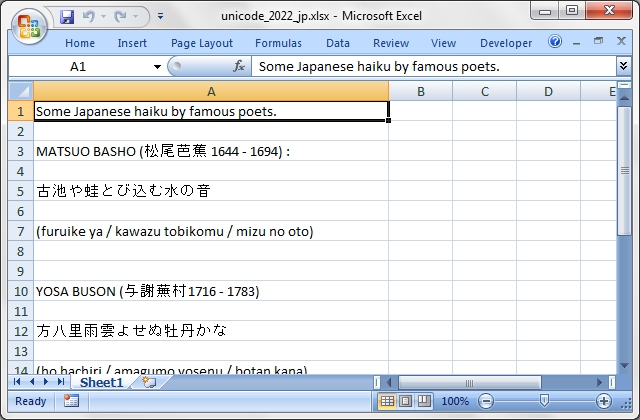Source code for this example:

``````    #!/usr/bin/perl

##############################################################################
#
# A simple example of converting some Unicode text to an Excel file using
# Excel::Writer::XLSX.
#
# This example generates some Japanese from a file with ISO-2022-JP
# encoded text.
#
# Copyright 2000-2021, John McNamara, jmcnamara@cpan.org
#

use strict;
use warnings;
use Excel::Writer::XLSX;

my \$workbook = Excel::Writer::XLSX->new( 'unicode_2022_jp.xlsx' );

die "Couldn't create new Excel file: \$!.\n" unless defined \$workbook;

my \$worksheet = \$workbook->add_worksheet();
\$worksheet->set_column( 'A:A', 50 );

my \$file = 'unicode_2022_jp.txt';

open FH, '<:encoding(iso-2022-jp)', \$file or die "Couldn't open \$file: \$!\n";

my \$row = 0;

while ( <FH> ) {
next if /^#/;    # Ignore the comments in the sample file.
chomp;
\$worksheet->write( \$row++, 0, \$_ );
}

\$workbook->close();

__END__
``````

## Example: unicode_8859_11.pl

A simple example of converting some Unicode text to an Excel file using Excel::Writer::XLSX.

This example generates some Thai from a file with ISO-8859-11 encoded text.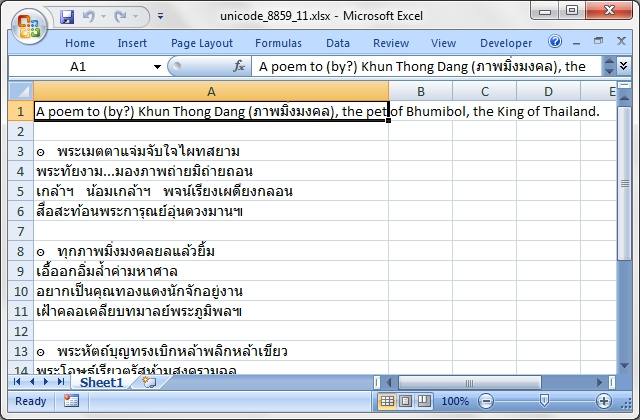Source code for this example:

``````    #!/usr/bin/perl

##############################################################################
#
# A simple example of converting some Unicode text to an Excel file using
# Excel::Writer::XLSX.
#
# This example generates some Thai from a file with ISO-8859-11 encoded text.
#
#
# Copyright 2000-2021, John McNamara, jmcnamara@cpan.org
#

use strict;
use warnings;
use Excel::Writer::XLSX;

my \$workbook = Excel::Writer::XLSX->new( 'unicode_8859_11.xlsx' );

die "Couldn't create new Excel file: \$!.\n" unless defined \$workbook;

my \$worksheet = \$workbook->add_worksheet();
\$worksheet->set_column( 'A:A', 50 );

my \$file = 'unicode_8859_11.txt';

open FH, '<:encoding(iso-8859-11)', \$file or die "Couldn't open \$file: \$!\n";

my \$row = 0;

while ( <FH> ) {
next if /^#/;    # Ignore the comments in the sample file.
chomp;
\$worksheet->write( \$row++, 0, \$_ );
}

\$workbook->close();

__END__
``````

## Example: unicode_8859_7.pl

A simple example of converting some Unicode text to an Excel file using Excel::Writer::XLSX.

This example generates some Greek from a file with ISO-8859-7 encoded text.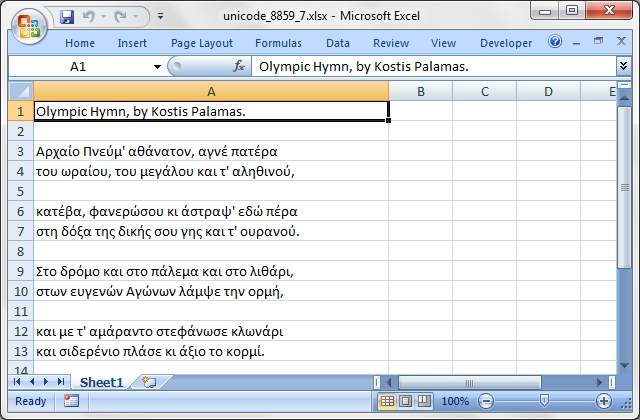Source code for this example:

``````    #!/usr/bin/perl

##############################################################################
#
# A simple example of converting some Unicode text to an Excel file using
# Excel::Writer::XLSX.
#
# This example generates some Greek from a file with ISO-8859-7 encoded text.
#
#
# Copyright 2000-2021, John McNamara, jmcnamara@cpan.org
#

use strict;
use warnings;
use Excel::Writer::XLSX;

my \$workbook = Excel::Writer::XLSX->new( 'unicode_8859_7.xlsx' );

die "Couldn't create new Excel file: \$!.\n" unless defined \$workbook;

my \$worksheet = \$workbook->add_worksheet();
\$worksheet->set_column( 'A:A', 50 );

my \$file = 'unicode_8859_7.txt';

open FH, '<:encoding(iso-8859-7)', \$file or die "Couldn't open \$file: \$!\n";

my \$row = 0;

while ( <FH> ) {
next if /^#/;    # Ignore the comments in the sample file.
chomp;
\$worksheet->write( \$row++, 0, \$_ );
}

\$workbook->close();

__END__
``````

## Example: unicode_big5.pl

A simple example of converting some Unicode text to an Excel file using Excel::Writer::XLSX.

This example generates some Chinese from a file with BIG5 encoded text.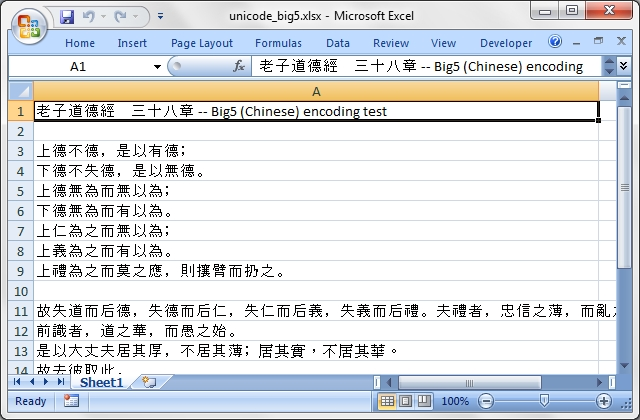Source code for this example:

``````    #!/usr/bin/perl

##############################################################################
#
# A simple example of converting some Unicode text to an Excel file using
# Excel::Writer::XLSX.
#
# This example generates some Chinese from a file with BIG5 encoded text.
#
#
# Copyright 2000-2021, John McNamara, jmcnamara@cpan.org
#

use strict;
use warnings;
use Excel::Writer::XLSX;

my \$workbook = Excel::Writer::XLSX->new( 'unicode_big5.xlsx' );

die "Couldn't create new Excel file: \$!.\n" unless defined \$workbook;

my \$worksheet = \$workbook->add_worksheet();
\$worksheet->set_column( 'A:A', 80 );

my \$file = 'unicode_big5.txt';

open FH, '<:encoding(big5)', \$file or die "Couldn't open \$file: \$!\n";

my \$row = 0;

while ( <FH> ) {
next if /^#/;    # Ignore the comments in the sample file.
chomp;
\$worksheet->write( \$row++, 0, \$_ );
}

\$workbook->close();

__END__
``````

## Example: unicode_cp1251.pl

A simple example of converting some Unicode text to an Excel file using Excel::Writer::XLSX.

This example generates some Russian from a file with CP1251 encoded text.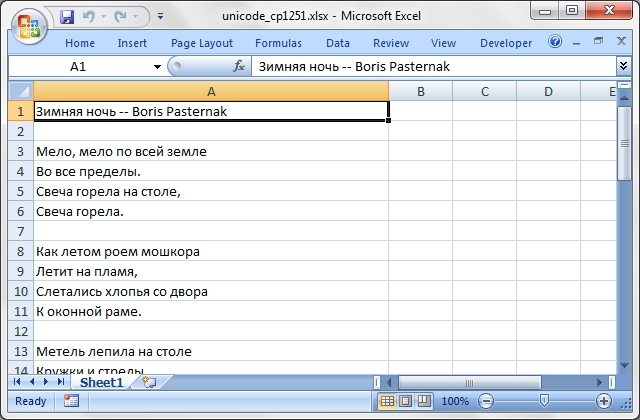Source code for this example:

``````    #!/usr/bin/perl

##############################################################################
#
# A simple example of converting some Unicode text to an Excel file using
# Excel::Writer::XLSX.
#
# This example generates some Russian from a file with CP1251 encoded text.
#
#
# Copyright 2000-2021, John McNamara, jmcnamara@cpan.org
#

use strict;
use warnings;
use Excel::Writer::XLSX;

my \$workbook = Excel::Writer::XLSX->new( 'unicode_cp1251.xlsx' );

die "Couldn't create new Excel file: \$!.\n" unless defined \$workbook;

my \$worksheet = \$workbook->add_worksheet();
\$worksheet->set_column( 'A:A', 50 );

my \$file = 'unicode_cp1251.txt';

open FH, '<:encoding(cp1251)', \$file or die "Couldn't open \$file: \$!\n";

my \$row = 0;

while ( <FH> ) {
next if /^#/;    # Ignore the comments in the sample file.
chomp;
\$worksheet->write( \$row++, 0, \$_ );
}

\$workbook->close();

__END__
``````

## Example: unicode_cp1256.pl

A simple example of converting some Unicode text to an Excel file using Excel::Writer::XLSX.

This example generates some Arabic text from a CP-1256 encoded file.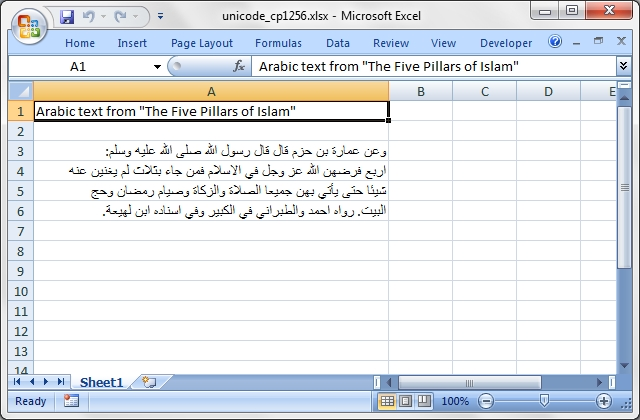Source code for this example:

``````    #!/usr/bin/perl

##############################################################################
#
# A simple example of converting some Unicode text to an Excel file using
# Excel::Writer::XLSX.
#
# This example generates some Arabic text from a CP-1256 encoded file.
#
#
# Copyright 2000-2021, John McNamara, jmcnamara@cpan.org
#

use strict;
use warnings;
use Excel::Writer::XLSX;

my \$workbook = Excel::Writer::XLSX->new( 'unicode_cp1256.xlsx' );

die "Couldn't create new Excel file: \$!.\n" unless defined \$workbook;

my \$worksheet = \$workbook->add_worksheet();
\$worksheet->set_column( 'A:A', 50 );

my \$file = 'unicode_cp1256.txt';

open FH, '<:encoding(cp1256)', \$file or die "Couldn't open \$file: \$!\n";

my \$row = 0;

while ( <FH> ) {
next if /^#/;    # Ignore the comments in the sample file.
chomp;
\$worksheet->write( \$row++, 0, \$_ );
}

\$workbook->close();

__END__
``````

## Example: unicode_cyrillic.pl

A simple example of writing some Russian cyrillic text using Excel::Writer::XLSX.Source code for this example:

``````    #!/usr/bin/perl

##############################################################################
#
# A simple example of writing some Russian cyrillic text using
# Excel::Writer::XLSX.
#
#
#
#
# Copyright 2000-2021, John McNamara, jmcnamara@cpan.org
#

use strict;
use warnings;
use Excel::Writer::XLSX;

# In this example we generate utf8 strings from character data but in a
# real application we would expect them to come from an external source.
#

# Create a Russian worksheet name in utf8.
my \$sheet = pack "U*", 0x0421, 0x0442, 0x0440, 0x0430, 0x043D, 0x0438,
0x0446, 0x0430;

# Create a Russian string.
my \$str = pack "U*", 0x0417, 0x0434, 0x0440, 0x0430, 0x0432, 0x0441,
0x0442, 0x0432, 0x0443, 0x0439, 0x0020, 0x041C,
0x0438, 0x0440, 0x0021;

my \$workbook = Excel::Writer::XLSX->new( 'unicode_cyrillic.xlsx' );

die "Couldn't create new Excel file: \$!.\n" unless defined \$workbook;

my \$worksheet = \$workbook->add_worksheet( \$sheet . '1' );

\$worksheet->set_column( 'A:A', 18 );
\$worksheet->write( 'A1', \$str );

\$workbook->close();

__END__
``````

## Example: unicode_koi8r.pl

A simple example of converting some Unicode text to an Excel file using Excel::Writer::XLSX.

This example generates some Russian from a file with KOI8-R encoded text.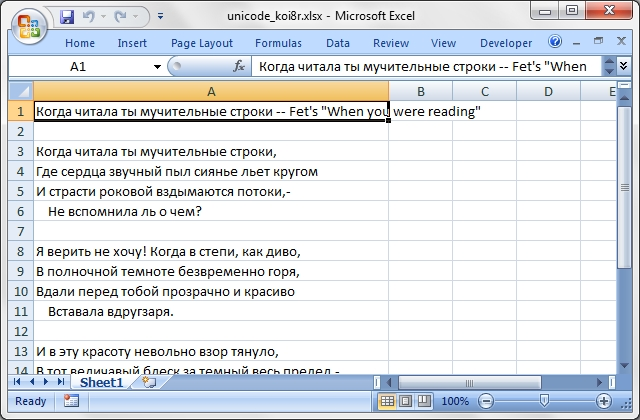Source code for this example:

``````    #!/usr/bin/perl

##############################################################################
#
# A simple example of converting some Unicode text to an Excel file using
# Excel::Writer::XLSX.
#
# This example generates some Russian from a file with KOI8-R encoded text.
#
#
# Copyright 2000-2021, John McNamara, jmcnamara@cpan.org
#

use strict;
use warnings;
use Excel::Writer::XLSX;

my \$workbook = Excel::Writer::XLSX->new( 'unicode_koi8r.xlsx' );

die "Couldn't create new Excel file: \$!.\n" unless defined \$workbook;

my \$worksheet = \$workbook->add_worksheet();
\$worksheet->set_column( 'A:A', 50 );

my \$file = 'unicode_koi8r.txt';

open FH, '<:encoding(koi8-r)', \$file or die "Couldn't open \$file: \$!\n";

my \$row = 0;

while ( <FH> ) {
next if /^#/;    # Ignore the comments in the sample file.
chomp;
\$worksheet->write( \$row++, 0, \$_ );
}

\$workbook->close();

__END__
``````

## Example: unicode_polish_utf8.pl

A simple example of converting some Unicode text to an Excel file using Excel::Writer::XLSX.

This example generates some Polish from a file with UTF8 encoded text.Source code for this example:

``````    #!/usr/bin/perl

##############################################################################
#
# A simple example of converting some Unicode text to an Excel file using
# Excel::Writer::XLSX.
#
# This example generates some Polish from a file with UTF8 encoded text.
#
#
# Copyright 2000-2021, John McNamara, jmcnamara@cpan.org
#

use strict;
use warnings;
use Excel::Writer::XLSX;

my \$workbook = Excel::Writer::XLSX->new( 'unicode_polish_utf8.xlsx' );

die "Couldn't create new Excel file: \$!.\n" unless defined \$workbook;

my \$worksheet = \$workbook->add_worksheet();
\$worksheet->set_column( 'A:A', 50 );

my \$file = 'unicode_polish_utf8.txt';

open FH, '<:encoding(utf8)', \$file or die "Couldn't open \$file: \$!\n";

my \$row = 0;

while ( <FH> ) {
next if /^#/;    # Ignore the comments in the sample file.
chomp;
\$worksheet->write( \$row++, 0, \$_ );
}

\$workbook->close();

__END__
``````

## Example: unicode_shift_jis.pl

A simple example of converting some Unicode text to an Excel file using Excel::Writer::XLSX.

This example generates some Japenese text from a file with Shift-JIS encoded text.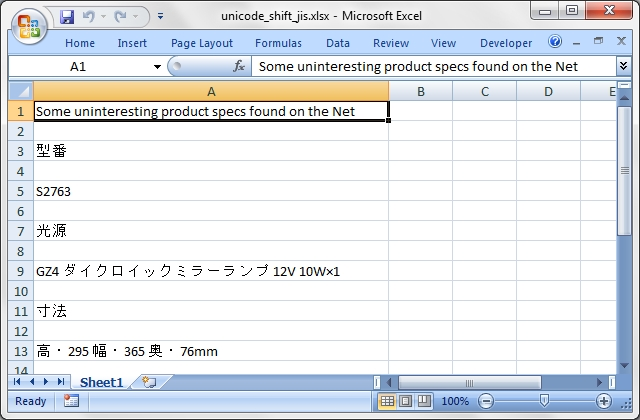Source code for this example:

``````    #!/usr/bin/perl

##############################################################################
#
# A simple example of converting some Unicode text to an Excel file using
# Excel::Writer::XLSX.
#
# This example generates some Japenese text from a file with Shift-JIS
# encoded text.
#
# Copyright 2000-2021, John McNamara, jmcnamara@cpan.org
#

use strict;
use warnings;
use Excel::Writer::XLSX;

my \$workbook = Excel::Writer::XLSX->new( 'unicode_shift_jis.xlsx' );

die "Couldn't create new Excel file: \$!.\n" unless defined \$workbook;

my \$worksheet = \$workbook->add_worksheet();
\$worksheet->set_column( 'A:A', 50 );

my \$file = 'unicode_shift_jis.txt';

open FH, '<:encoding(shiftjis)', \$file or die "Couldn't open \$file: \$!\n";

my \$row = 0;

while ( <FH> ) {
next if /^#/;    # Ignore the comments in the sample file.
chomp;
\$worksheet->write( \$row++, 0, \$_ );
}

\$workbook->close();

__END__
``````

# AUTHOR

John McNamara jmcnamara@cpan.org

Contributed examples contain the original author's name.

Copyright 2000-2021, John McNamara.

All Rights Reserved. This module is free software. It may be used, redistributed and/or modified under the same terms as Perl itself.